# Faster than Light in Our Model of Physics: Some Preliminary Thoughts

When the NASA Innovative Advanced Concepts Program asked me to keynote their annual conference I thought it would be a good excuse to spend some time on a question I’ve always wanted to explore…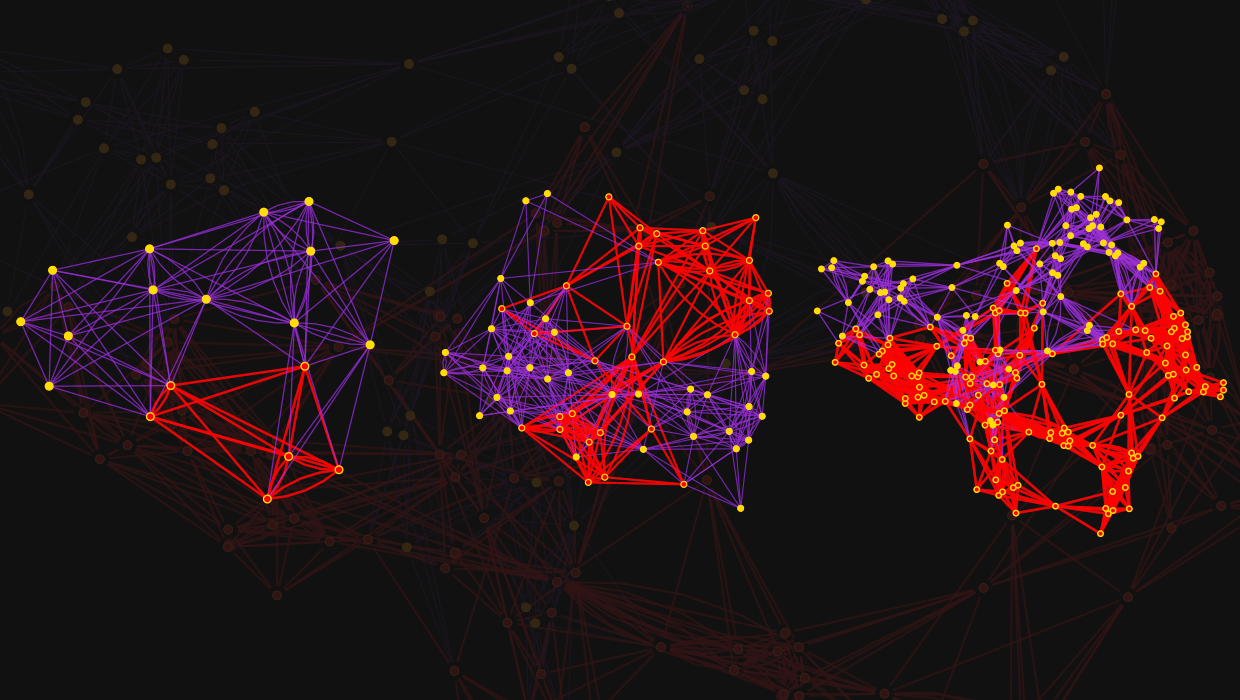## Can You Build a Warp Drive?

“So you think you have a fundamental theory of physics. Well, then tell us if warp drive is possible!” Despite the hopes and assumptions of science fiction, real physics has for at least a century almost universally assumed that no genuine effect can ever propagate through physical space any faster than light. But is this actually true? We’re now in a position to analyze this in the context of our model for fundamental physics. And I’ll say at the outset that it’s a subtle and complicated question, and I don’t know the full answer yet.

But I increasingly suspect that going faster than light is not a physical impossibility; instead, in a sense, doing it is “just” an engineering problem. But it may well be an irreducibly hard engineering problem. And one that can’t be solved with the computational resources available to us in our universe. But it’s also conceivable that there may be some clever “engineering solution”, as there have been to so many seemingly insuperable engineering problems in the past. And that in fact there is a way to “move through space” faster than light.

It’s a little tricky even to define what it means to “go faster than light”. Do we allow an existing “space tunnel” (like the wormholes of general relativity)? Perhaps a space tunnel that has been there since the beginning of the universe. Or even if no space tunnel already exists, do we allow the possibility of building one—that we can then travel through? I’ll discuss these possibilities later. But the most dramatic possibility is that even if one’s going where “no one has gone before”, it might still be possible to traverse space faster than light to get there.

To give a preview of why doing this might devolve into an “engineering problem”, let’s consider a loose (but, in the end, not quite so loose) analogy. Imagine you’ve got molecules of gas in a room, all bouncing around and colliding with each other. Now imagine there’s a special molecule—or even a tiny speck of dust or a virus particle—somewhere in the room. Normally the special molecule will be buffeted by the molecules in the air, and will move in some kind of random walk, gradually diffusing across the room. But imagine that the special molecule somehow knows enough about the motion of the air molecules that it can compute exactly where to go to avoid being buffeted. Then that special molecule can travel much faster than diffusion—and effectively make a beeline from one side of the room to the other.

Of course this requires more knowledge and more computation than we currently imagine something like a molecule can muster (though it’s not clear this is true when we start thinking about explicitly constructing molecule-scale computers). But the point is that the limit on the speed of the molecule is less a question of what’s physically possible, and more a question of what’s “engineerable”.

And so, I suspect, it is with space, and motion through space. Like our room full of air molecules, space in our theory of physics has a complex structure with many component parts that act in seemingly (but not actually) random ways. And in our theory the question of whether we can “move through space” faster than light can then be thought of as becoming a question of whether there can exist a “space demon” that can find ways to do computations fast enough to be able to successfully “hack space”.

But before we can discuss this further, we have to talk about just what space—and time—are in our models.

## The Structure of Space and the Nature of Time

In standard physics, space (and the “spacetime continuum”) is just a background on which everything exists. Mathematically, it’s thought of as a manifold, in which every possible position can ultimately be labeled by 3 coordinate values. In our model, space is different. It’s not just a background; it’s got definite, intrinsic structure. And in fact everything in the universe is ultimately defined by that structure; in fact, at some level, everything is just “made of space”.

We might think of something like water as being a continuous fluid. But we know that at a small scale it’s actually made of discrete molecules. And so it is, I suspect, with space. At a small enough scale, there are actually discrete “atoms of space”—and only on a large scale does space appear to be continuous.

In our model, the “atoms of space” correspond to abstract elements whose only property is their relation to other abstract elements. Mathematically the structure can be thought of as a hypergraph, where the atoms of space are nodes, which are related by hyperedges to other nodes. On a very small scale we might have for example: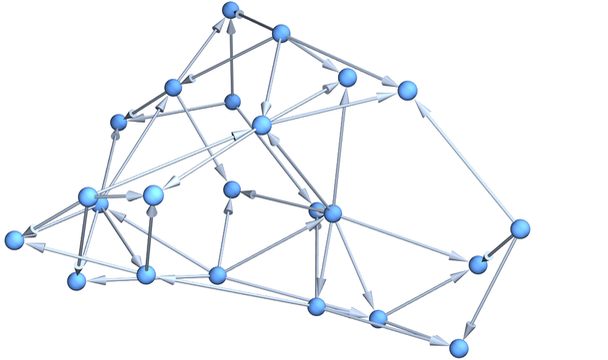✕ ```Graph3D[Rule @@@ ResourceFunction[ "WolframModel"][{{{x, y}, {x, z}} -> {{x, z}, {x, w}, {y, w}, {z, w}}}, {{0, 0}, {0, 0}}, 5, "FinalState"], GraphLayout -> "SpringElectricalEmbedding"]```

On a slightly larger scale we might have: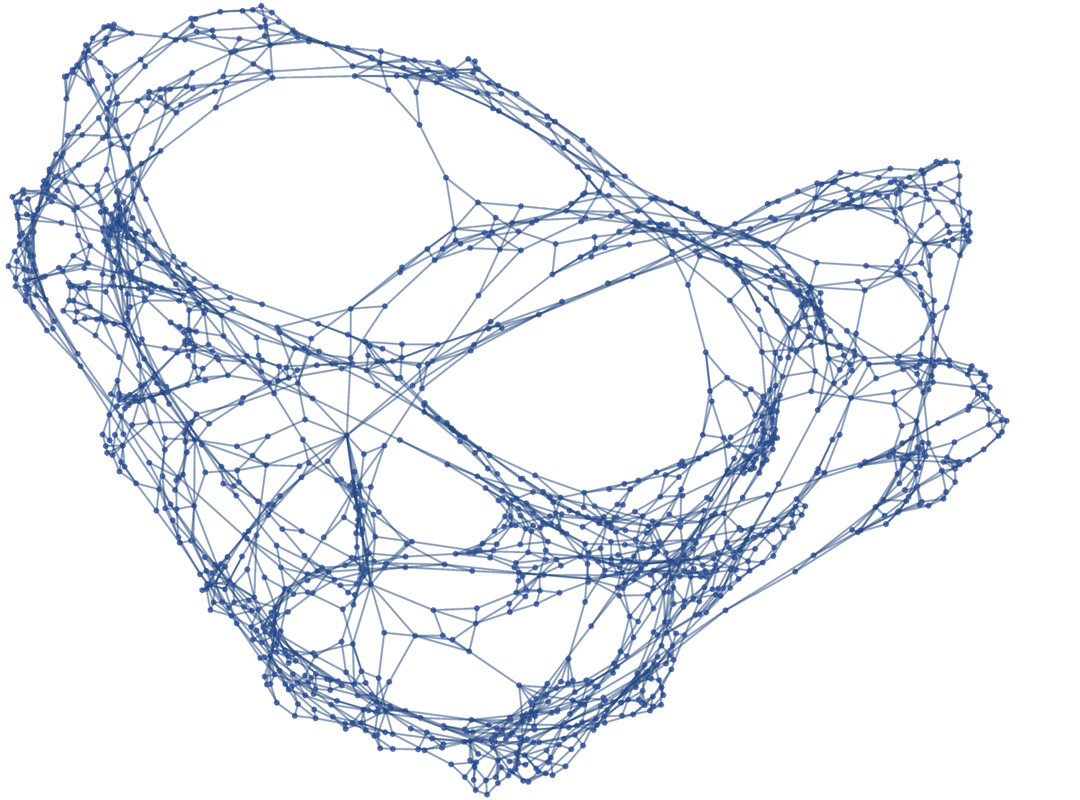✕ ```Graph3D[Rule @@@ ResourceFunction[ "WolframModel"][{{{x, y}, {x, z}} -> {{x, z}, {x, w}, {y, w}, {z, w}}}, {{0, 0}, {0, 0}}, 12, "FinalState"]]```

And in our actual universe we might have a hypergraph with perhaps 10400 nodes.

How does a giant hypergraph behave like continuous space? In a case like this we can see that the nodes can be thought of as forming a 2D grid on a (curved) surface: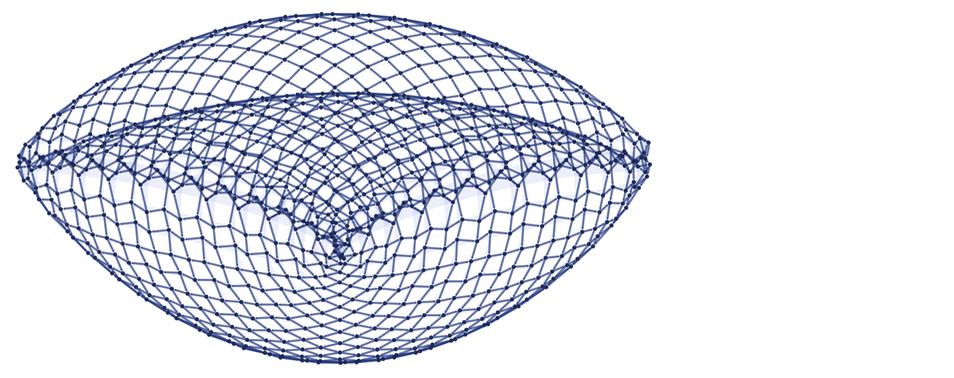✕ ```ResourceFunction[ "WolframModel"][{{1, 2, 3}, {4, 2, 5}} -> {{6, 3, 1}, {3, 6, 4}, {1, 2, 6}}, {{0, 0, 0}, {0, 0, 0}}, 1000, "FinalStatePlot"]```

There’s nothing intrinsic about our model of space that determines the effective dimensionality it will have. These are all perfectly good possible (hyper)graphs, but on a large scale they behave like space in different numbers of dimensions: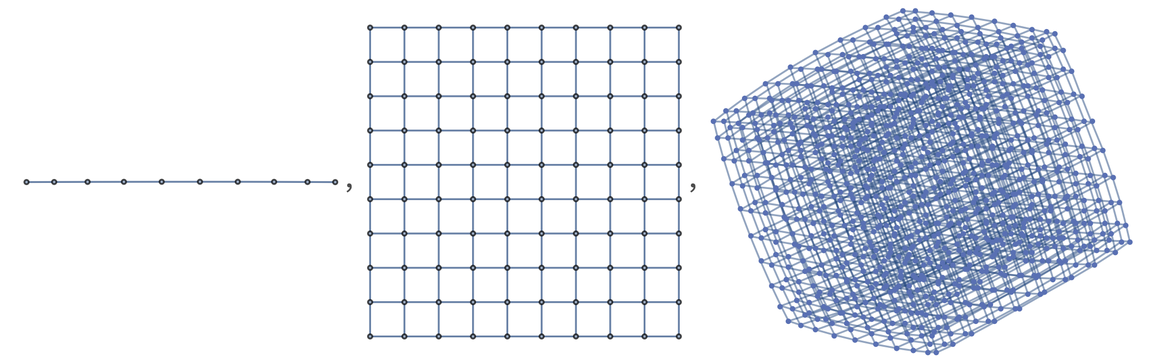✕ `Table[GridGraph[Table[10, n]], {n, 1, 3}]`

It’s convenient to introduce the notion of a “geodesic ball”: the region in a (hyper)graph that one reaches by following at most r connections in the (hyper)graph. A key fact is that in a (hyper)graph that limits to d-dimensional space, the number of nodes in the geodesic ball grows like rd. In a curved space (say, on the surface of a sphere) there’s a correction to rd, proportional to the curvature of the space.

The full story is quite long, but ultimately what happens is that—much as we can derive the properties of a fluid from the large-scale aggregate dynamics of lots of discrete molecules—so we can derive the properties of space from the large-scale aggregate dynamics of lots of nodes in our hypergraphs. And—excitingly enough—it seems that we get exactly Einstein’s equations from general relativity.

OK, so if space is a collection of elements laid out in a “spatial hypergraph”, what is time? Unlike in standard physics, it’s something initially very different. It’s a reflection of the process of computation by which the spatial hypergraph is progressively updated.

Let’s say our underlying rule for updating the hypergraph is: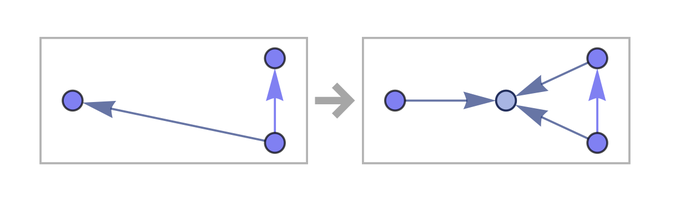✕ ```RulePlot[ResourceFunction[ "WolframModel"][{{x, y}, {x, z}} -> {{x, y}, {x, w}, {y, w}, {z, w}}]]```

Here’s a representation of the results of a sequence of updates according to this: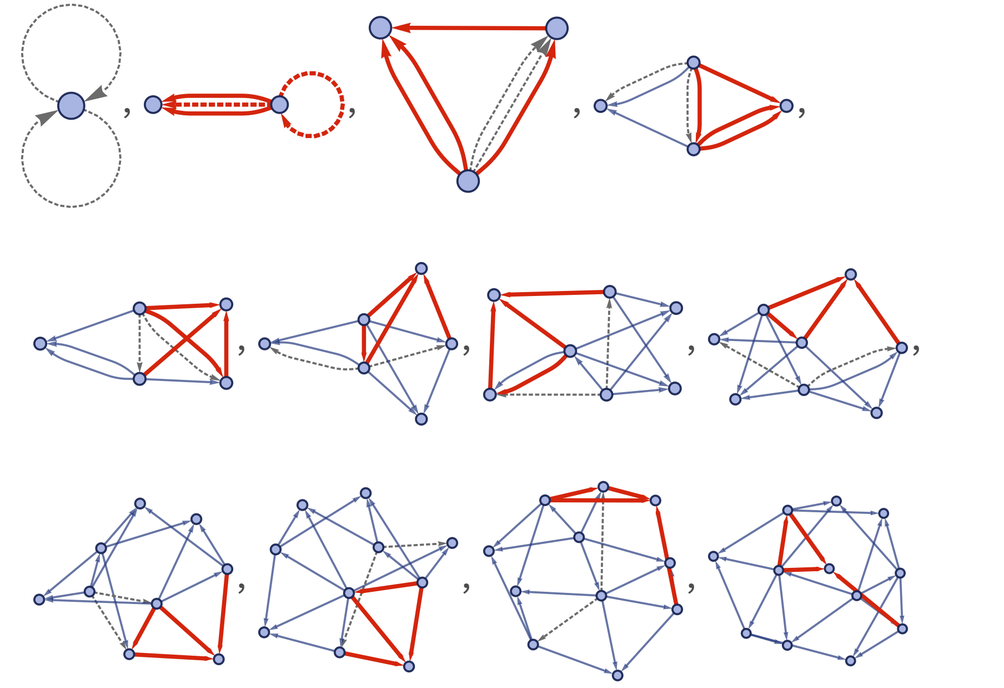✕ ```Flatten[With[{eo = ResourceFunction[ "WolframModel"][{{x, y}, {x, z}} -> {{x, z}, {x, w}, {y, w}, {z, w}}, {{0, 0}, {0, 0}}, 4]}, TakeList[eo["EventsStatesPlotsList", ImageSize -> Tiny], eo["GenerationEventsCountList", "IncludeBoundaryEvents" -> "Initial"]]]]```

Going further we’ll get for example: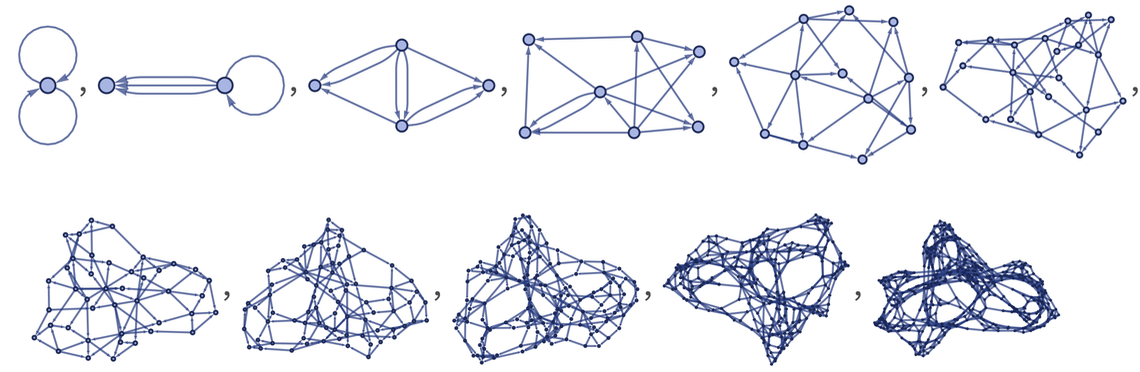✕ ```ResourceFunction[ "WolframModel"][{{x, y}, {x, z}} -> {{x, z}, {x, w}, {y, w}, {z, w}}, {{1, 1}, {1, 1}}, 10]["StatesPlotsList", "MaxImageSize" -> 100]```

But there’s a crucial point here. The underlying rule just defines how a local piece of hypergraph that has a particular form should be updated. If there are several pieces of hypergraph that have that form, it doesn’t say anything about which of them should be updated first. But once we’ve done a particular update, that can affect subsequent updates—and in general there’s a whole “causal graph” of causal relationships between updates.

We can see what’s going on a little more easily if instead of using spatial hypergraphs we just use strings of characters. Here we’re updating a string by repeatedly applying the (“sorting”) rule BA  AB: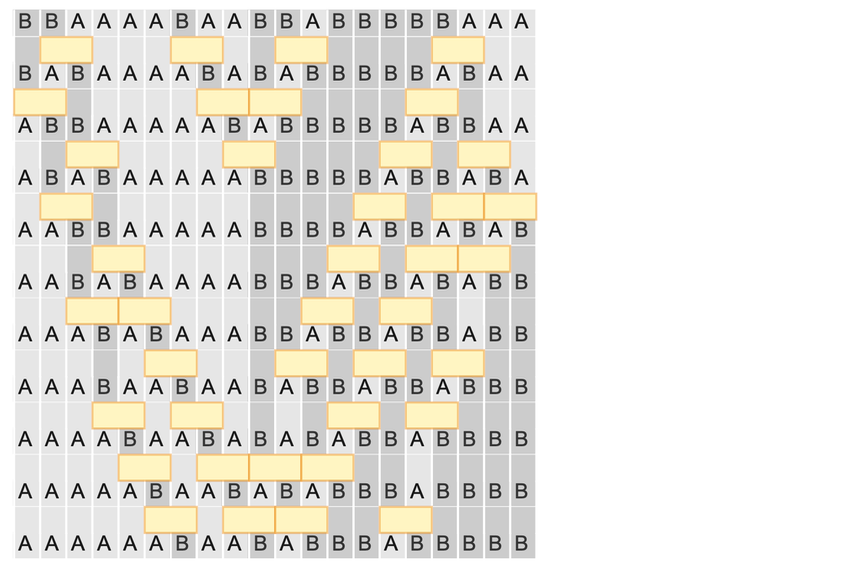✕ ```evo = (SeedRandom; ResourceFunction[ "SubstitutionSystemCausalEvolution"][{"BA" -> "AB"}, "BBAAAABAABBABBBBBAAA", 10, {"Random", 4}]); ResourceFunction["SubstitutionSystemCausalPlot"][evo, EventLabels -> False, CellLabels -> True, CausalGraph -> False]```

The yellow boxes indicate “updating events”, and we can join them by a causal graph that represents which event affects which other ones: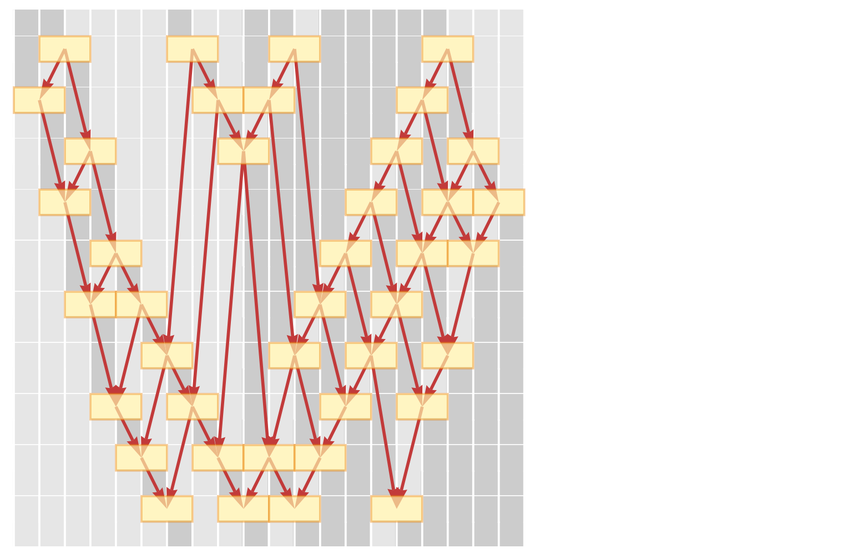✕ ```evo = (SeedRandom; ResourceFunction[ "SubstitutionSystemCausalEvolution"][{"BA" -> "AB"}, "BBAAAABAABBABBBBBAAA", 10, {"Random", 4}]); ResourceFunction["SubstitutionSystemCausalPlot"][evo, EventLabels -> False, CellLabels -> False, CausalGraph -> True]```

If we’re an observer inside this system, all we can directly tell is what events are occurring, and how they’re causally connected. But to set up a description of what’s going on, it’s convenient to be able to talk about certain events happening “at a certain time”, and others happening later. Or, in other words, we want to define some kind of “simultaneity surfaces”—or a “reference frame”.

Here are two choices for how to do this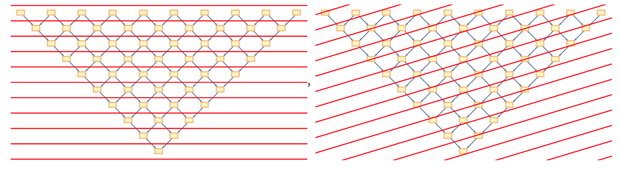✕ ```CloudGet["https://wolfr.am/KVkTxvC5"]; \ CloudGet["https://wolfr.am/KVl97Tf4"]; Show[regularCausalGraphPlot[10, {1, 0}, {#, 0.0}, lorentz], ImageSize -> 330] & /@ {0., .3}```

where the second one can be reinterpreted as: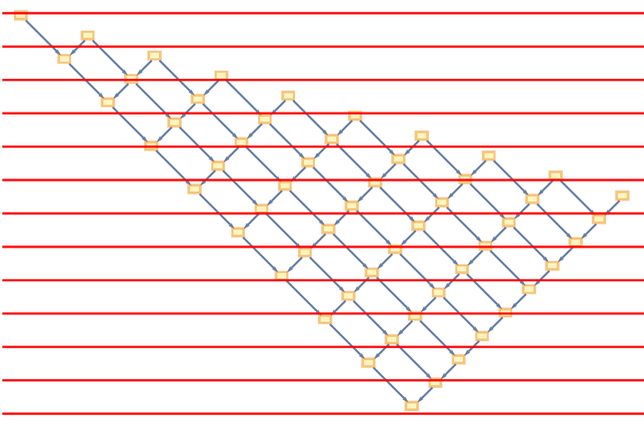✕ ```CloudGet["https://wolfr.am/KVkTxvC5"]; \ CloudGet["https://wolfr.am/KVl97Tf4"]; regularCausalGraphPlot[10, {1, 0}, {0.3, 0.0}, lorentz[0.3]]```

And, yes, this can be thought of as corresponding to a reference frame with a different speed, just like in standard special relativity. But now there’s a crucial point. The particular rule we’ve used here is an example of one with the property of causal invariance—which means that it doesn’t matter “at what time” we do a particular update; we’ll always get the same causal graph. And this is why—even though space and time start out so differently in our models—we end up being able to derive the fact that they follow special relativity.

Given a reference frame, we can always “reconstruct” a view of the behavior of the system from the causal graph. In the cases shown here we’d get: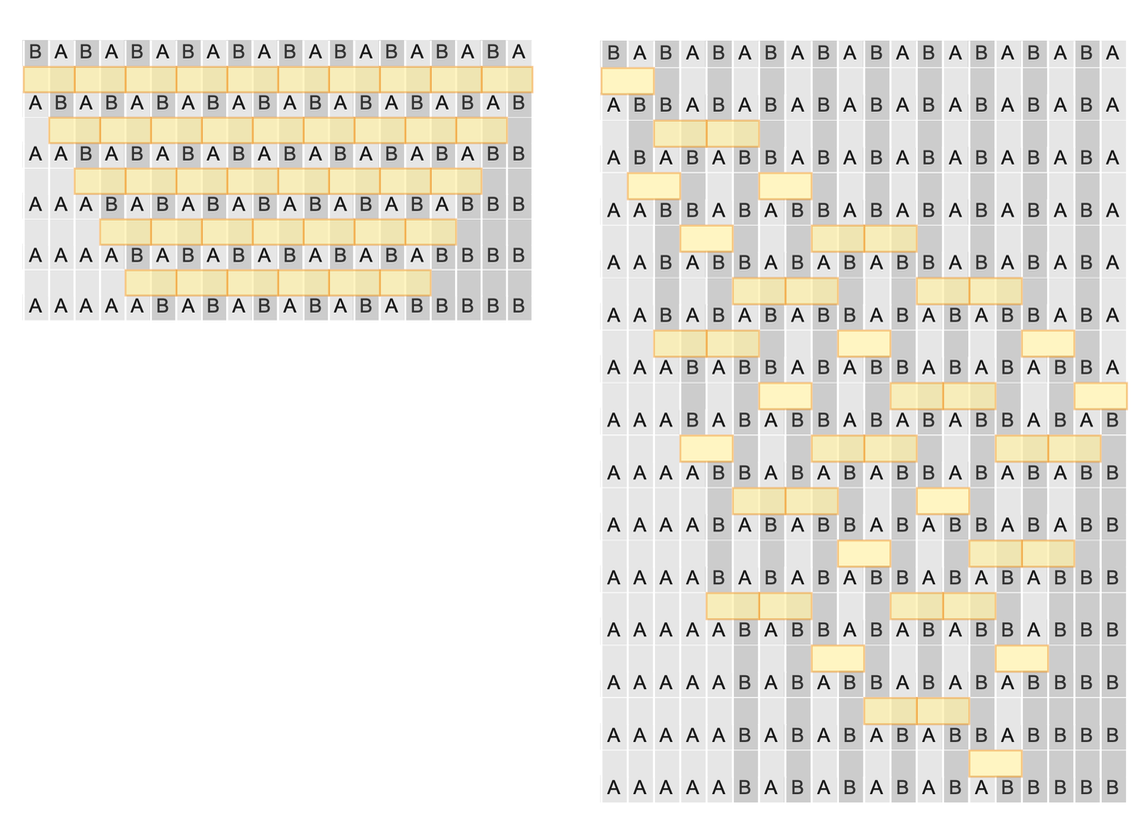✕ ```CloudGet["https://wolfr.am/LbaDFVSn"]; GraphicsRow[ Show[ResourceFunction["SubstitutionSystemCausalPlot"][ boostedEvolution[ ResourceFunction[ "SubstitutionSystemCausalEvolution"][{"BA" -> "AB"}, StringRepeat["BA", 10], 5], #], EventLabels -> False, CellLabels -> True, CausalGraph -> False], ImageSize -> {250, Automatic}] & /@ {0., 0.3}, Alignment -> Top]```

And the fact that the system seems to “take longer to do its thing” in the second reference frame is precisely a reflection of relativistic time dilation in that frame.

Just as with strings, we can also draw causal graphs to represent the causal relationships between updating events in spatial hypergraphs. Here’s an example of what we get for the rule shown above: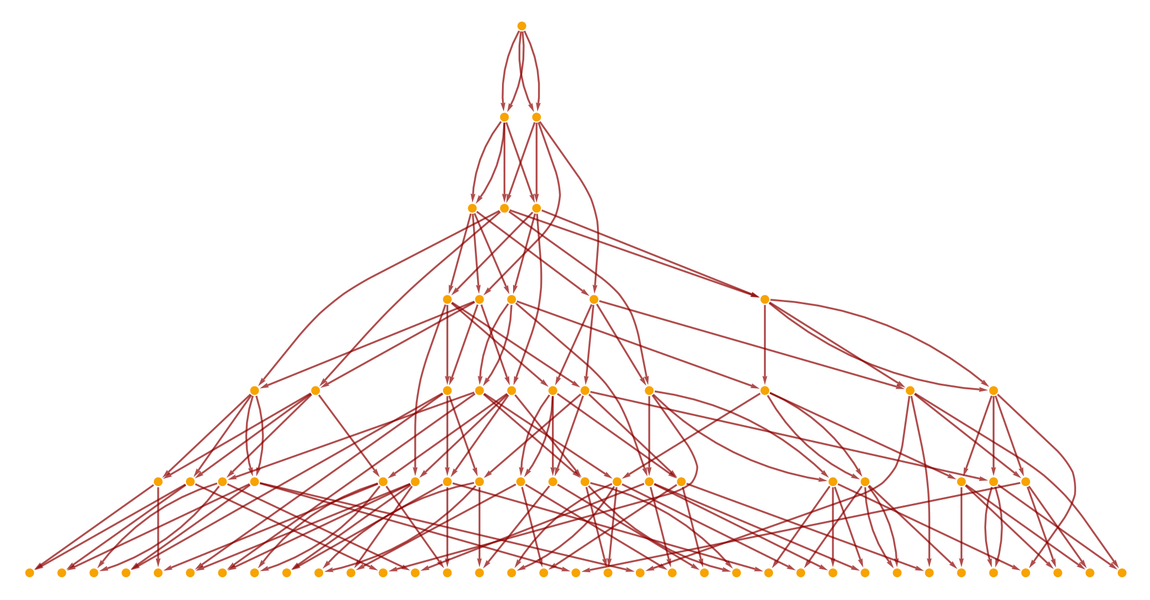✕ ```ResourceFunction[ "WolframModel"][{{{x, y}, {x, z}} -> {{x, z}, {x, w}, {y, w}, {z, w}}}, {{0, 0}, {0, 0}}, 7]["LayeredCausalGraph", AspectRatio -> 1/2]```

And once again we can set up reference frames to define what events we want to consider “simultaneous”. The only fundamental constraint on our reference frames is that in each slice of the “foliation” that defines the reference frame there can never be two events in which one follows from the other. Or, in the language of relativity, no events in a given slice can be timelike separated; instead, all of them must be spacelike separated, so that the slice defines a purely spacelike hypersurface.

In drawing a causal graph like the one above, we’re picking a particular collection of relative orderings of different possible updating events in the spatial hypergraph. But why one choice and not another? A key feature of our models is that actually we can think of all possible orderings as being done, or said, differently, we can construct a whole multiway graph of possibilities. Here’s what the multiway graph looks like for the string system above: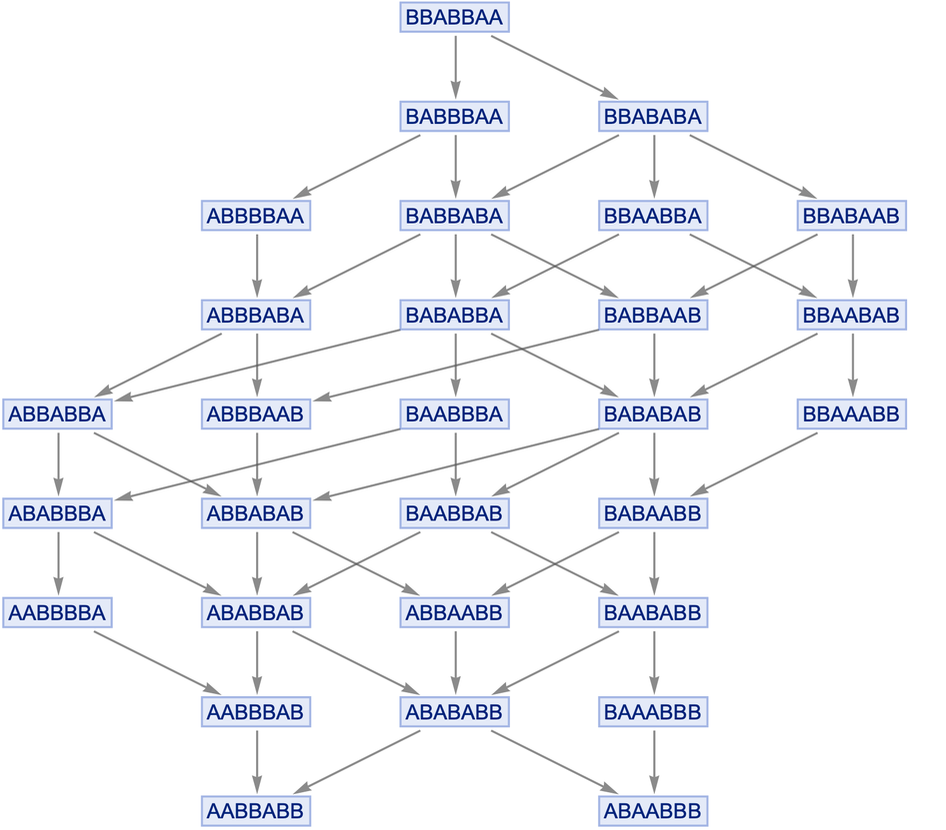✕ ```LayeredGraphPlot[ ResourceFunction["MultiwaySystem"][{"BA" -> "AB"}, "BBABBAA", 8, "StatesGraph"], AspectRatio -> 1]```

Each node in this multiway graph represents a complete state of our system (in this case, a string), and a path through the multiway system corresponds to a possible history of the system, with a particular corresponding causal graph.

But now there’s an important connection with physics: the fact that we get a multiway graph makes quantum mechanics inevitable in our models. And it turns out that just like we can use reference frames to make sense of the evolution of our systems in space and time, so also we can use “quantum observation frames” to make sense of the time evolution of multiway graphs. But now the analog of space is what we call “branchial space”: in effect a space of possible quantum states, with the connections between states defined by their relationship on branches in the multiway system.

And much as we can define a spatial hypergraph representing relationships between “points in space”, so we can define a branchial graph that represents relationships (or “entanglements”) between quantum states, in branchial space: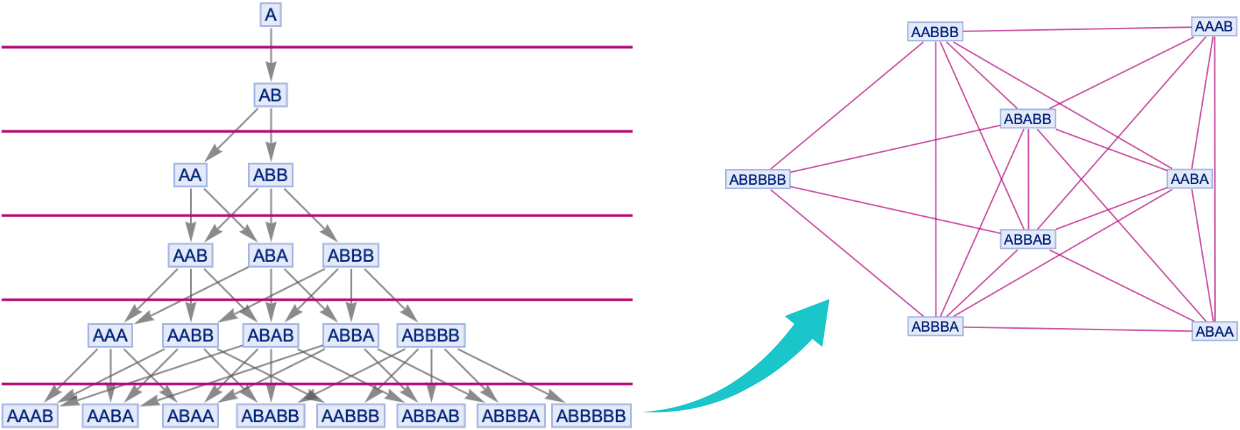✕ ```Cell[CellGroupData[{Cell[BoxData[ RowBox[{"LayeredGraphPlot", "[", RowBox[{ RowBox[{"Graph", "[", RowBox[{ RowBox[{"ResourceFunction", "[", "\"\\"", "]"}], "[", RowBox[{ RowBox[{"{", RowBox[{ RowBox[{"\"\\"", "\[Rule]", "\"\\""}], ",", RowBox[{"\"\\"", "\[Rule]", "\"\\""}]}], "}"}], ",", "\"\\"", ",", "5", ",", "\"\\""}], "]"}], "]"}], ",", RowBox[{"Epilog", "\[Rule]", RowBox[{"{", RowBox[{ RowBox[{ RowBox[{ "ResourceFunction", "[", "\"\\"", "]"}], "[", RowBox[{"\"\\"", ",", "\"\\""}], "]"}], ",", RowBox[{"AbsoluteThickness", "[", "1.5", "]"}], ",", RowBox[{"Table", "[", RowBox[{ RowBox[{"Line", "[", RowBox[{"{", RowBox[{ RowBox[{"{", RowBox[{ RowBox[{"-", "10"}], ",", "i"}], "}"}], ",", RowBox[{"{", RowBox[{"9", ",", "i"}], "}"}]}], "}"}], "]"}], ",", RowBox[{"{", RowBox[{"i", ",", ".4", ",", "5", ",", "1.05"}], "}"}]}], "]"}]}], "}"}]}]}], "]"}]], "Input"], Cell[BoxData[ RowBox[{ RowBox[{"ResourceFunction", "[", "\"\\"", "]"}], "[", RowBox[{ RowBox[{"{", RowBox[{ RowBox[{"\"\\"", "\[Rule]", "\"\\""}], ",", RowBox[{"\"\\"", "\[Rule]", "\"\\""}]}], "}"}], ",", "\"\\"", ",", "5", ",", "\"\\""}], "]"}]], "Input"] }, Open ]]```

I won’t go into the details here, but one of the beautiful things in our models is that just as we can derive the Einstein equations as a large-scale limiting description of the behavior of our spatial hypergraphs, so also we can figure out the large-scale limiting behavior for multiway systems—and it seems that we get the Feynman path integral for quantum mechanics!

By the way, since we’re talking about faster than light and motion in space, it’s worth mentioning that there’s also a notion of motion in branchial space. And just like we have the speed of light c that defines some kind of limit on how fast we can explore physical space, so also we have a maximal entanglement rate ζ that defines a limit on how fast we can explore (and thus “entangle”) different quantum states in branchial space. And just as we can ask about “faster than c”, we can also talk about “faster than ζ”. But before we get to that, we’ve got a lot of other things to discuss.

## Can We Make Tunnels in Space?

Traditional general relativity describes space as a continuous manifold that evolves according to certain partial differential equations. But our models talk about what’s underneath that, and what space actually seems to be made of. And while in appropriate limits they reproduce what general relativity says, they also imply all sorts of new and different phenomena.

Imagine that the hypergraph that represents space has the form of a simple 2D grid: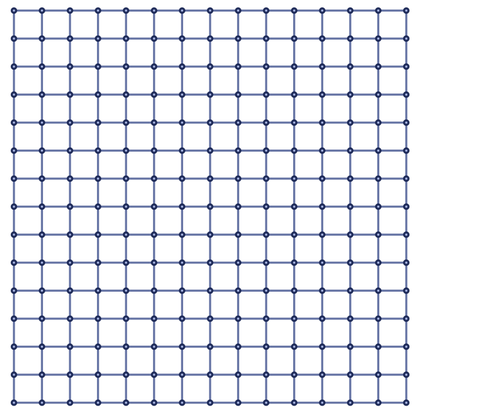✕ ```GridGraph[{15, 15}, EdgeStyle -> ResourceFunction["WolframPhysicsProjectStyleData"]["SpatialGraph", "EdgeLineStyle"], VertexStyle -> ResourceFunction["WolframPhysicsProjectStyleData"]["SpatialGraph", "VertexStyle"]]```

In the limit this will be like 2D Euclidean space. But now suppose we add some extra “long-range threads” to the graph: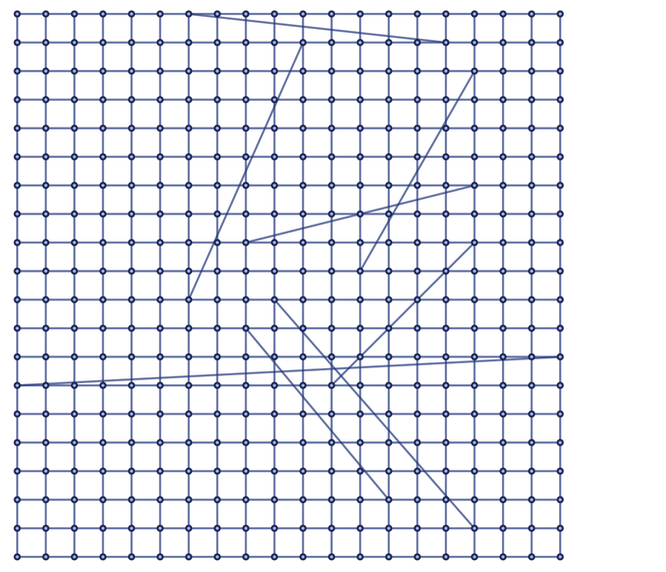✕ ```SeedRandom; With[{g = GridGraph[{20, 20}]}, EdgeAdd[g, UndirectedEdge @@@ Select[Table[RandomInteger[{1, VertexCount[g]}, 2], 10], GraphDistance[g, #[], #[]] > 8 &], EdgeStyle -> ResourceFunction["WolframPhysicsProjectStyleData"]["SpatialGraph", "EdgeLineStyle"], VertexStyle -> ResourceFunction["WolframPhysicsProjectStyleData"]["SpatialGraph", "VertexStyle"]]]```

Here’s a different rendering of the same graph: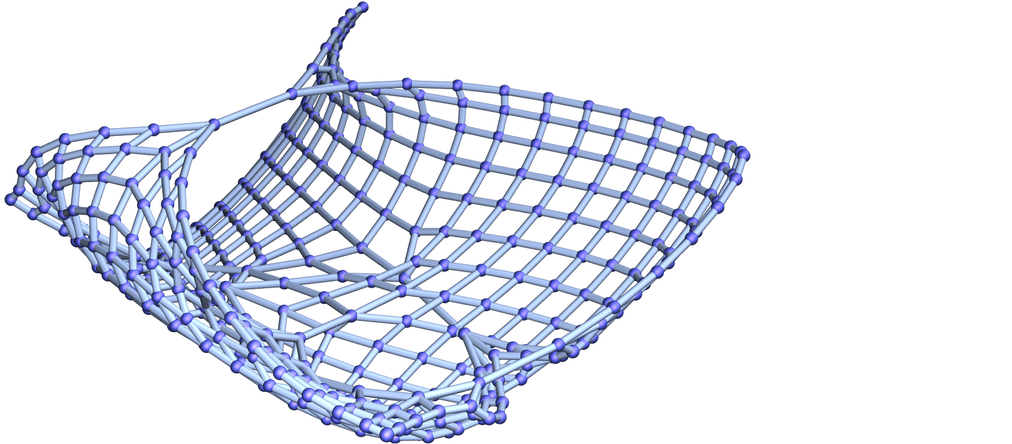✕ ```Graph3D[EdgeList[%], EdgeStyle -> ResourceFunction["WolframPhysicsProjectStyleData"]["SpatialGraph3D", "EdgeLineStyle"], VertexStyle -> ResourceFunction["WolframPhysicsProjectStyleData"]["SpatialGraph3D", "VertexStyle"]]```

Now let’s ask about distances on this graph. Some nodes on the graph will have distances that are just like what one would expect in ordinary 2D space. But some will be “anomalously close”, because one will be able to get from one to another not by going “all the way through 2D space” but by taking a shortcut along one of the long-range threads.

Let’s say that we’re able to move around so that at every elementary interval of time we traverse a single connection in the graph. Then if our view of “what space is like” is based on the general structure of the graph (ignoring the long-range threads) we’ll come to some conclusion about how far we can go in a certain time—and what the maximum speed is at which we can “go through space”. But then what happens if we encounter one of the long-range threads? If we go through it we’ll be able to get from one “place in space” to another much faster than would be implied by the maximum speed we deduced from looking at “ordinary space”.

In a graph, there are many ways to end up having “long-range threads”—and we can think of these as defining various kinds of “space tunnels” that provide ways to get around in space evading usual speed-of-light constraints. We can imagine both persistent space tunnels that could be repeatedly used, and spontaneous or “just-in-time” ones that exist only transiently. But—needless to say—there is all sorts of subtlety around the notion of space tunnels. If a tunnel is a pattern in a graph, what actually happens when something “goes through it”? And if a tunnel didn’t always exist, how does it get formed?

Space tunnels are a fairly general concept that can be defined on graphs or hypergraphs. But there’s at least a special case of them that can be defined even in standard general relativity: wormholes. General relativity describes space as a continuum—a manifold—in which there’s no way to have “just a few long-range threads”. The best one can do is to imagine that there’s a kind of “handle in space”, that provides an alternative path from one part of space to another: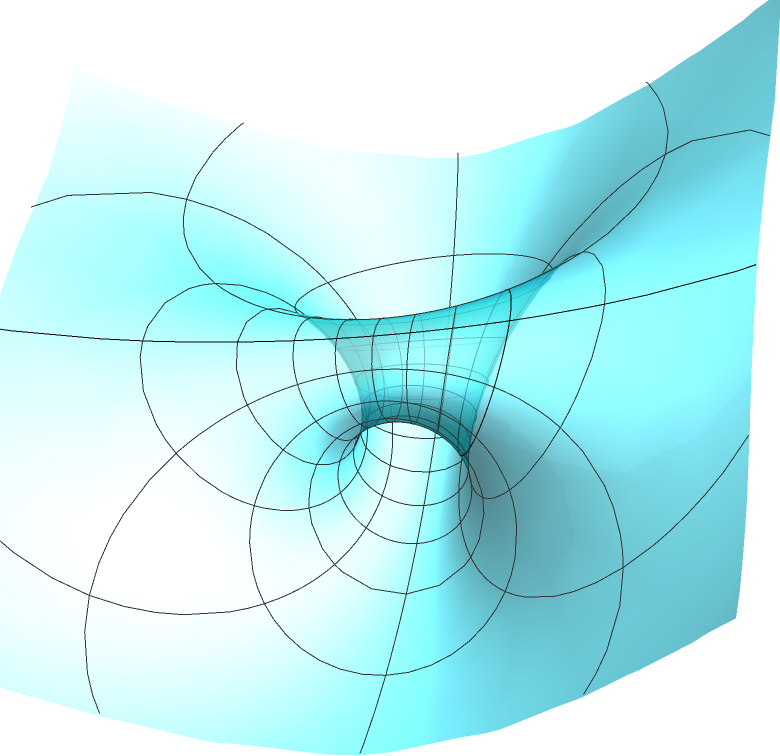How would such a non-simply-connected manifold form? Perhaps it’s a bit like the gastrulation that happens in embryonic development. But mathematically one can’t continuously change the topology of something continuous; there has to at least be some kind of singularity. In general relativity it’s been tricky to see how this could work. But of course in our models there’s not the same kind of constraint, because one doesn’t have to “rearrange a whole continuum”; one can do something more like “growing a handle one thread at a time”.

Here’s an example where one can see something a bit like this happening. We’re using the rule: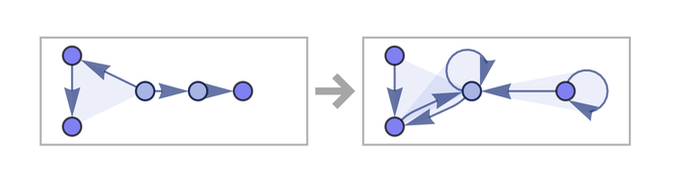✕ ```RulePlot[ResourceFunction[ "WolframModel"][{{1, 2, 3}, {1, 4, 5}} -> {{3, 3, 6}, {6, 6, 5}, {4, 5, 6}}]]```

And what it does is effectively to “knit handles” that provide “shortcuts” between “separated” points in patches of what limits to 2D Euclidean space: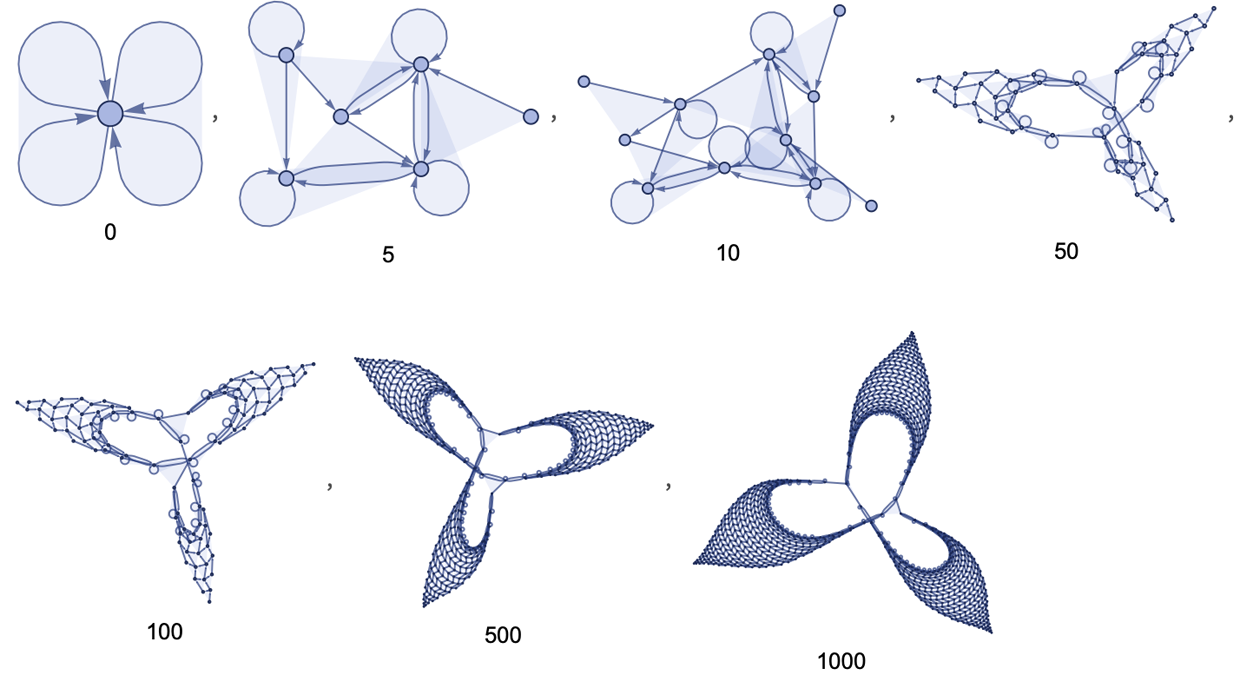✕ ```Labeled[ResourceFunction[ "WolframModel"][{{1, 2, 3}, {1, 4, 5}} -> {{3, 3, 6}, {6, 6, 5}, {4, 5, 6}}, {{0, 0, 0}, {0, 0, 0}}, #, "FinalStatePlot"], Text[#]] & /@ {0, 5, 10, 50, 100, 500, 1000}```

In our models—free from the constraints of continuity—space can have all sorts of exotic forms. First of all, there’s no constraint that space has to have an integer number of dimension (say 3). Dimension is just defined by the asymptotic growth rates of balls, and can have any value. Like here’s a case that approximates 2.3-dimensional space: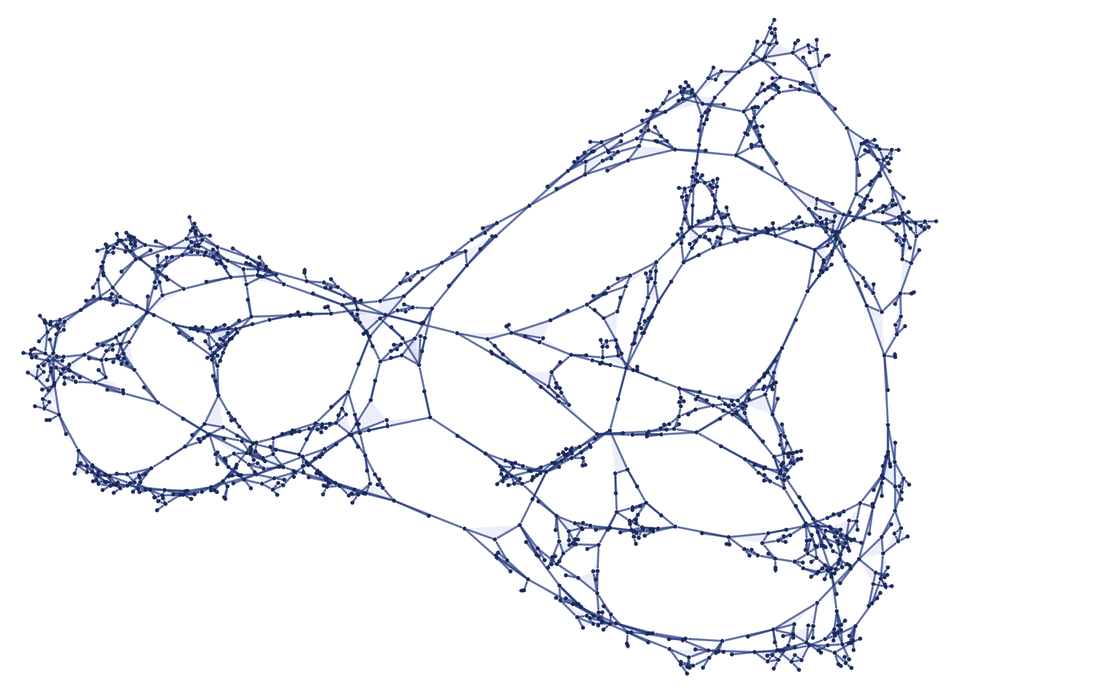✕ ```ResourceFunction[ "WolframModel"][{{{1, 2, 3}, {2, 4, 5}} -> {{6, 7, 2}, {5, 7, 8}, {4, 2, 8}, {9, 3, 5}}}, {{0, 0, 0}, {0, 0, 0}}, 20, "FinalStatePlot"]```

It’s worth noting that although it’s perfectly possibly to define distance—and, in the limit, lots of other geometric concepts—on a graph like this, one doesn’t get to say that nodes are at positions defined by particular sets of coordinates, as one would in integer-dimensional space.

With a manifold, one basically has to pick a certain (integer) dimension, then stick to it. In our models, dimension can effectively become a dynamical variable, that can change with position (and time). So in our models one possible form of “space tunnel” is a region of space with higher or lower dimension. (Our derivation of general relativity is based on assuming that space has a limiting finite dimension, then asking what curvature and other properties it must have; the derivation is in a sense blind to different-dimensional space tunnels.)

It’s worth noting that both lower- and higher-dimensional space tunnels can be interesting in terms of “getting places quickly”. Lower-dimensional space tunnels (such as bigger versions of the 1D long-range threads in the 2D grid above) potentially connect some specific sparse set of “distant” points. Higher-dimensional space tunnels (which in the infinite-dimensional limit can be trees) are more like “switching stations” that make many points on their boundaries closer.

## Negative Mass, Wormholes, etc.

Let’s say we’ve somehow managed to get a space tunnel. What will happen to it? Traditional general relativity suggests that it’s pretty hard to maintain a wormhole under the evolution of space implied by Einstein’s equations. A wormhole is in effect defined by geodesic paths coming together when they enter the wormhole and diverging again when they exit. In general relativity the presence of mass makes geodesics converge; that’s the “attraction due to gravity”. But what could make the geodesics diverge again? Basically one needs some kind of gravitational repulsion. And the only obvious way to get this in general relativity is to introduce negative mass.

Normally mass is assumed to be a positive quantity. But, for example, dark energy effectively has to have negative mass. And actually there are several mechanisms in traditional physics that effectively lead to negative mass. All of them revolve around the question of where one sets the zero to be. Normally one sets things up so that one can say that “the vacuum” has zero energy (and mass). But actually—even in traditional physics—there’s lots that’s supposed to be going on in “the vacuum”. For example, there’s supposed to be a constant intensity of the Higgs field, that interacts with all massive particles and has the effect of giving them mass. And there are supposed to be vacuum fluctuations associated with all quantum fields, each leading (at least in standard quantum field theory) to an infinite energy density.

But if these things exist everywhere in the universe, then (at least for most purposes) we can just set our zero of energy to include them. So then if there’s anything that can reduce their effects, we’ll effectively see negative mass. And one example of where this can in some sense happen is the Casimir effect. Imagine that instead of having an infinite vacuum, we just have vacuum inside a box. Having the box cuts out some of the possible vacuum fluctuations of quantum fields (basically modes with wavelengths larger than the size of the box)—and so in some sense leads to negative energy density inside the box (at least relative to outside). And, yes, the effect is observable with metal boxes, etc. But what becomes of the Casimir effects in a purely spacetime or gravitational setting isn’t clear.

(This leads to a personal anecdote. Back in 1981 I wrote two papers about the Casimir effect with Jan Ambjørn, titled Properties of the Vacuum: 1. Mechanical and …: 2. Electrodynamic. We had planned a “…: 3. Gravitational” but never wrote it, and now I’m really curious what the results would have been. By the way, our paper #1 computed Casimir effects for boxes of different shapes, and had the surprising implication that by changing shapes in a cycle it would in principle be possible to continuously “mine” energy from the vacuum. This was later suggested as a method for interstellar propulsion, but to make it work requires an infinitely impermeable box, which doesn’t seem physically constructible, except maybe using gravitational effects and event horizons… but we never wrote paper #3 to figure that out….)

In traditional physics there’s been a conflict between what the vacuum is like according to quantum field theory (with infinite energy density from vacuum fluctuations, etc.) and what the vacuum is assumed to be like in general relativity (effectively zero energy density). In our models there isn’t the same kind of conflict, but “the vacuum” is something with even more structure.

In particular, in our models, space isn’t some separate thing that exists; it is just a consequence of the large-scale structure of the spatial hypergraph. And any matter, particles, quantum fields, etc. that exist “in space” must also be features of this same hypergraph. Things like vacuum fluctuations aren’t something that happens in space; they are an integral part of the formation of space itself.

By the way, it’s important to note that in our models the hypergraph isn’t something static—and it’s in the end knitted together only through actual update events that occur. And the energy of some region of the hypergraph is directly related to the amount of updating activity in that region (or, more accurately, to the flux of causal edges through that portion of spacelike hypersurfaces).

So what does this mean for negative mass in our models? Well, if there was a region of the hypergraph where there was somehow less activity, it would have negative energy relative to the zero defined by the “normal vacuum”. It’s tempting to call whatever might reduce activity in the hypergraph a “vacuum cleaner”. And, no, we don’t know if vacuum cleaners can exist. But if they do, then there’s a fairly direct path to seeing how wormholes can be maintained (basically because geodesics almost by definition diverge wherever a vacuum cleaner has operated).

By the way, while a large-scale wormhole-like structure presumably requires negative mass, vacuum cleaners, etc., and other space tunnel structures may not have the same requirements. By their very construction, they tend to operate outside the regime described by general relativity and Einstein’s equations. So things like the standard singularity theorems of general relativity can’t be expected to apply. And instead there doesn’t seem to be any choice but to analyze them directly in the context of our models.

One might think: given a particular space tunnel configuration, why not just run a simulation of it, and see what happens? The problem is computational irreducibility. Yes, the simulation might show that the configuration is stable for a million or a billion steps. But that might still be far, far away from human-level timescales. And there may be no way to determine what the outcome for a given number of steps will be except in effect by doing that irreducible amount of computational work—so that if, for example, we want to find out the limiting result after an infinite time, that’ll in general require an infinite amount of computational work, and thus effectively be undecidable.

Or, put another way, even if we can successfully “engineer” a space tunnel, there may be no systematic way to guarantee that it’ll “stay up”; it may require an infinite sequence of “engineering tweaks” to keep it going, and eventually it may not be possible to keep it going. But before that, of course, we have to figure out how to construct a space tunnel in the first place…

## It Doesn’t Mean Time Travel

In ordinary general relativity one tends to think of everything in terms of spacetime. So if a wormhole connects two different places, one assumes they are places in spacetime. Or, in other words, a wormhole can allow shortcuts between both different parts of space, and different parts of time. But with a shortcut between different parts of time one can potentially have time travel.

More specifically, one can have a situation where the future of something affects its past: in other words there is a causal connection from the future to the past. At some level this isn’t particularly strange. In any system that behaves in a perfectly periodic way one can think of the future as leading to a repetition of the past. But of course it’s not a future that one can freely determine; it’s just a future that’s completely determined by the periodic behavior.

How all this works is rather complicated to see in the standard mathematical treatment of general relativity, although in the end what presumably happens is that in the presence of wormholes the only consistent solutions to the equations are ones for which past and future are locked together with something like purely periodic behavior.

Still, in traditional physics there’s a certain sense that “time is just a coordinate”, so there’s the potential for “motion in time” just like we have motion in space. In our models, however, things work quite differently. Because now space and time are not the same kind of thing at all. Space is defined by the structure of the spatial hypergraph. But time is defined by the computational process of applying updates. And that computational process undoubtedly shows computational irreducibility.

So while we may go backwards and forwards in space, exploring different parts of the spatial hypergraph, the progress of time is associated with the progressive performance of irreducible computation by the universe. One can compute what will happen (or, with certain restrictions, what has happened), but one can only do so effectively by following the actual steps of it happening; one can’t somehow separately “move through it” to see what happens or has happened.

But in our models the whole causality of events is completely tracked, and is represented by the causal graph. And in fact each connection in the causal graph can be thought of as a representation of the very smallest unit of progression in time.

So now let’s look at a causal graph again: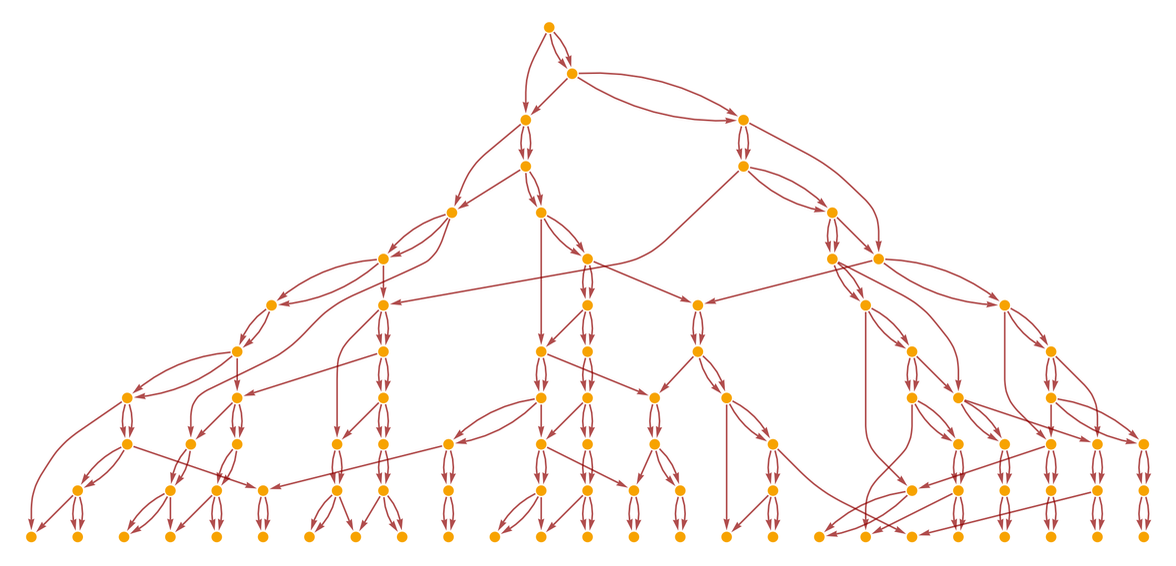✕ ```ResourceFunction[ "WolframModel"][{{x, y}, {z, y}} -> {{x, z}, {y, z}, {w, z}}, {{0, 0}, {0, 0}}, 12, "LayeredCausalGraph"]```

There’s a very important feature of this graph: it contains no cycles. In other words, there’s a definite “flow of causality”. There’s a partial ordering of what events can affect what other events, and there’s never any looping back, and having an event affect itself.

There are different ways we can define “simultaneity surfaces”, corresponding to different foliations of this graph: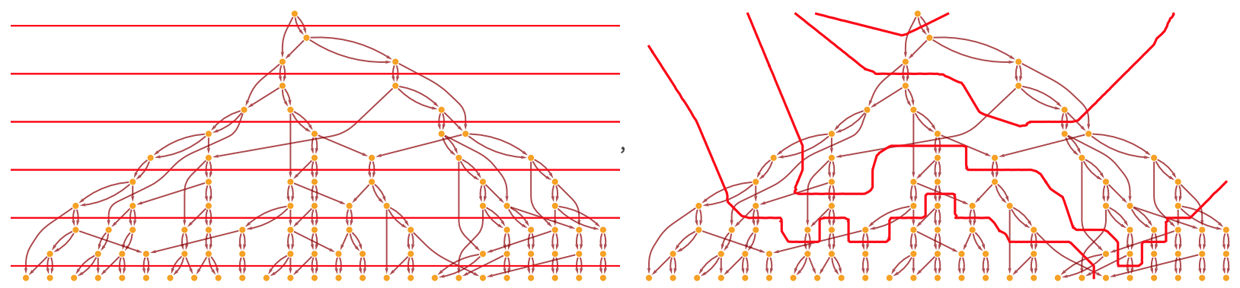✕ ```Show[#, ImageSize -> 400] & /@ {CloudGet["https://wolfr.am/KXgcRNRJ"]; evolution = ResourceFunction[ "WolframModel"][{{x, y}, {z, y}} -> {{x, z}, {y, z}, {w, z}}, {{0, 0}, {0, 0}}, 12]; gg = Graph[evolution["LayeredCausalGraph"]]; GraphPlot[gg, Epilog -> {Directive[Red], straightFoliationLines[{1/2, 0}, {0, 0}, (# &), {0, 1}]}], CloudGet["https://wolfr.am/KXgcRNRJ"];(*drawFoliation*) gg = Graph[ ResourceFunction[ "WolframModel"][{{x, y}, {z, y}} -> {{x, z}, {y, z}, {w, z}}, {{0, 0}, {0, 0}}, 12, "LayeredCausalGraph"]]; semiRandomWMFoliation = {{1}, {1, 2, 4, 6, 9, 3}, {1, 2, 4, 6, 9, 3, 13, 19, 12, 26, 36, 5, 7, 10, 51, 14, 69, 18, 8, 25, 11, 34, 20, 35, 50, 17}, {1, 2, 4, 6, 9, 3, 13, 19, 12, 26, 36, 5, 7, 10, 51, 14, 69, 18, 8, 25, 11, 34, 20, 35, 50, 17, 24, 68, 47, 15, 92, 27, 48, 37, 21, 28, 42, 22, 30, 16, 32, 23, 33, 46, 64, 90, 94, 65, 88, 49, 67, 91, 66, 89}}; Quiet[drawFoliation[gg, semiRandomWMFoliation, Directive[Red]], FindRoot::cvmit]}```

But there’s always a way to do it so that all events in a given slice are “causally before” events in subsequent slices. And indeed whenever the underlying rule has the property of causal invariance, it’s inevitable that things have to work this way.

But if we break causal invariance, other things can happen. Here’s an example of the multiway system for a (string) rule that doesn’t have causal invariance, and in which the same state can repeatedly be visited: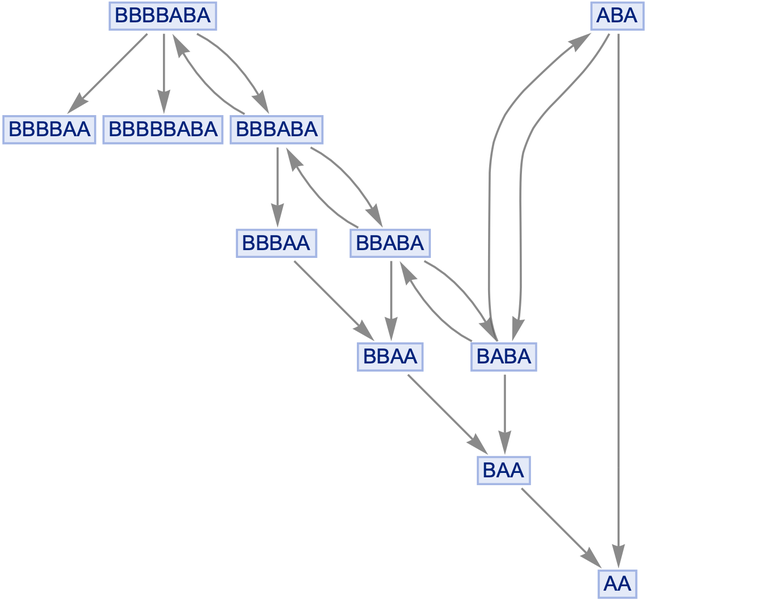✕ ```Graph[ResourceFunction["MultiwaySystem"][{"AB" -> "BAB", "BA" -> "A"}, "ABA", 5, "StatesGraph"], GraphLayout -> {"LayeredDigraphEmbedding", "RootVertex" -> "ABA"}]```

If we look at the corresponding (multiway) causal graph, it contains a loop: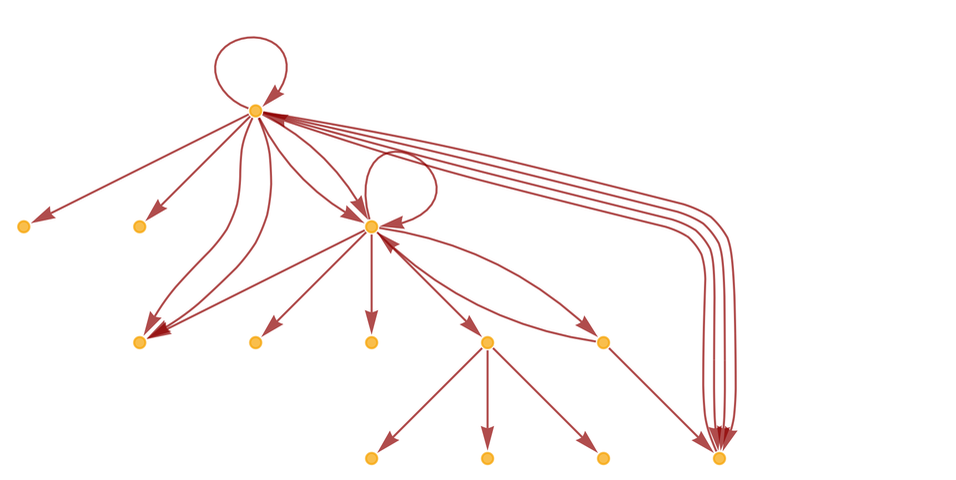✕ ```LayeredGraphPlot[ ResourceFunction["MultiwaySystem"][{"AB" -> "BAB", "BA" -> "A"}, "ABA", 4, "CausalGraphStructure"]]```

In the language of general relativity, this loop represents a closed timelike curve, where the future can affect the past. And if we try to construct a foliation in which “time systematically moves forward” we won’t be able to do it.

But the presence of these kinds of loops is a different phenomenon from the existence of space tunnels. In a space tunnel there’s connectivity in the spatial hypergraph that makes the (graph) distance between two points be shorter than you’d expect from the overall structure of the hypergraph. But it’s just connecting different places in space. An event that happens at one end of the space tunnel can affect events associated with distant places in space, but (assuming causal invariance, etc.) those events have to be “subsequent events” with respect to the partial ordering defined by the causal graph.

Needless to say, there’s all sorts of subtlety about the events involved in maintaining the space tunnel, the definition of distance being “shorter than you’d expect”, etc. But the main point here is that “jumping” between distant places in space doesn’t in any way require or imply “traveling backwards in time”. Yes, if you think about flat, continuum space and you imagine a tachyon going faster than light, then the standard equations of special relativity imply that it must be going backwards in time. But as soon as space itself can have features like space tunnels, nothing like this needs to be going on. Time—and the computational process that corresponds to it—can still progress even as effects propagate, say through space tunnels, faster than light to places that seem distant in space.

## Causal Cones and Light Cones

OK, now we’re ready to get to the meat of the question of faster-than-light effects in our models. Let’s say some event occurs. This event can affect a cone of subsequent events in the causal graph. When the causal graph is a simple grid, it’s all quite straightforward: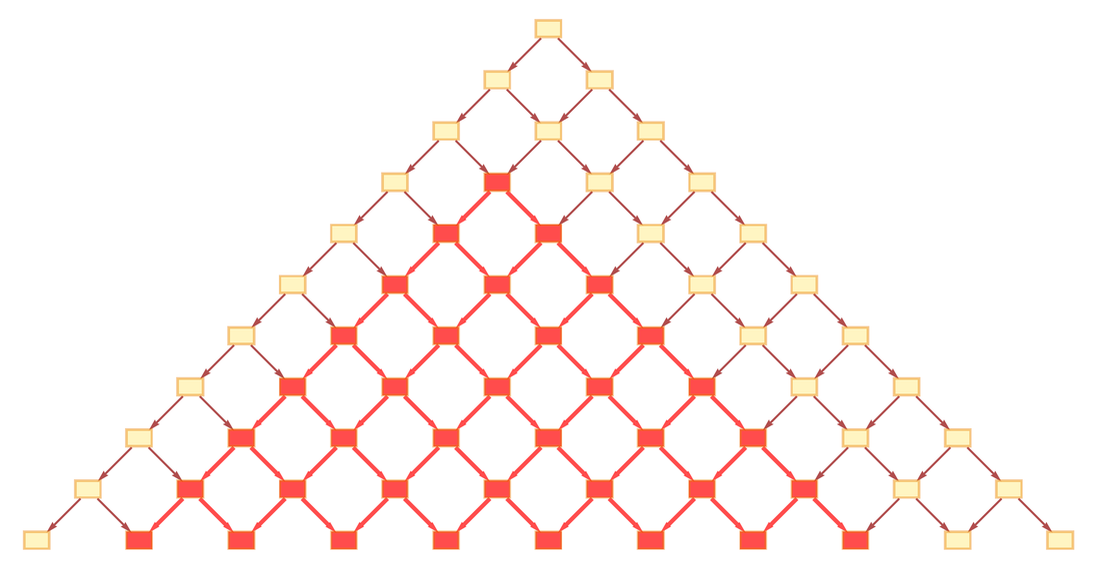✕ ```CloudGet["https://wolfr.am/LcADnk1u"]; upTriangleGraph = diamondCausalGraphPlot[11, {0, 0}, {}, # &, "Up", ImageSize -> 450]; HighlightGraph[upTriangleGraph, Style[Subgraph[upTriangleGraph, VertexOutComponent[upTriangleGraph, 8]], Red, Thick]]```

But in a more realistic causal graph the story is more complicated: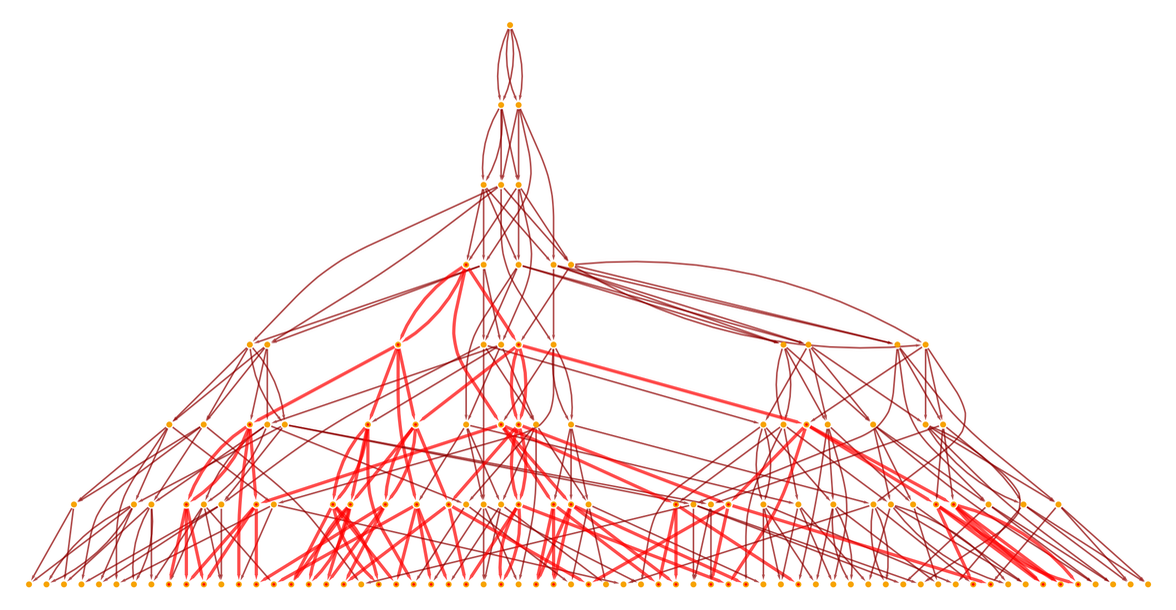✕ ```With[{g = ResourceFunction[ "WolframModel"][{{{x, y}, {x, z}} -> {{x, z}, {x, w}, {y, w}, {z, w}}}, {{0, 0}, {0, 0}}, 8]["LayeredCausalGraph", AspectRatio -> 1/2]}, HighlightGraph[g, Style[Subgraph[g, VertexOutComponent[g, 10]], Red, Thick]]]```

The “causal cone” of affected events is very well defined. But now the question is: how does this relate to what happens in space and time?

When one thinks about the propagation of effects in space and time one typically thinks of light cones. Given a source of light somewhere in space and time, where in space and time can this affect?

And one might assume that the causal cone is exactly the light cone. But things are more subtle than that. The light cone is normally defined by the positions in space and time that it reaches. And that makes perfect sense if we’re dealing with a manifold representing continuous spacetime, on which we can, for example, set up numerical coordinates. But in our models there’s not intrinsically anything like that. Yes, we can say what element in a hypergraph is affected after some sequence of events. But there’s no a priori way to say where that element is in space. That’s only defined in some limit, relative to everything else in the whole hypergraph.

And this is the nub of the issue of faster-than-light effects in our models: causal (and, in a sense, temporal) relationships are immediately well defined. But spatial ones are not. One event can affect another through a single connection in the causal graph, but those events might be occurring at different ends of a space tunnel that traverses what we consider to be a large distance in space.

There are several related issues to consider, but they center around the question of what space really is in our models. We started off by talking about space corresponding to a collection of elements and relations, represented by a hypergraph. But the hypergraph is continually being updated. So the first question is: can we define an instantaneous snapshot of space?

Well, that’s what our reference frames, and foliations, and simultaneity surfaces, and so on, are about. They specify which particular collection of events we should consider to have happened at the moment when we “sample the structure of space”. There is arbitrariness to this choice, which corresponds directly to the arbitrariness that we’re used to in the selection of reference frames in relativity.

But can we choose any collection of events consistent with the partial ordering defined by the causal graph (i.e. where no events associated with a “single time slice” follow each other in the causal graph, and thus affect each other)? This is where things begin to get complicated. Let’s imagine we pick a foliation like this, or something even wilder: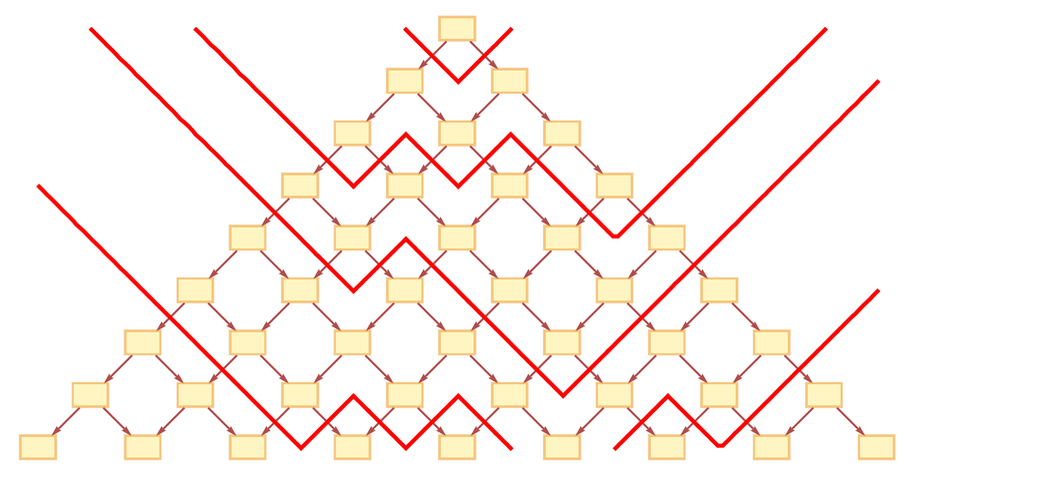✕ ```CloudGet["https://wolfr.am/LcADnk1u"]; upTriangleGraph = diamondCausalGraphPlot[9, {0, 0}, {}, # &, "Up", ImageSize -> 450]; Show[ drawFoliation[ Graph[upTriangleGraph, VertexLabelStyle -> Directive[8, Bold], VertexSize -> .45], {{1}, {1, 3, 6, 10, 2, 4, 5}, {1, 3, 6, 10, 2, 4, 5, 8, 9, 15, 13, 14, 19, 20, 26, 7, 12}, {1, 3, 6, 10, 2, 4, 5, 8, 9, 15, 13, 14, 19, 20, 26, 7, 12, 11, 17, 21, 18, 25, 24, 27, 32, 34, 28, 33, 16, 23, 31, 35, 42}}, Directive[AbsoluteThickness, Red]], ImageSize -> 550]```

We may know what the spatial hypergraph “typically” looks like. But perhaps with a weird enough foliation, it could be very different.

But for now, let’s ignore this (though it will be important later). And let’s just imagine we pick some “reasonable” foliation. Then we want to ask what the “projection” of the causal cone onto the instantaneous structure of space is. Or, in other words, what elements in space are affected by a particular event?

Let’s look at a specific example. Let’s consider the same rule and same causal cone as above, with the “flat” (“cosmological rest frame”) foliation: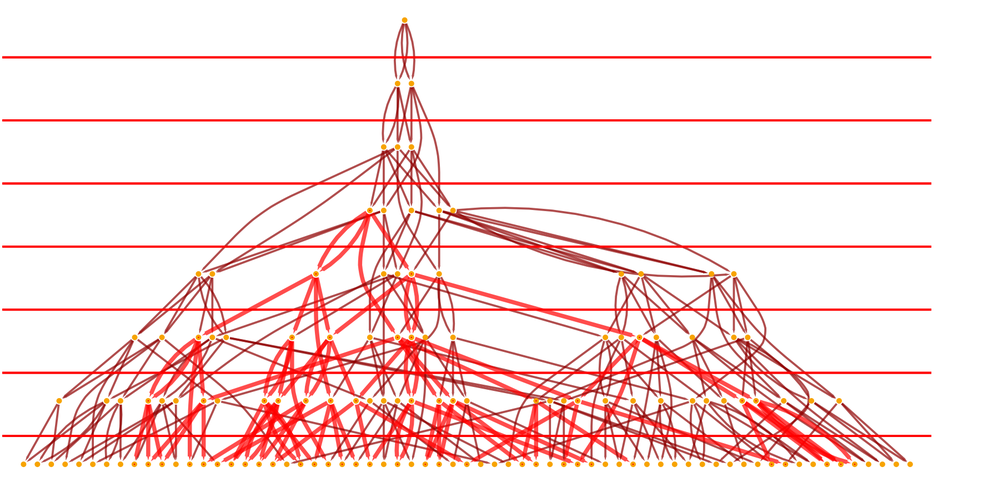✕ ```CloudGet["https://wolfr.am/KXgcRNRJ"]; With[{g = ResourceFunction[ "WolframModel"][{{{x, y}, {x, z}} -> {{x, z}, {x, w}, {y, w}, {z, w}}}, {{0, 0}, {0, 0}}, 8]["LayeredCausalGraph", AspectRatio -> 1/2, Epilog -> {Directive[Red], straightFoliationLines[{0.22, 0}, {0, 0}, (# &), {0, -2}]}]}, HighlightGraph[g, Style[Subgraph[g, VertexOutComponent[g, 10]], Red, Thick]]]```

Here are spatial hypergraphs associated with successive slices in this foliation, with the parts contained in the causal cone highlighted: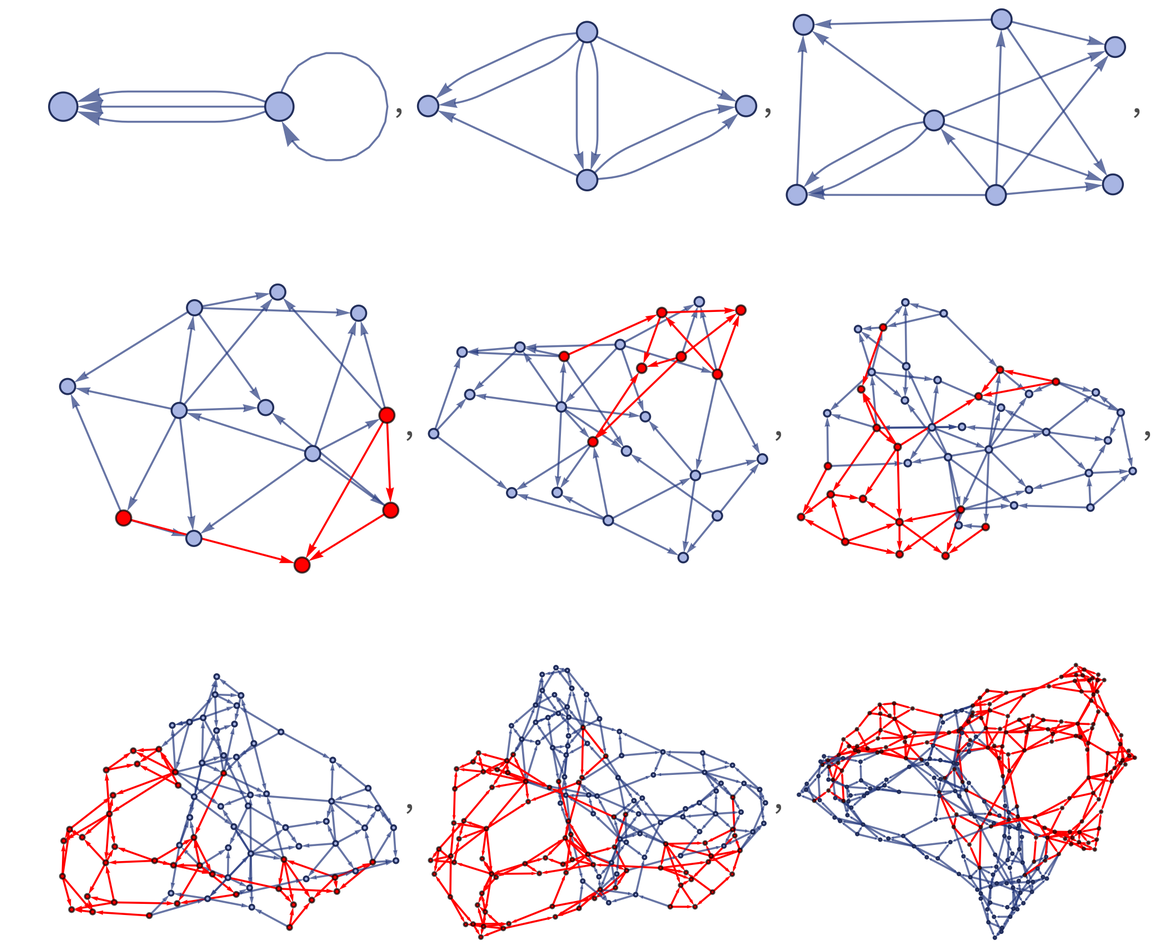✕ ``` Cell[CellGroupData[{Cell[BoxData[ RowBox[{ RowBox[{"EffectiveSpatialBall", "[", RowBox[{"wmo_", ",", "expr0_"}], "]"}], ":=", RowBox[{"Module", "[", RowBox[{ RowBox[{"{", RowBox[{ RowBox[{"t", "=", RowBox[{ "wmo", "[", "\"\\"", "]"}]}], ",", "fexprs"}], "}"}], ",", RowBox[{ RowBox[{"fexprs", "=", RowBox[{"wmo", "[", RowBox[{"\"\\"", ",", RowBox[{"-", "1"}]}], "]"}]}], ";", RowBox[{"Intersection", "[", RowBox[{ RowBox[{"Cases", "[", RowBox[{ RowBox[{"VertexOutComponent", "[", RowBox[{ RowBox[{ "wmo", "[", "\"\\"", "]"}], ",", RowBox[{"{", "expr0", "}"}]}], "]"}], ",", RowBox[{ RowBox[{"{", RowBox[{"\"\\"", ",", "n_"}], "}"}], ":>", "n"}]}], "]"}], ",", "fexprs"}], "]"}]}]}], "]"}]}]], "Input"], Cell[BoxData[ RowBox[{ RowBox[{"EffectiveSpatialAtomBall", "[", RowBox[{"wmo_", ",", "expr0_"}], "]"}], ":=", RowBox[{"Module", "[", RowBox[{ RowBox[{"{", RowBox[{ RowBox[{"t", "=", RowBox[{ "wmo", "[", "\"\\"", "]"}]}], ",", "fexprs"}], "}"}], ",", RowBox[{ RowBox[{"fexprs", "=", RowBox[{"wmo", "[", RowBox[{"\"\\"", ",", RowBox[{"-", "1"}]}], "]"}]}], ";", RowBox[{ RowBox[{"wmo", "[", "\"\\"", "]"}], "[", RowBox[{"[", RowBox[{"Intersection", "[", RowBox[{ RowBox[{"Cases", "[", RowBox[{ RowBox[{"VertexOutComponent", "[", RowBox[{ RowBox[{ "wmo", "[", "\"\\"", "]"}], ",", RowBox[{"{", "expr0", "}"}]}], "]"}], ",", RowBox[{ RowBox[{"{", RowBox[{"\"\\"", ",", "n_"}], "}"}], ":>", "n"}]}], "]"}], ",", "fexprs"}], "]"}], "]"}], "]"}]}]}], "]"}]}]], "Input"], Cell[BoxData[ RowBox[{ RowBox[{"EffectiveSpatialBallPlot", "[", RowBox[{"wmo_", ",", "expr0_"}], "]"}], ":=", RowBox[{"With", "[", RowBox[{ RowBox[{"{", RowBox[{"bb", "=", RowBox[{"EffectiveSpatialAtomBall", "[", RowBox[{"wmo", ",", "expr0"}], "]"}]}], "}"}], ",", RowBox[{"wmo", "[", RowBox[{"\"\\"", ",", RowBox[{"GraphHighlight", "\[Rule]", RowBox[{"Join", "[", RowBox[{"bb", ",", RowBox[{"Union", "[", RowBox[{"Catenate", "[", "bb", "]"}], "]"}]}], "]"}]}]}], "]"}]}], "]"}]}]], "Input"], Cell[BoxData[ RowBox[{"Table", "[", RowBox[{ RowBox[{"If", "[", RowBox[{ RowBox[{"t", "<", "4"}], ",", RowBox[{ RowBox[{"ResourceFunction", "[", "\"\\"", "]"}], "[", RowBox[{ RowBox[{"{", RowBox[{ RowBox[{"{", RowBox[{ RowBox[{"{", RowBox[{"x", ",", "y"}], "}"}], ",", RowBox[{"{", RowBox[{"x", ",", "z"}], "}"}]}], "}"}], "\[Rule]", RowBox[{"{", RowBox[{ RowBox[{"{", RowBox[{"x", ",", "z"}], "}"}], ",", RowBox[{"{", RowBox[{"x", ",", "w"}], "}"}], ",", RowBox[{"{", RowBox[{"y", ",", "w"}], "}"}], ",", RowBox[{"{", RowBox[{"z", ",", "w"}], "}"}]}], "}"}]}], "}"}], ",", RowBox[{"{", RowBox[{ RowBox[{"{", RowBox[{"0", ",", "0"}], "}"}], ",", RowBox[{"{", RowBox[{"0", ",", "0"}], "}"}]}], "}"}], ",", "t", ",", "\"\\""}], "]"}], ",", RowBox[{"EffectiveSpatialBallPlot", "[", RowBox[{ RowBox[{ RowBox[{ "ResourceFunction", "[", "\"\\"", "]"}], "[", RowBox[{ RowBox[{"{", RowBox[{ RowBox[{"{", RowBox[{ RowBox[{"{", RowBox[{"x", ",", "y"}], "}"}], ",", RowBox[{"{", RowBox[{"x", ",", "z"}], "}"}]}], "}"}], "\[Rule]", RowBox[{"{", RowBox[{ RowBox[{"{", RowBox[{"x", ",", "z"}], "}"}], ",", RowBox[{"{", RowBox[{"x", ",", "w"}], "}"}], ",", RowBox[{"{", RowBox[{"y", ",", "w"}], "}"}], ",", RowBox[{"{", RowBox[{"z", ",", "w"}], "}"}]}], "}"}]}], "}"}], ",", RowBox[{"{", RowBox[{ RowBox[{"{", RowBox[{"0", ",", "0"}], "}"}], ",", RowBox[{"{", RowBox[{"0", ",", "0"}], "}"}]}], "}"}], ",", "t"}], "]"}], ",", RowBox[{"{", RowBox[{"\"\\"", ",", "10"}], "}"}]}], "]"}]}], "]"}], ",", RowBox[{"{", RowBox[{"t", ",", "9"}], "}"}]}], "]"}]], "Input"] }, Open ]]```

For the first 3 slices the event that begins the causal cone hasn’t happened yet. But after that we start seeing the effect of the event, gradually spreading across successive spatial hypergraphs.

Yes, there are more subtleties ahead. But basically what we’re seeing here is the expansion of the light cone with time. So now we’ve got to ask the critical question: how fast does the edge of this light cone actually expand? How much space does it traverse at each unit in time? In other words, what is the effective speed of light here?

It is already clear from the pictures above that this is a somewhat subtle question. But let’s begin with an even more basic issue. The speed of light is something we measure in units like meters per second. But what we can potentially get from our model is instead a speed in spatial hypergraph edges per causal edge. We can say that each causal edge corresponds to a certain elementary time elapsing. And as soon as we quote the elementary time in seconds—say 100–100 s—we’re basically defining the second. And similarly, we can say that each spatial hypergraph edge corresponds to a distance of a certain elementary length. But now imagine that in t elementary times the light cone in the hypergraph has advanced by α t spatial hypergraph edges, or α t elementary lengths. What is α t in meters? It has to be α c t, where c is the speed of light, because in effect this defines the speed of light.

In other words, it’s at some level a tautology to say that the light cone in the spatial hypergraph advances at the speed of light—because this is the definition of the speed of light. But it’s more complicated than that. In continuum space there’s nothing inconsistent about saying that the speed of light is the same in every direction, everywhere. But when we’re projecting our causal cone onto the spatial hypergraph we can’t really say that anymore. But to know what happens we have to figure out more about how to characterize space.

In our models it’s clear what causal effects there are, and even how they spread. But what’s far from clear is where in detail these effects show up in what we call space. We know what the causal cones are like; but we still have to figure out how they map into positions in space. And from that we can try to work out whether—relative to the way we set up space—there can be effects that go faster than light.

## How to Measure Distance

In a sense speeds are complicated to characterize in our models because positions and times are hard to define. But it’s useful to consider for a moment the much simpler case of cellular automata, where from the outset we just set up a grid in space and time. Given some cellular automaton, say with a random initial condition, we can ask how fast an effect can propagate. For example, if we change one cell in the initial condition, by how many cells per step can the effect of this expand? Here are a couple of typical results: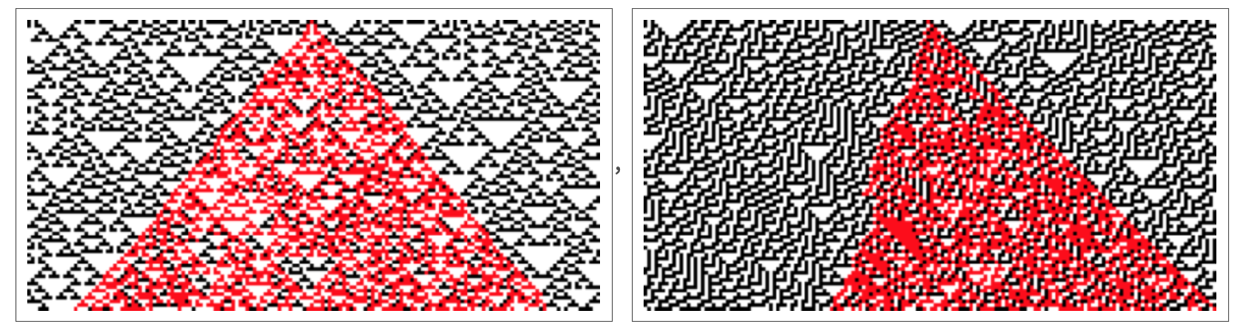✕ ```With[{u = RandomInteger[1, 160]}, SeedRandom; ArrayPlot[ Sum[(2 + (-1)^i) CellularAutomaton[#, ReplacePart[u, 80 -> i], 80], {i, 0, 1}], ColorRules -> {0 -> White, 4 -> Black, 1 -> Red, 3 -> Red}, ImageSize -> 330]] & /@ {22, 30}```

The actual speed of expansion can vary, but in both cases the absolute maximum speed is 1 cell/step. And this is very straightforward to understand from the underlying rules for the cellular automata:✕ `RulePlot[CellularAutomaton[#], ImageSize -> 300] & /@ {22, 30}`

In both cases, the rule for each step “reaches” one cell away, so 1 cell/step is the maximum rate at which effects can propagate.

There’s something somewhat analogous that happens in our models. Consider a rule like: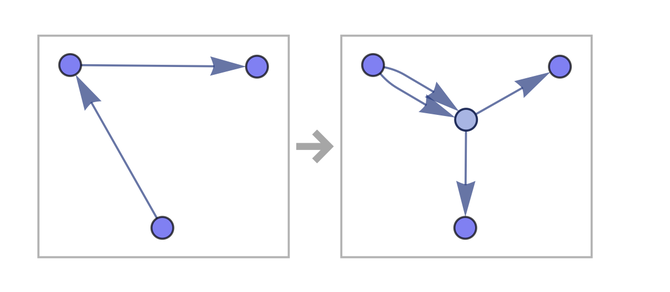✕ ```RulePlot[ResourceFunction[ "WolframModel"][{{{1, 2}, {2, 3}} -> {{2, 4}, {2, 4}, {4, 1}, {4, 3}}}]]```

A bit like in the cellular automaton, the rule only “reaches” a limited number of connections away. And what this means is that in each updating event only elements within a certain range of connections can “have an effect” on each other. But inevitably this is only a very local statement. Because while the structure of the rule implies that effects can only spread a certain distance in a single update there is nothing that says what the “relative geometry” of successive updates will be, and what connection might be connected to what. Unlike in a cellular automaton where the global spatial structure is predefined, in our models there is no immediate global consequence to the fact that the rules are fundamentally local with respect to the hypergraph.

It should be mentioned that the rules don’t strictly even have to be local. If the left-hand side is disconnected, as in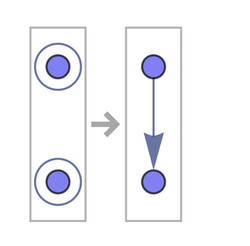✕ `RulePlot[ResourceFunction["WolframModel"][{{x}, {y}} -> {{x, y}}]]`

then in a sense any individual update can pick up elements from anywhere in the spatial hypergraph—even disconnected parts. And as a result, something anywhere in the universe can immediately affect something anywhere else. But with a rule like this, there doesn’t seem to be a way to build up anything with the kind of locality properties that characterize what we think of as space.

OK, but given a spatial hypergraph, how do we figure out “how far” it is from one node to another? That’s a subtle question. It’s easy to figure out the graph distance: just find the geodesic path from one node to another and see how many connections it involves. But this is just an abstract distance on the hypergraph: now the question is how it relates to a distance we might measure “physically”, say with something like a ruler.

It’s a tricky thing: we have a hypergraph that is supposed to represent everything in the universe. And now we want something—presumably itself part of the hypergraph—to measure a distance in the hypergraph. In traditional treatments of relativity it’s common to think of measuring distances by looking at arrival times of light signals or photons. But this implicitly assumes that there’s an underlying structure of space, and photons are simply being added in to probe it. In our models, however, the photons have to themselves be part of the spatial hypergraph: they’re in a sense just “pieces of space”, albeit presumably with appropriate generalized topological properties.

Or, put another way: when we directly study the spatial hypergraph, we’re operating far below the level of things like photons. But if we’re going to compare what we see in spatial hypergraphs with actual distance measurements in physics we’re going to have to find some way to bridge the gap. Or, in other words, we need to find some adequate proxy for physical distance that we can compute directly on the spatial hypergraph.

A simple possibility that we’ve used a lot in practice in exploring our models is just graph distance, though with one wrinkle. The wrinkle is as follows: our hypergraphs represent collections of relations between elements, and we assume that these relations are ordered—so that the hyperedges in our hypergraphs are directed hyperedges. But in computing “physical-like distances” we ignore the directedness, and treat what we have as an undirected hypergraph. In the limit of sufficiently large hypergraphs, this shouldn’t make much difference, although it seems as if including directedness information may let us look at the analog of spinors, while the undirected case corresponds to ordinary vectors, which are what we’re more familiar with in terms of measuring distances.

So is there any other proxy for distance that we could use? Actually, there are several. But one that may be particularly good is directly derived from the causal graph. It’s in some ways the analog of what we might do in traditional discussions of relativity where we imagine a grid of beacons signaling to each other over a limited period of time. In terms of our models we can say that it’s the analog of a branchial distance for the causal graph.

Here’s how it works. Construct a causal graph, say: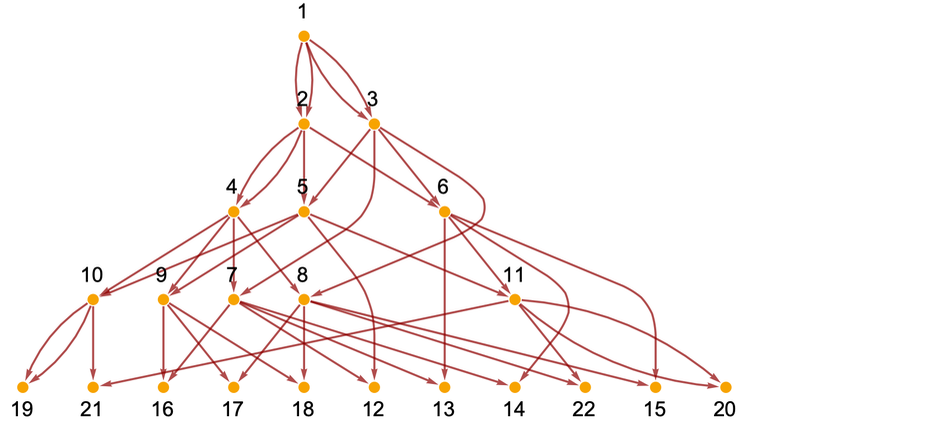✕ ```ResourceFunction[ "WolframModel"][{{{x, y}, {x, z}} -> {{x, z}, {x, w}, {y, w}, {z, w}}}, {{0, 0}, {0, 0}}, 5]["LayeredCausalGraph", AspectRatio -> 1/2, VertexLabels -> Automatic]```

Now look at the events in the last slice shown here. For each pair of events look at their ancestry, i.e. at what previous event(s) led to them. If a particular pair of events have a common ancestor on the step before, connect them. The result in this case is the graph: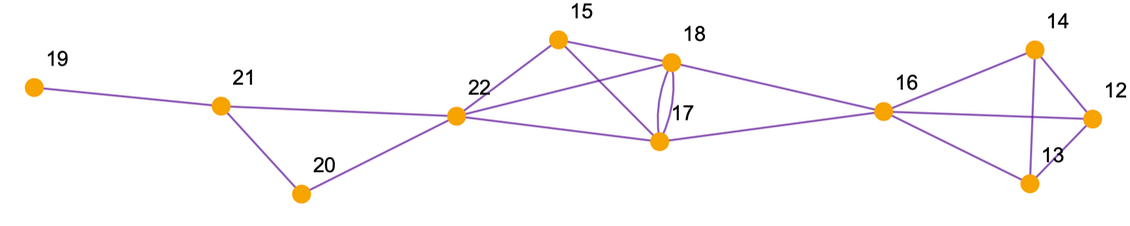✕ ```PacletInstall["SetReplace"]; << SetReplace`; SpatialReconstruction[wmo_WolframModelEvolutionObject, dt_Integer : 1] := Module[{cg = wmo["CausalGraph"], ceg = wmo["EventGenerations"], ev0, ev1, oc}, ev0 = First /@ Position[-(ceg - Max[ceg]), dt]; ev1 = First /@ Position[-(ceg - Max[ceg]), 0]; oc = Select[Rest[VertexOutComponent[cg, #]], MemberQ[ev1, #] &] & /@ ev0; Graph[ WolframPhysicsProjectStyleData["SpatialGraph", "Function"][ Graph[ev1, Flatten[(UndirectedEdge @@@ Subsets[#, {2}]) & /@ oc]]], VertexStyle -> WolframPhysicsProjectStyleData["CausalGraph", "VertexStyle"], EdgeStyle -> Blend[{First[ WolframPhysicsProjectStyleData["SpatialGraph", "EdgeLineStyle"]], WolframPhysicsProjectStyleData["BranchialGraph", "EdgeStyle"]}]]] Graph[SpatialReconstruction[ WolframModel[{{{x, y}, {x, z}} -> {{x, z}, {x, w}, {y, w}, {z, w}}}, {{0, 0}, {0, 0}}, 5], 1], VertexLabels -> Automatic]```

One can think of this as a “reconstruction of space”, based on the causal graph. In an appropriate limit, it should be essentially the same as the structure of space associated with the original hypergraph—though with this small a graph the spatial hypergraph still looks quite different: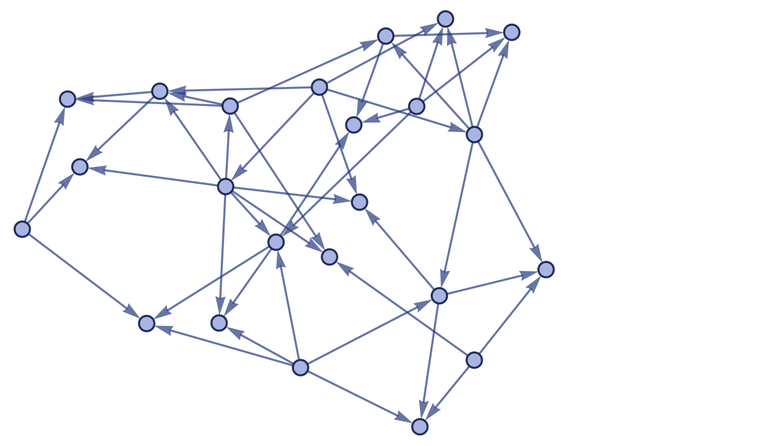✕ ```ResourceFunction[ "WolframModel"][{{{x, y}, {x, z}} -> {{x, z}, {x, w}, {y, w}, {z, w}}}, {{0, 0}, {0, 0}}, 5]["FinalStatePlot"]```

It’s slightly complicated, but it’s important to understand the differences between these various graphs. In the underlying spatial hypergraph, the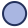nodes are the fundamental elements in our model—that we’ve dubbed above “atoms of space”. The hyperedges connecting these nodes correspond to the relations between the elements. In the causal graph, however, the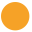nodes represent updating events, joined by edges that represent the causal relationships between these events.

The “spatial reconstruction graph” has events as its nodes, but it has a new kind of edge connecting these nodes—an edge that represents immediate common ancestry of the events. Whenever an event “causes” other events one can think of the first event as “starting an elementary light cone” that contains the other events. The causal graph represents the way that the elementary light cones are “knitted together” by the evolution of the system, and, more specifically, by the overlap of effects of different events on relations in the spatial hypergraph. The spatial reconstruction graph now uses the fact that two events lie in the same elementary light cone as a way to infer that the events are “close together”, as recorded by an edge in the spatial reconstruction graph.

There is an analogy here to our discussions of quantum mechanics. In talking about quantum mechanics we start from multiway graphs whose nodes are quantum states, and then we look at (“time”) slices through these graphs, and construct branchial graphs from them—with two states being joined in this branchial graph when they have an immediate common ancestor in the multiway graph. Or, said another way: in the branchial graph we join states that are in the same elementary “entanglement cone”. And the resulting branchial graph can be viewed as a map of a space of quantum states and their entanglements: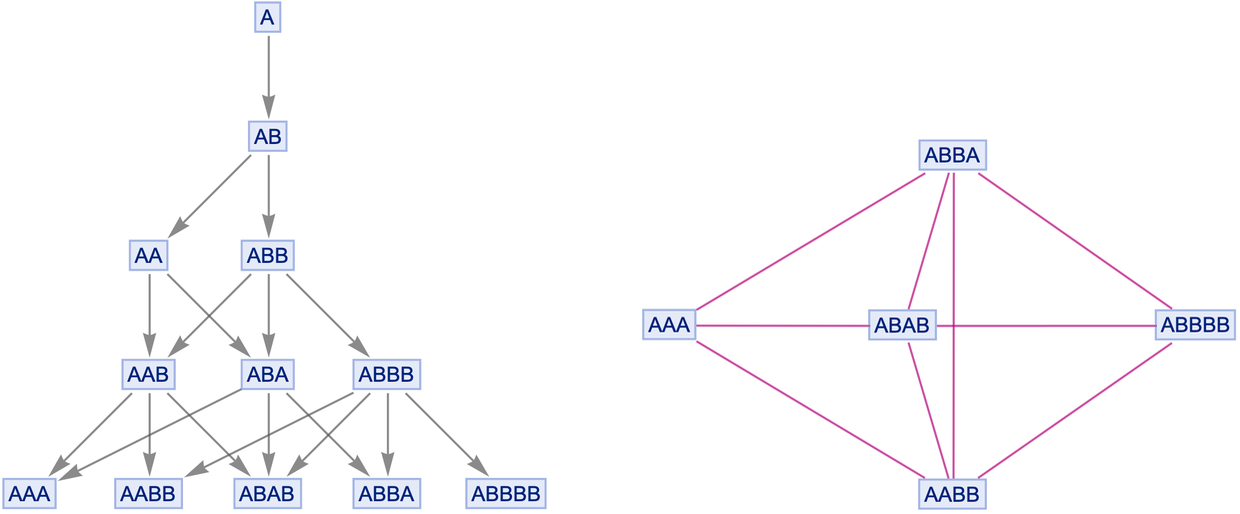✕ ```Cell[CellGroupData[{Cell[BoxData[ RowBox[{ RowBox[{ RowBox[{"ResourceFunction", "[", "\"\\"", "]"}], "[", RowBox[{ RowBox[{"{", RowBox[{ RowBox[{"\"\\"", "\[Rule]", "\"\\""}], ",", RowBox[{"\"\\"", "\[Rule]", "\"\\""}]}], "}"}], ",", "\"\\"", ",", "4", ",", "\"\\""}], "]"}], "//", "LayeredGraphPlot"}]], "Input"], Cell[BoxData[ RowBox[{ RowBox[{"ResourceFunction", "[", "\"\\"", "]"}], "[", RowBox[{ RowBox[{"{", RowBox[{ RowBox[{"\"\\"", "\[Rule]", "\"\\""}], ",", RowBox[{"\"\\"", "\[Rule]", "\"\\""}]}], "}"}], ",", "\"\\"", ",", "4", ",", "\"\\""}], "]"}]], "Input"] }, Open ]]```

The spatial reconstruction graph is the same idea: it’s like a branchial graph, but computed from the causal graph, rather than from a multiway graph. (Aficionados of our project may notice that the spatial reconstruction graph is a new kind of graph that we haven’t drawn before—and in which we’re coloring the edges with a new, purple color that happens to be a blend of our “branchial pink” with the blue-gray used for spatial hypergraphs.)

In the spatial reconstruction graph shown above, we’re joining events when they have a common ancestor one step before. But we can generalize the notion of a spatial reconstruction graph (or, for that matter, a branchial graph) by allowing common ancestors more than one step back.

In the case we showed above, going even two steps back causes almost all events to have common ancestors: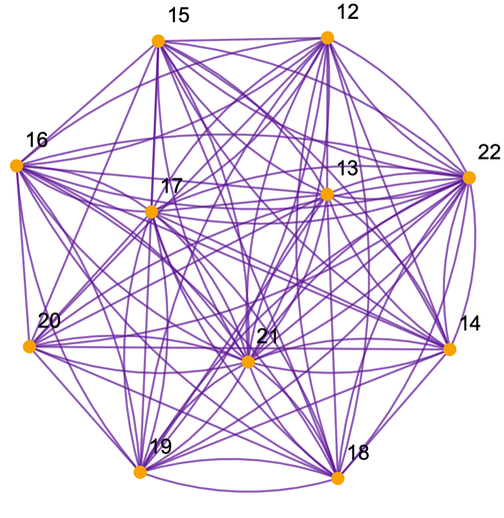✕ ```PacletInstall["SetReplace"]; << SetReplace`; SpatialReconstruction[wmo_WolframModelEvolutionObject, dt_Integer : 1] := Module[{cg = wmo["CausalGraph"], ceg = wmo["EventGenerations"], ev0, ev1, oc}, ev0 = First /@ Position[-(ceg - Max[ceg]), dt]; ev1 = First /@ Position[-(ceg - Max[ceg]), 0]; oc = Select[Rest[VertexOutComponent[cg, #]], MemberQ[ev1, #] &] & /@ ev0; Graph[ WolframPhysicsProjectStyleData["SpatialGraph", "Function"][ Graph[ev1, Flatten[(UndirectedEdge @@@ Subsets[#, {2}]) & /@ oc]]], VertexStyle -> WolframPhysicsProjectStyleData["CausalGraph", "VertexStyle"], EdgeStyle -> Blend[{First[ WolframPhysicsProjectStyleData["SpatialGraph", "EdgeLineStyle"]], WolframPhysicsProjectStyleData["BranchialGraph", "EdgeStyle"]}]]] Graph[SpatialReconstruction[ ResourceFunction[ "WolframModel"][{{{x, y}, {x, z}} -> {{x, z}, {x, w}, {y, w}, {z, w}}}, {{0, 0}, {0, 0}}, 5], 2], VertexLabels -> Automatic]```

And indeed if we go enough steps back, every event will inevitably share a common ancestor: the “big bang” event that started the evolution of the system.

Let’s say we have a rule that leads to a sequence of spatial hypergraphs: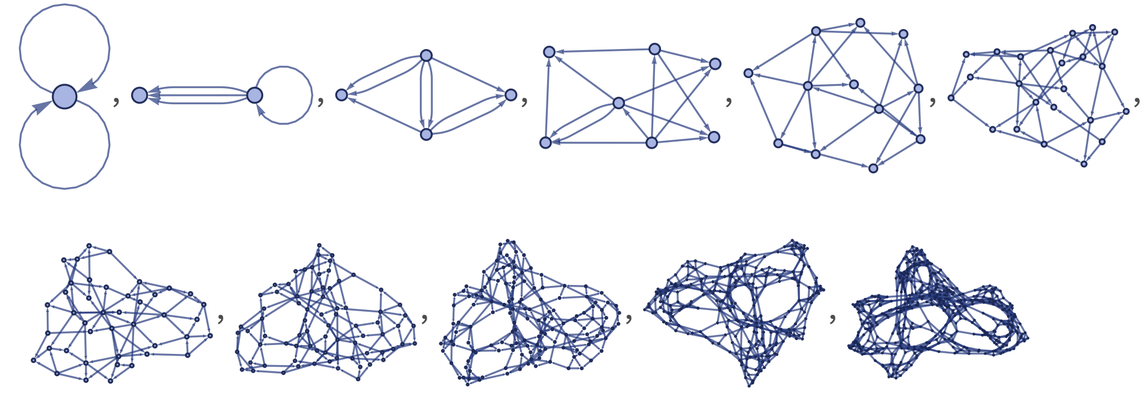✕ ```ResourceFunction[ "WolframModel"][{{{x, y}, {x, z}} -> {{x, z}, {x, w}, {y, w}, {z, w}}}, {{0, 0}, {0, 0}}, 10]["StatesPlotsList", ImageSize -> Tiny]```

We can compare these with the spatial reconstruction graphs that we get from the causal graph for this system. Here are the results on successive steps, allowing a “lookback” of 2 steps: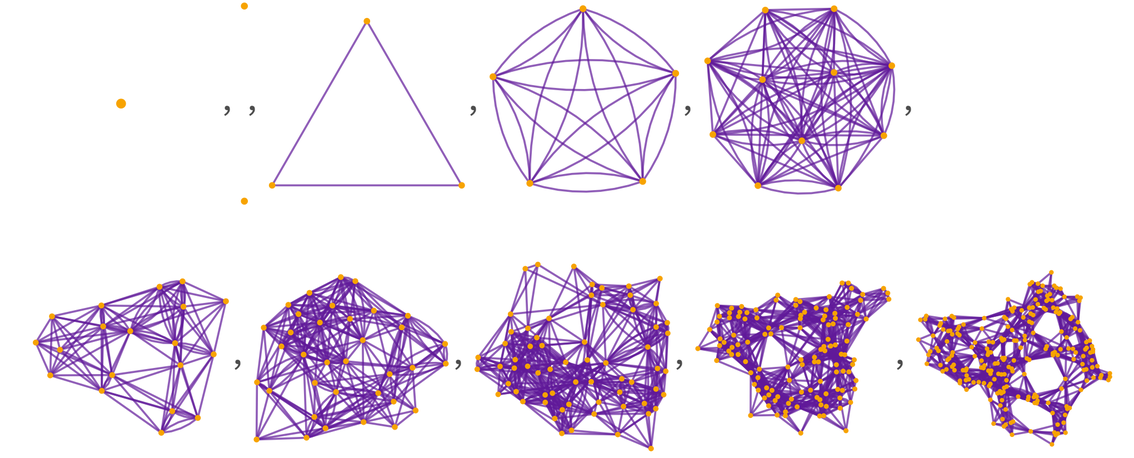✕ ```PacletInstall["SetReplace"]; << SetReplace`; SpatialReconstruction[wmo_WolframModelEvolutionObject, dt_Integer : 1] := Module[{cg = wmo["CausalGraph"], ceg = wmo["EventGenerations"], ev0, ev1, oc}, ev0 = First /@ Position[-(ceg - Max[ceg]), dt]; ev1 = First /@ Position[-(ceg - Max[ceg]), 0]; oc = Select[Rest[VertexOutComponent[cg, #]], MemberQ[ev1, #] &] & /@ ev0; Graph[ WolframPhysicsProjectStyleData["SpatialGraph", "Function"][ Graph[ev1, Flatten[(UndirectedEdge @@@ Subsets[#, {2}]) & /@ oc]]], VertexStyle -> WolframPhysicsProjectStyleData["CausalGraph", "VertexStyle"], EdgeStyle -> Blend[{First[ WolframPhysicsProjectStyleData["SpatialGraph", "EdgeLineStyle"]], WolframPhysicsProjectStyleData["BranchialGraph", "EdgeStyle"]}]]] Table[Graph[ SpatialReconstruction[ ResourceFunction[ "WolframModel"][{{{x, y}, {x, z}} -> {{x, z}, {x, w}, {y, w}, {z, w}}}, {{0, 0}, {0, 0}}, t], 2], ImageSize -> Tiny], {t, 10}]```

And as the number of steps increases, there is increasingly commonality between the spatial hypergraph and the spatial reconstruction graph—though they are not identical.

It’s worth pointing out that the spatial reconstruction graphs we’ve drawn certainly aren’t the only ways to get a proxy for physical distances. One simple change is that we can look at common successors, rather than common ancestors.

Another thing is to look not at a spatial hypergraph in which the nodes are elements and the hyperedges are relations, but instead at a “dual spatial hypergraph” in which the nodes are relations and the hyperedges are associated with elements, with each (unordered) hyperedge recording which relations share a given element.

For example, for the spatial hypergraph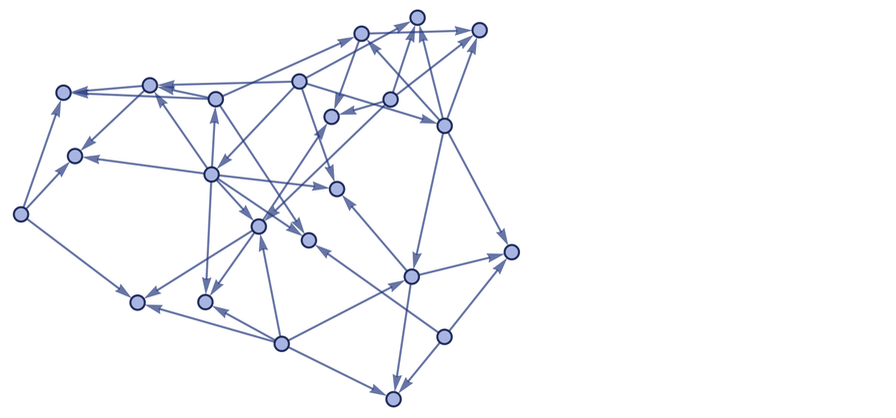✕ ```ResourceFunction[ "WolframModel"][{{{x, y}, {x, z}} -> {{x, z}, {x, w}, {y, w}, {z, w}}}, {{0, 0}, {0, 0}}, 5, "FinalStatePlot"]```

the corresponding dual spatial hypergraph is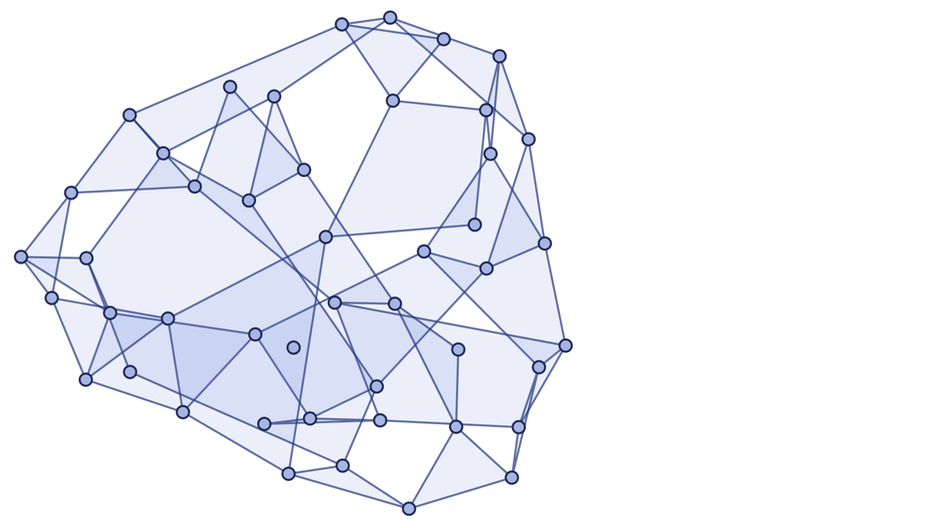✕ ```Cell[CellGroupData[{Cell[BoxData[ RowBox[{ RowBox[{"RelationsElementsHypergraph", "[", "wmo_", "]"}], ":=", RowBox[{"Module", "[", RowBox[{ RowBox[{"{", RowBox[{ RowBox[{"ix", "=", RowBox[{"wmo", "[", RowBox[{"\"\\"", ",", RowBox[{"-", "1"}]}], "]"}]}], ",", "es"}], "}"}], ",", RowBox[{"Values", "[", RowBox[{"Merge", "[", RowBox[{ RowBox[{"Association", "@@@", RowBox[{"(", RowBox[{"Thread", "/@", RowBox[{"Thread", "[", RowBox[{ RowBox[{ RowBox[{"wmo", "[", "\"\\"", "]"}], "[", RowBox[{"[", "ix", "]"}], "]"}], "\[Rule]", "ix"}], "]"}]}], ")"}]}], ",", "Identity"}], "]"}], "]"}]}], "]"}]}]], "Input"], Cell[BoxData[ RowBox[{ RowBox[{"UnorderedHypergraphPlot", "[", RowBox[{"h_", ",", "opts___"}], "]"}], ":=", RowBox[{ RowBox[{"ResourceFunction", "[", "\"\\"", "]"}], "[", RowBox[{"h", ",", "opts", ",", RowBox[{"\"\\"", "\[Rule]", "0"}], ",", RowBox[{"EdgeStyle", "\[Rule]", RowBox[{"<|", RowBox[{ RowBox[{"{", RowBox[{"_", ",", "_", ",", RowBox[{"_", ".."}]}], "}"}], "\[Rule]", "Transparent"}], "|>"}]}], ",", RowBox[{"\"\\"", "\[Rule]", RowBox[{"<|", RowBox[{ RowBox[{"{", RowBox[{"_", ",", "_", ",", RowBox[{"_", ".."}]}], "}"}], "\[Rule]", RowBox[{"Directive", "[", RowBox[{ RowBox[{"Hue", "[", RowBox[{"0.63", ",", "0.66", ",", "0.81"}], "]"}], ",", RowBox[{"Opacity", "[", "0.1", "]"}], ",", RowBox[{"EdgeForm", "[", RowBox[{"Directive", "[", RowBox[{ RowBox[{"Hue", "[", RowBox[{"0.63", ",", "0.7", ",", "0.5"}], "]"}], ",", RowBox[{"Opacity", "[", "0.7", "]"}]}], "]"}], "]"}]}], "]"}]}], "|>"}]}]}], "]"}]}]], "Input"], Cell[BoxData[ RowBox[{"UnorderedHypergraphPlot", "[", RowBox[{"RelationsElementsHypergraph", "[", RowBox[{ RowBox[{"ResourceFunction", "[", "\"\\"", "]"}], "[", RowBox[{ RowBox[{"{", RowBox[{ RowBox[{"{", RowBox[{ RowBox[{"{", RowBox[{"x", ",", "y"}], "}"}], ",", RowBox[{"{", RowBox[{"x", ",", "z"}], "}"}]}], "}"}], "\[Rule]", RowBox[{"{", RowBox[{ RowBox[{"{", RowBox[{"x", ",", "z"}], "}"}], ",", RowBox[{"{", RowBox[{"x", ",", "w"}], "}"}], ",", RowBox[{"{", RowBox[{"y", ",", "w"}], "}"}], ",", RowBox[{"{", RowBox[{"z", ",", "w"}], "}"}]}], "}"}]}], "}"}], ",", RowBox[{"{", RowBox[{ RowBox[{"{", RowBox[{"0", ",", "0"}], "}"}], ",", RowBox[{"{", RowBox[{"0", ",", "0"}], "}"}]}], "}"}], ",", "5"}], "]"}], "]"}], "]"}]], "Input"] }, Open ]]```

and the sequence of dual spatial hypergraphs corresponding to the evolution above is: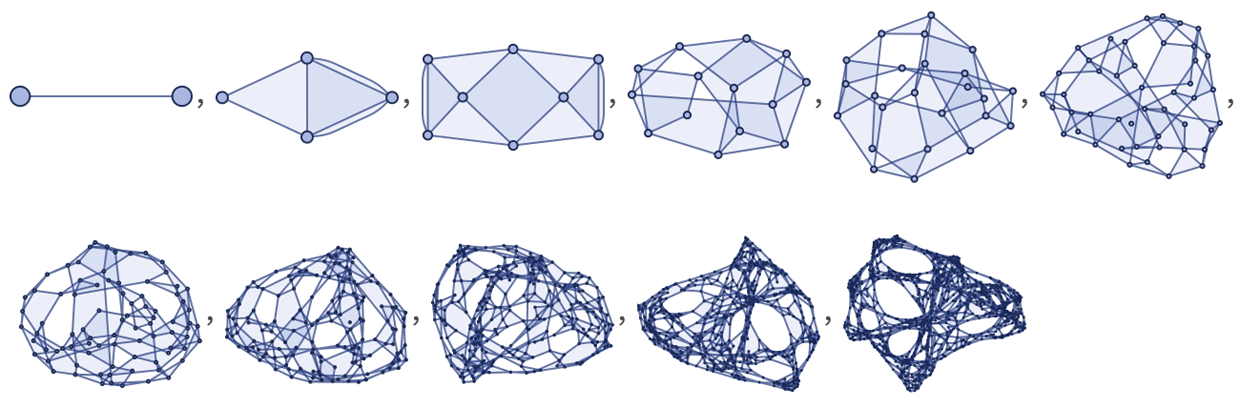✕ ```Cell[CellGroupData[{Cell[BoxData[ RowBox[{ RowBox[{"RelationsElementsHypergraph", "[", "wmo_", "]"}], ":=", RowBox[{"Module", "[", RowBox[{ RowBox[{"{", RowBox[{ RowBox[{"ix", "=", RowBox[{"wmo", "[", RowBox[{"\"\\"", ",", RowBox[{"-", "1"}]}], "]"}]}], ",", "es"}], "}"}], ",", RowBox[{"Values", "[", RowBox[{"Merge", "[", RowBox[{ RowBox[{"Association", "@@@", RowBox[{"(", RowBox[{"Thread", "/@", RowBox[{"Thread", "[", RowBox[{ RowBox[{ RowBox[{"wmo", "[", "\"\\"", "]"}], "[", RowBox[{"[", "ix", "]"}], "]"}], "\[Rule]", "ix"}], "]"}]}], ")"}]}], ",", "Identity"}], "]"}], "]"}]}], "]"}]}]], "Input"], Cell[BoxData[ RowBox[{ RowBox[{"UnorderedHypergraphPlot", "[", RowBox[{"h_", ",", "opts___"}], "]"}], ":=", RowBox[{ RowBox[{"ResourceFunction", "[", "\"\\"", "]"}], "[", RowBox[{"h", ",", "opts", ",", RowBox[{"\"\\"", "\[Rule]", "0"}], ",", RowBox[{"EdgeStyle", "\[Rule]", RowBox[{"<|", RowBox[{ RowBox[{"{", RowBox[{"_", ",", "_", ",", RowBox[{"_", ".."}]}], "}"}], "\[Rule]", "Transparent"}], "|>"}]}], ",", RowBox[{"\"\\"", "\[Rule]", RowBox[{"<|", RowBox[{ RowBox[{"{", RowBox[{"_", ",", "_", ",", RowBox[{"_", ".."}]}], "}"}], "\[Rule]", RowBox[{"Directive", "[", RowBox[{ RowBox[{"Hue", "[", RowBox[{"0.63", ",", "0.66", ",", "0.81"}], "]"}], ",", RowBox[{"Opacity", "[", "0.1", "]"}], ",", RowBox[{"EdgeForm", "[", RowBox[{"Directive", "[", RowBox[{ RowBox[{"Hue", "[", RowBox[{"0.63", ",", "0.7", ",", "0.5"}], "]"}], ",", RowBox[{"Opacity", "[", "0.7", "]"}]}], "]"}], "]"}]}], "]"}]}], "|>"}]}]}], "]"}]}]], "Input"], Cell[BoxData[ RowBox[{"Table", "[", RowBox[{ RowBox[{"Show", "[", RowBox[{ RowBox[{"UnorderedHypergraphPlot", "[", RowBox[{"RelationsElementsHypergraph", "[", RowBox[{ RowBox[{ "ResourceFunction", "[", "\"\\"", "]"}], "[", RowBox[{ RowBox[{"{", RowBox[{ RowBox[{"{", RowBox[{ RowBox[{"{", RowBox[{"x", ",", "y"}], "}"}], ",", RowBox[{"{", RowBox[{"x", ",", "z"}], "}"}]}], "}"}], "\[Rule]", RowBox[{"{", RowBox[{ RowBox[{"{", RowBox[{"x", ",", "z"}], "}"}], ",", RowBox[{"{", RowBox[{"x", ",", "w"}], "}"}], ",", RowBox[{"{", RowBox[{"y", ",", "w"}], "}"}], ",", RowBox[{"{", RowBox[{"z", ",", "w"}], "}"}]}], "}"}]}], "}"}], ",", RowBox[{"{", RowBox[{ RowBox[{"{", RowBox[{"0", ",", "0"}], "}"}], ",", RowBox[{"{", RowBox[{"0", ",", "0"}], "}"}]}], "}"}], ",", "t"}], "]"}], "]"}], "]"}], ",", RowBox[{"ImageSize", "\[Rule]", "Tiny"}]}], "]"}], ",", RowBox[{"{", RowBox[{"t", ",", "0", ",", "10"}], "}"}]}], "]"}]], "Input"] }, Open ]]```

There are still other possibilities, particularly if one goes “below” the causal graph, and starts looking not just at causal relations between whole events, but also at causal relations between specific relations in the underlying spatial hypergraph.

But the main takeaway is that there are various proxies we can use for physical distance. In the limit of a sufficiently large system, all of them should give compatible results. But when we’re dealing with small graphs, they won’t quite agree, and so we may not be sure what we should say the distance between two things is.

## Causal Balls vs. Geodesic Balls

To measure speed, we basically have to divide distance by elapsed time. But, as I just discussed at some length, when we’re constructing space and time from something lower level, it’s not straightforward to say exactly what we mean by distance and by elapsed time, and how different possibilities will correspond to what we’d actually measure, say at a human scale.

But as a first approximation, let’s just ask about the effect of a single event. The effect of this event is captured by a causal cone: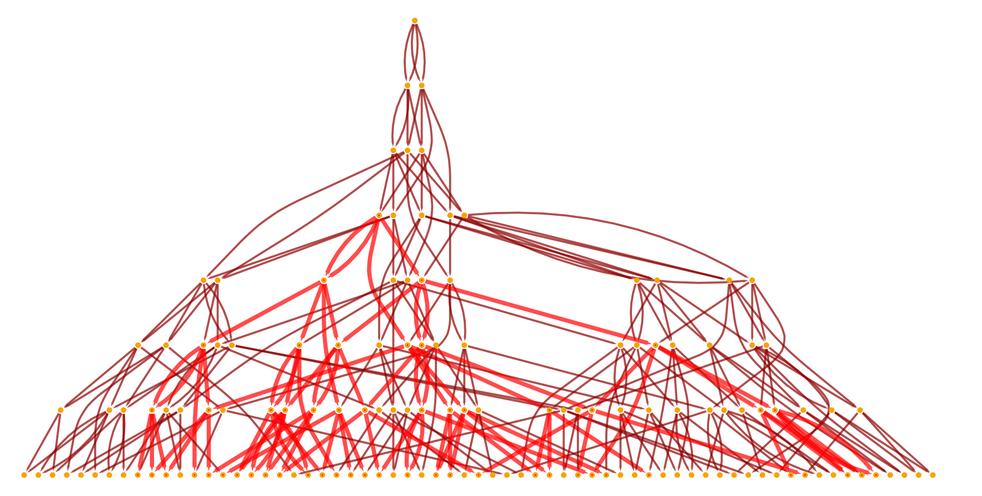✕ ```With[{g = ResourceFunction[ "WolframModel"][{{{x, y}, {x, z}} -> {{x, z}, {x, w}, {y, w}, {z, w}}}, {{0, 0}, {0, 0}}, 8]["LayeredCausalGraph", AspectRatio -> 1/2]}, HighlightGraph[g, Style[Subgraph[g, VertexOutComponent[g, 10]], Red, Thick]]]```

We can say that the elapsed time associated with a particular slice through this causal cone is the graph distance from the event at the top of the cone to events in this slice. (How the slice is chosen is determined by the reference frame we’re using.)

So now we want to see how far the effect of the event spreads in space. The first step is to “project” the causal cone onto some representation of “instantaneous space”. We can do this with the ordinary spatial hypergraph: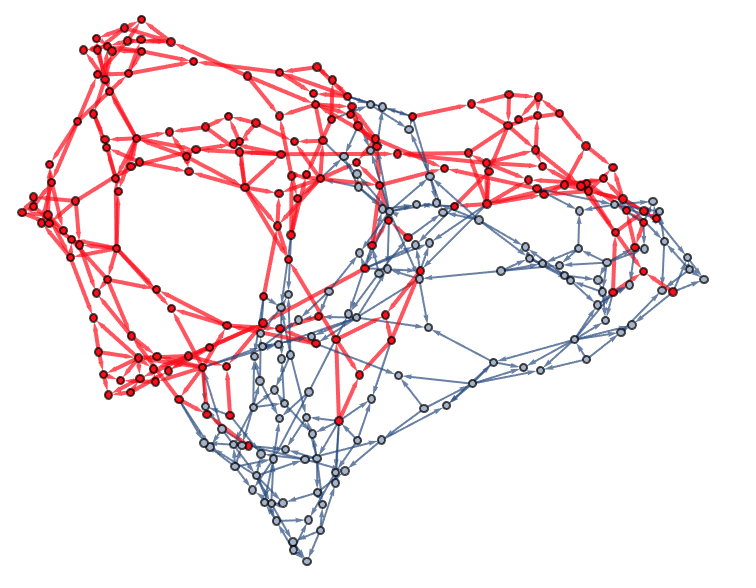✕ ```Cell[CellGroupData[{Cell[BoxData[ RowBox[{ RowBox[{"EffectiveSpatialBall", "[", RowBox[{"wmo_", ",", "expr0_"}], "]"}], ":=", RowBox[{"Module", "[", RowBox[{ RowBox[{"{", RowBox[{ RowBox[{"t", "=", RowBox[{ "wmo", "[", "\"\\"", "]"}]}], ",", "fexprs"}], "}"}], ",", RowBox[{ RowBox[{"fexprs", "=", RowBox[{"wmo", "[", RowBox[{"\"\\"", ",", RowBox[{"-", "1"}]}], "]"}]}], ";", RowBox[{"Intersection", "[", RowBox[{ RowBox[{"Cases", "[", RowBox[{ RowBox[{"VertexOutComponent", "[", RowBox[{ RowBox[{ "wmo", "[", "\"\\"", "]"}], ",", RowBox[{"{", "expr0", "}"}]}], "]"}], ",", RowBox[{ RowBox[{"{", RowBox[{"\"\\"", ",", "n_"}], "}"}], ":>", "n"}]}], "]"}], ",", "fexprs"}], "]"}]}]}], "]"}]}]], "Input"], Cell[BoxData[ RowBox[{ RowBox[{"EffectiveSpatialAtomBall", "[", RowBox[{"wmo_", ",", "expr0_"}], "]"}], ":=", RowBox[{"Module", "[", RowBox[{ RowBox[{"{", RowBox[{ RowBox[{"t", "=", RowBox[{ "wmo", "[", "\"\\"", "]"}]}], ",", "fexprs"}], "}"}], ",", RowBox[{ RowBox[{"fexprs", "=", RowBox[{"wmo", "[", RowBox[{"\"\\"", ",", RowBox[{"-", "1"}]}], "]"}]}], ";", RowBox[{ RowBox[{"wmo", "[", "\"\\"", "]"}], "[", RowBox[{"[", RowBox[{"Intersection", "[", RowBox[{ RowBox[{"Cases", "[", RowBox[{ RowBox[{"VertexOutComponent", "[", RowBox[{ RowBox[{ "wmo", "[", "\"\\"", "]"}], ",", RowBox[{"{", "expr0", "}"}]}], "]"}], ",", RowBox[{ RowBox[{"{", RowBox[{"\"\\"", ",", "n_"}], "}"}], ":>", "n"}]}], "]"}], ",", "fexprs"}], "]"}], "]"}], "]"}]}]}], "]"}]}]], "Input"], Cell[BoxData[ RowBox[{ RowBox[{"HighlightEffectiveSpatialBallPlot", "[", RowBox[{"wmo_", ",", "expr0_"}], "]"}], ":=", RowBox[{"With", "[", RowBox[{ RowBox[{"{", RowBox[{ RowBox[{"bb", "=", RowBox[{"EffectiveSpatialAtomBall", "[", RowBox[{"wmo", ",", "expr0"}], "]"}]}], ",", RowBox[{"edges", "=", RowBox[{"wmo", "[", "\"\\"", "]"}]}]}], "}"}], ",", RowBox[{"HighlightGraph", "[", RowBox[{ RowBox[{"Graph", "[", RowBox[{"DirectedEdge", "@@@", RowBox[{"Catenate", "[", RowBox[{ RowBox[{ RowBox[{"Partition", "[", RowBox[{"#", ",", "2", ",", "1"}], "]"}], "&"}], "/@", "edges"}], "]"}]}], "]"}], ",", RowBox[{"Style", "[", RowBox[{ RowBox[{"DirectedEdge", "@@@", RowBox[{"Join", "[", RowBox[{"bb", ",", RowBox[{"Union", "[", RowBox[{"Catenate", "[", "bb", "]"}], "]"}]}], "]"}]}], ",", "Red", ",", "Thick"}], "]"}]}], "]"}]}], "]"}]}]], "Input"], Cell[BoxData[ RowBox[{"HighlightEffectiveSpatialBallPlot", "[", RowBox[{ RowBox[{ RowBox[{"ResourceFunction", "[", "\"\\"", "]"}], "[", RowBox[{ RowBox[{"{", RowBox[{ RowBox[{"{", RowBox[{ RowBox[{"{", RowBox[{"x", ",", "y"}], "}"}], ",", RowBox[{"{", RowBox[{"x", ",", "z"}], "}"}]}], "}"}], "\[Rule]", RowBox[{"{", RowBox[{ RowBox[{"{", RowBox[{"x", ",", "z"}], "}"}], ",", RowBox[{"{", RowBox[{"x", ",", "w"}], "}"}], ",", RowBox[{"{", RowBox[{"y", ",", "w"}], "}"}], ",", RowBox[{"{", RowBox[{"z", ",", "w"}], "}"}]}], "}"}]}], "}"}], ",", RowBox[{"{", RowBox[{ RowBox[{"{", RowBox[{"0", ",", "0"}], "}"}], ",", RowBox[{"{", RowBox[{"0", ",", "0"}], "}"}]}], "}"}], ",", "9"}], "]"}], ",", RowBox[{"{", RowBox[{"\"\\"", ",", "10"}], "}"}]}], "]"}]], "Input"] }, Open ]]```

But to align with the most obvious notion of “elapsed time” in the causal cone it’s better to use the spatial reconstruction graph, whose nodes, just like those of the causal graph, are events: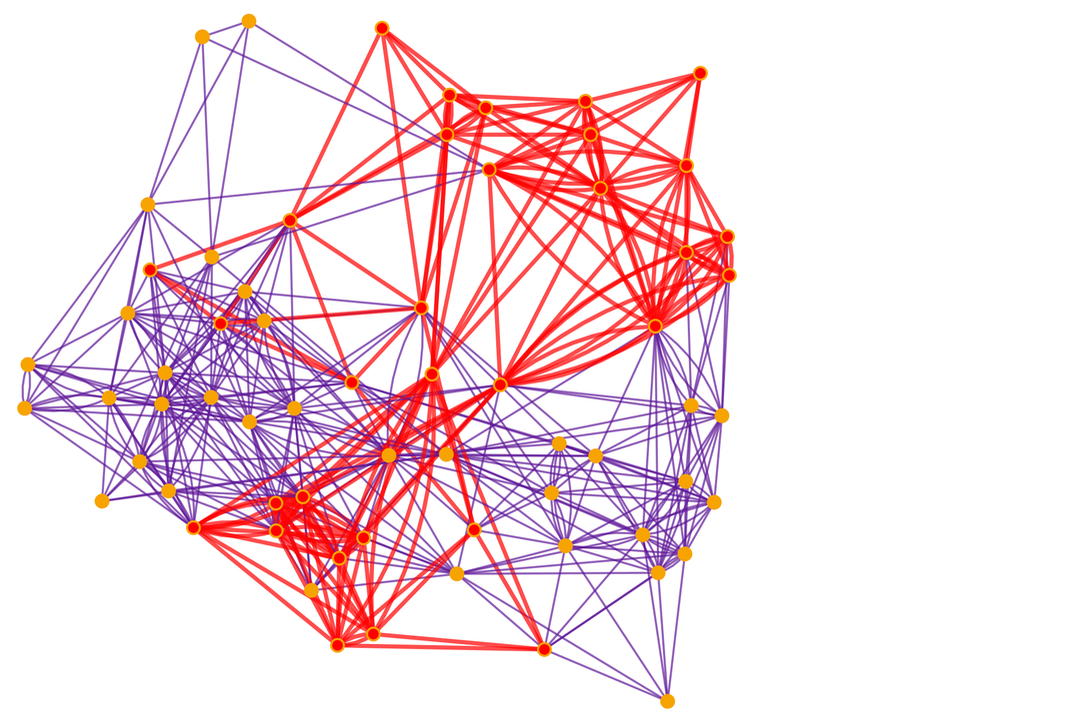✕ ```PacletInstall["SetReplace"]; << SetReplace`; SpatialReconstruction[wmo_WolframModelEvolutionObject, dt_Integer : 1] := Module[{cg = wmo["CausalGraph"], ceg = wmo["EventGenerations"], ev0, ev1, oc}, ev0 = First /@ Position[-(ceg - Max[ceg]), dt]; ev1 = First /@ Position[-(ceg - Max[ceg]), 0]; oc = Select[Rest[VertexOutComponent[cg, #]], MemberQ[ev1, #] &] & /@ ev0; Graph[ WolframPhysicsProjectStyleData["SpatialGraph", "Function"][ Graph[ev1, Flatten[(UndirectedEdge @@@ Subsets[#, {2}]) & /@ oc]]], VertexStyle -> WolframPhysicsProjectStyleData["CausalGraph", "VertexStyle"], EdgeStyle -> Blend[{First[ WolframPhysicsProjectStyleData["SpatialGraph", "EdgeLineStyle"]], WolframPhysicsProjectStyleData["BranchialGraph", "EdgeStyle"]}]]] With[{sg = SpatialReconstruction[ ResourceFunction[ "WolframModel"][{{{x, y}, {x, z}} -> {{x, z}, {x, w}, {y, w}, {z, w}}}, {{0, 0}, {0, 0}}, 8], 2]}, HighlightGraph[sg, Style[Subgraph[sg, With[{g = ResourceFunction[ "WolframModel"][{{{x, y}, {x, z}} -> {{x, z}, {x, w}, {y, w}, {z, w}}}, {{0, 0}, {0, 0}}, 8][ "LayeredCausalGraph"]}, VertexOutComponent[g, 10]]], Red, Thick]]]```

Let’s “watch the intersection grow” from successive slices of the causal cone, projected onto spatial reconstruction graphs: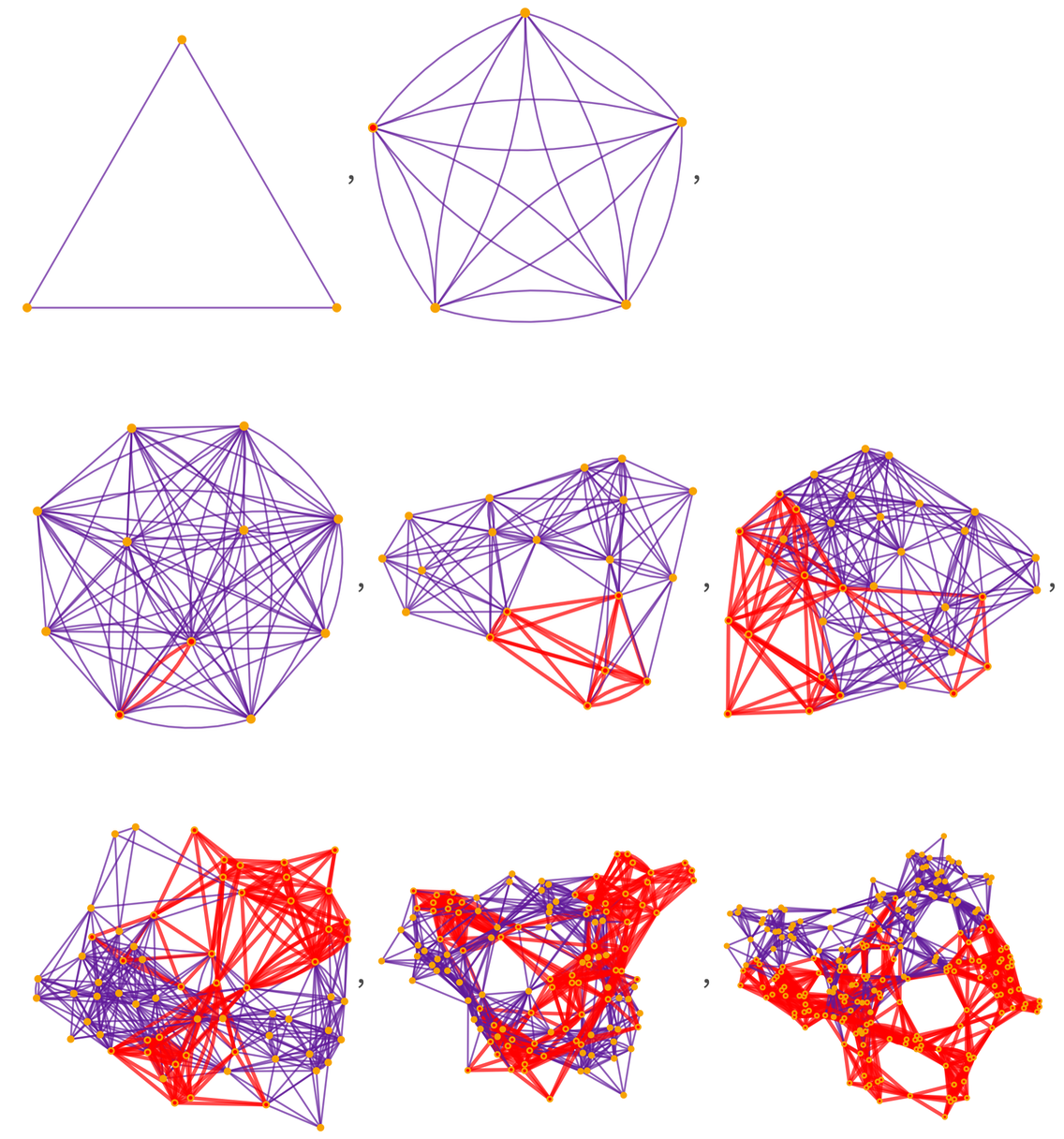✕ ```PacletInstall["SetReplace"]; << SetReplace`; SpatialReconstruction[wmo_WolframModelEvolutionObject, dt_Integer : 1] := Module[{cg = wmo["CausalGraph"], ceg = wmo["EventGenerations"], ev0, ev1, oc}, ev0 = First /@ Position[-(ceg - Max[ceg]), dt]; ev1 = First /@ Position[-(ceg - Max[ceg]), 0]; oc = Select[Rest[VertexOutComponent[cg, #]], MemberQ[ev1, #] &] & /@ ev0; Graph[ WolframPhysicsProjectStyleData["SpatialGraph", "Function"][ Graph[ev1, Flatten[(UndirectedEdge @@@ Subsets[#, {2}]) & /@ oc]]], VertexStyle -> WolframPhysicsProjectStyleData["CausalGraph", "VertexStyle"], EdgeStyle -> Blend[{First[ WolframPhysicsProjectStyleData["SpatialGraph", "EdgeLineStyle"]], WolframPhysicsProjectStyleData["BranchialGraph", "EdgeStyle"]}]]] Table[With[{sg = SpatialReconstruction[ ResourceFunction[ "WolframModel"][{{{x, y}, {x, z}} -> {{x, z}, {x, w}, {y, w}, {z, w}}}, {{0, 0}, {0, 0}}, t], 2]}, HighlightGraph[sg, Style[Subgraph[sg, With[{g = ResourceFunction[ "WolframModel"][{{{x, y}, {x, z}} -> {{x, z}, {x, w}, {y, w}, {z, w}}}, {{0, 0}, {0, 0}}, 10][ "LayeredCausalGraph"]}, VertexOutComponent[g, 10]]], Red, Thick]]], {t, 3, 10}]```

Now the question we have to ask is: how “wide” is that area of intersection? The pictures make it clear that it’s not trivial to answer—or even precisely define—that question. Yes, in the continuum limit of sufficiently large graphs we’d better get something that looks like a light cone in continuum space, but it’s far from trivial how that limiting process might work.

We can think of the intersection of the causal cone with a spatial slice as defining a “causal ball” at a particular “time”. But now within that spatial slice we can ask about graph distances. So, for example, given a particular point in the slice we can ask what points lie within a certain graph distance of it—or, in other words, what the geodesic ball of some radius around that point is.

And fundamentally the computation of “speed” is all about the comparison of the “widths” of causal balls and of geodesic balls. Another way to look at this is to say that given two points in the causal ball (that by definition are produced from a common ancestor some “time” back) we want to know the “spatial distance” between them.

There are several ways we can assess “width”. We could compute the boundaries of causal balls, and for each point see what the “geodesically most distant” point is. Or we can just compute geodesic (i.e. spatial reconstruction graph) distances between all pairs of points in the causal ball. Here are distributions of these distances for each step shown above: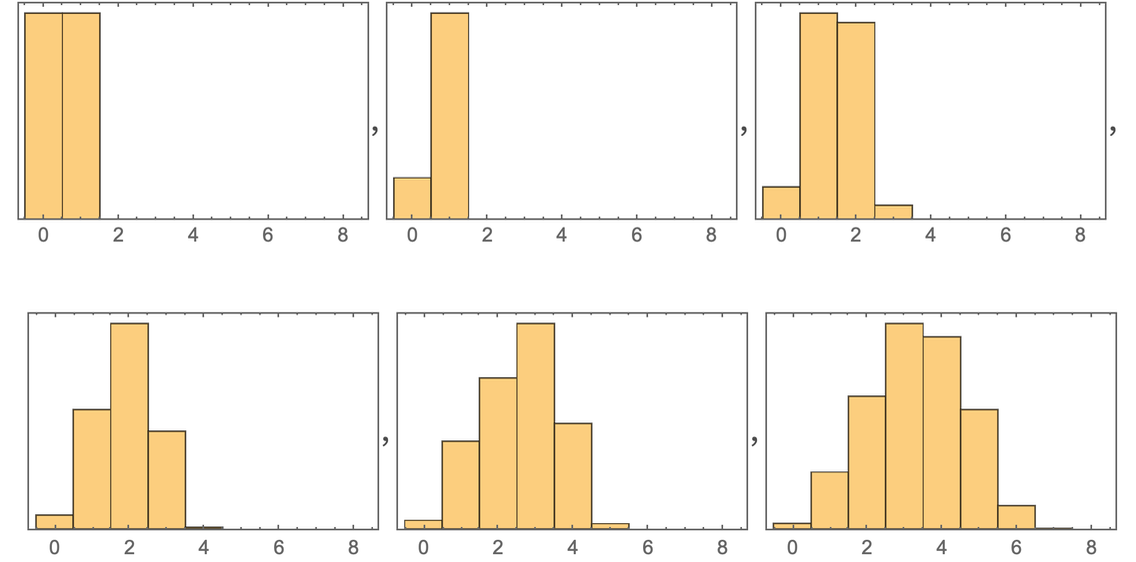✕ ```PacletInstall["SetReplace"]; << SetReplace`; SpatialReconstruction[wmo_WolframModelEvolutionObject, dt_Integer : 1] := Module[{cg = wmo["CausalGraph"], ceg = wmo["EventGenerations"], ev0, ev1, oc}, ev0 = First /@ Position[-(ceg - Max[ceg]), dt]; ev1 = First /@ Position[-(ceg - Max[ceg]), 0]; oc = Select[Rest[VertexOutComponent[cg, #]], MemberQ[ev1, #] &] & /@ ev0; Graph[ WolframPhysicsProjectStyleData["SpatialGraph", "Function"][ Graph[ev1, Flatten[(UndirectedEdge @@@ Subsets[#, {2}]) & /@ oc]]], VertexStyle -> WolframPhysicsProjectStyleData["CausalGraph", "VertexStyle"], EdgeStyle -> Blend[{First[ WolframPhysicsProjectStyleData["SpatialGraph", "EdgeLineStyle"]], WolframPhysicsProjectStyleData["BranchialGraph", "EdgeStyle"]}]]] Table[Histogram[ Flatten[Module[{sg = SpatialReconstruction[ ResourceFunction[ "WolframModel"][{{{x, y}, {x, z}} -> {{x, z}, {x, w}, {y, w}, {z, w}}}, {{0, 0}, {0, 0}}, t], 2], pts, dm}, pts = Intersection[ With[{g = ResourceFunction[ "WolframModel"][{{{x, y}, {x, z}} -> {{x, z}, {x, w}, {y, w}, {z, w}}}, {{0, 0}, {0, 0}}, 10][ "LayeredCausalGraph"]}, VertexOutComponent[g, 10]], VertexList[sg]]; Outer[GraphDistance[sg, #1, #2] &, pts, pts]]], {1}, PlotRange -> {{-.5, 8.5}, Automatic}, Frame -> True, FrameTicks -> {Automatic, None}], {t, 5, 10}]```

How do we assess the “speed of light” from this? We might imagine we should look at the “outer edge” of this histogram, and see how it advances with “time”. If we do that, we get the result: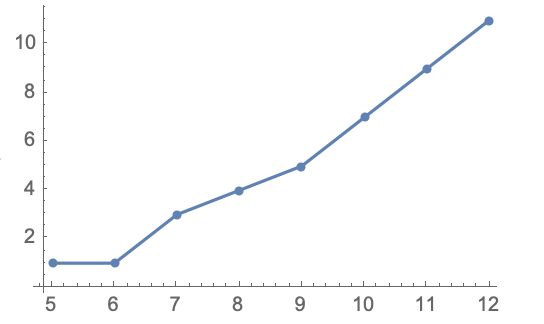✕ ```Cell[CellGroupData[{Cell[BoxData[{ RowBox[{ RowBox[{"PacletInstall", "[", "\"\\"", "]"}], ";", RowBox[{"<<", "SetReplace`"}], ";"}], "\n", RowBox[{ RowBox[{"SpatialReconstruction", "[", RowBox[{"wmo_WolframModelEvolutionObject", ",", RowBox[{"dt_Integer", ":", "1"}]}], "]"}], ":=", RowBox[{"Module", "[", RowBox[{ RowBox[{"{", RowBox[{ RowBox[{"cg", "=", RowBox[{"wmo", "[", "\"\\"", "]"}]}], ",", RowBox[{"ceg", "=", RowBox[{"wmo", "[", "\"\\"", "]"}]}], ",", "ev0", ",", "ev1", ",", "oc"}], "}"}], ",", RowBox[{ RowBox[{"ev0", "=", RowBox[{"First", "/@", RowBox[{"Position", "[", RowBox[{ RowBox[{"-", RowBox[{"(", RowBox[{"ceg", "-", RowBox[{"Max", "[", "ceg", "]"}]}], ")"}]}], ",", "dt"}], "]"}]}]}], ";", "\[IndentingNewLine]", RowBox[{"ev1", "=", RowBox[{"First", "/@", RowBox[{"Position", "[", RowBox[{ RowBox[{"-", RowBox[{"(", RowBox[{"ceg", "-", RowBox[{"Max", "[", "ceg", "]"}]}], ")"}]}], ",", "0"}], "]"}]}]}], ";", "\[IndentingNewLine]", RowBox[{"oc", "=", RowBox[{ RowBox[{ RowBox[{"Select", "[", RowBox[{ RowBox[{"Rest", "[", RowBox[{"VertexOutComponent", "[", RowBox[{"cg", ",", "#"}], "]"}], "]"}], ",", RowBox[{ RowBox[{"MemberQ", "[", RowBox[{"ev1", ",", "#"}], "]"}], "&"}]}], "]"}], "&"}], "/@", "ev0"}]}], ";", RowBox[{"Graph", "[", RowBox[{ RowBox[{ RowBox[{"WolframPhysicsProjectStyleData", "[", RowBox[{"\"\\"", ",", "\"\\""}], "]"}], "[", RowBox[{"Graph", "[", RowBox[{"ev1", ",", RowBox[{"Flatten", "[", RowBox[{ RowBox[{ RowBox[{"(", RowBox[{"UndirectedEdge", "@@@", RowBox[{"Subsets", "[", RowBox[{"#", ",", RowBox[{"{", "2", "}"}]}], "]"}]}], ")"}], "&"}], "/@", "oc"}], "]"}]}], "]"}], "]"}], ",", RowBox[{"VertexStyle", "\[Rule]", RowBox[{"WolframPhysicsProjectStyleData", "[", RowBox[{"\"\\"", ",", "\"\\""}], "]"}]}], ",", RowBox[{"EdgeStyle", "\[Rule]", RowBox[{"Blend", "[", RowBox[{"{", RowBox[{ RowBox[{"First", "[", RowBox[{"WolframPhysicsProjectStyleData", "[", RowBox[{ "\"\\"", ",", "\"\\""}], "]"}], "]"}], ",", RowBox[{"WolframPhysicsProjectStyleData", "[", RowBox[{ "\"\\"", ",", "\"\\""}], "]"}]}], "}"}], "]"}]}]}], "]"}]}]}], "]"}]}], "\[IndentingNewLine]", RowBox[{"Table", "[", RowBox[{ RowBox[{"{", RowBox[{"t", ",", RowBox[{"Max", "[", RowBox[{"Flatten", "[", RowBox[{"Module", "[", RowBox[{ RowBox[{"{", RowBox[{ RowBox[{"sg", "=", RowBox[{"SpatialReconstruction", "[", RowBox[{ RowBox[{ RowBox[{ "ResourceFunction", "[", "\"\\"", "]"}], "[", RowBox[{ RowBox[{"{", RowBox[{ RowBox[{"{", RowBox[{ RowBox[{"{", RowBox[{"x", ",", "y"}], "}"}], ",", RowBox[{"{", RowBox[{"x", ",", "z"}], "}"}]}], "}"}], "\[Rule]", RowBox[{"{", RowBox[{ RowBox[{"{", RowBox[{"x", ",", "z"}], "}"}], ",", RowBox[{"{", RowBox[{"x", ",", "w"}], "}"}], ",", RowBox[{"{", RowBox[{"y", ",", "w"}], "}"}], ",", RowBox[{"{", RowBox[{"z", ",", "w"}], "}"}]}], "}"}]}], "}"}], ",", RowBox[{"{", RowBox[{ RowBox[{"{", RowBox[{"0", ",", "0"}], "}"}], ",", RowBox[{"{", RowBox[{"0", ",", "0"}], "}"}]}], "}"}], ",", "t"}], "]"}], ",", "2"}], "]"}]}], ",", "pts", ",", "dm"}], "}"}], ",", "\n", RowBox[{ RowBox[{"pts", "=", RowBox[{"Intersection", "[", RowBox[{ RowBox[{"With", "[", RowBox[{ RowBox[{"{", RowBox[{"g", "=", RowBox[{ RowBox[{ RowBox[{ "ResourceFunction", "[", "\"\\"", "]"}], "[", RowBox[{ RowBox[{"{", RowBox[{ RowBox[{"{", RowBox[{ RowBox[{"{", RowBox[{"x", ",", "y"}], "}"}], ",", RowBox[{"{", RowBox[{"x", ",", "z"}], "}"}]}], "}"}], "\[Rule]", RowBox[{"{", RowBox[{ RowBox[{"{", RowBox[{"x", ",", "z"}], "}"}], ",", RowBox[{"{", RowBox[{"x", ",", "w"}], "}"}], ",", RowBox[{"{", RowBox[{"y", ",", "w"}], "}"}], ",", RowBox[{"{", RowBox[{"z", ",", "w"}], "}"}]}], "}"}]}], "}"}], ",", RowBox[{"{", RowBox[{ RowBox[{"{", RowBox[{"0", ",", "0"}], "}"}], ",", RowBox[{"{", RowBox[{"0", ",", "0"}], "}"}]}], "}"}], ",", "12"}], "]"}], "[", "\"\\"", "]"}]}], "}"}], ",", RowBox[{"VertexOutComponent", "[", RowBox[{"g", ",", "10"}], "]"}]}], "]"}], ",", RowBox[{"VertexList", "[", "sg", "]"}]}], "]"}]}], ";", RowBox[{"Outer", "[", RowBox[{ RowBox[{ RowBox[{"GraphDistance", "[", RowBox[{"sg", ",", "#1", ",", "#2"}], "]"}], "&"}], ",", "pts", ",", "pts"}], "]"}]}]}], "]"}], "]"}], "]"}]}], "}"}], ",", RowBox[{"{", RowBox[{"t", ",", "5", ",", "12"}], "}"}]}], "]"}]}], "Input"], Cell[BoxData[ RowBox[{"ListLinePlot", "[", RowBox[{"%", ",", RowBox[{"Mesh", "\[Rule]", "All"}]}], "]"}]], "Input"] }, Open ]]```

But the full story is more complicated. Because, yes, the large-scale limit should be like a light cone, where we can measure the speed of light from its slope. But that doesn’t tell us about the “fine structure”. It doesn’t tell us whether at the edge of the causal ball, there are, for example, effectively space tunnels that “reach out” in the geodesic ball.

There are lots of subtle issues here. And there’s another issue in the example we’ve been using: not only does this involve a causal cone that’s expanding, but the “whole universe” (i.e. the whole spatial hypergraph) is also expanding.

So why not look at a simpler, “more static” case? Well, it isn’t so easy. Because in our models space is being “made dynamically”: it can’t really ever be “static”. At best we might imagine just having a rule that “trivially tills space”, touching elements but not “doing much” to them. But doing this introduces its own collection of artifacts.

## To Travel? To Communicate?

We’ve so far been talking mainly about the very low-level structure of spacetime, and how fast “threads of causality” can effectively “traverse space”. But if we’re actually going to be able to make use of faster-than-light phenomena, we’ve somehow got to “send something through them”. It’s not good enough to just have the structure of spacetime show some kind of faster-than-light phenomenon. We’ve got to be able to take something that we’ve chosen, and “send it through”.

When we talk about “traveling faster than light”, what we normally mean is that we can take ourselves, made of ordinary matter, atoms, etc. and transport that whole structure faster than light across space. A lower bar is to consider faster-than-light communication. To do this we have to be able to take some message that we have chosen, and convert it to a form that can be transferred across space faster than light.

To achieve true faster-than-light travel we presumably have to be able to construct some form of space tunnel in which the interior of the tunnel (and its entrance and exit) are sufficiently close to ordinary, flat space that they wouldn’t destroy us if we passed through them. It doesn’t seem difficult to imagine a spatial hypergraph that at least statically contains such a space tunnel. But it’s much more challenging to think about how this would be created dynamically.

But, OK, so let’s say we just want to send individual particles, like photons, through. Well, in our models it’s not clear that’s that much easier. Because it seems likely that even a single photon of ordinary energy will correspond to a quite large region in the spatial hypergraph. Presumably the “core” of the photon is some kind of persistent topological-like structure in the hypergraph. And to understand the propagation of a photon, what one should do is to trace this structure in the causal graph.

What about “communication without travel”? To propagate a “signal” in space requires that the signal has persistence of some kind, and the most obvious mechanism for such persistence would be a topological-like structure of the kind we assume exists in particles like photons. But—at least with some of the processes we’ll discuss below—there will be a premium on having our “signal carrier” involve as few underlying elements in the spatial hypergraph as possible. And one might imagine that this would be best achieved by something like the oligon particles that our models suggest could exist, and that involve many fewer elements in the spatial hypergraph than the particles we currently know about.

Of course, using “oligon radio” requires that we have some kind of transducer between ordinary familiar particles and oligons, and it’s not clear how that can be achieved.

There is probably a close connection in our models between what we might think of as black holes and what we might think of as particles. Quite what the details of this connection or correspondence are we don’t know yet, but both correspond to persistent structures “created purely from the structure of space”.

And it’s quite possible that there is a whole spectrum of persistent structures that don’t quite have characteristics like particles (indeed, our space tunnels would presumably be examples). The question of whether any of these can be used for communication is in a sense quite easy to define. To communicate, we need some structure in the causal graph that maintains information through time, and that has parts that can be arbitrarily changed. In other words, there needs to be some way to encode something like arbitrary patterns of bits in the causal graph, and have them persist.

## The Second Law of Thermodynamics

I’ve been interested in the Second Law of thermodynamics and its origins for nearly 50 years, and it’s remarkable that it now seems to be intimately connected to questions about going faster than light in our models. Fundamentally, what the Second Law says is that initially orderly configurations of things like molecules have a seemingly inexorable tendency to become more disorderly over time. And as we’ll discuss, this is something very general, ultimately rooted in the general phenomenon of computational irreducibility. And it doesn’t just apply to familiar things like molecules: it also applies—in our models—to the very structure of space.

So what’s the underlying story of the Second Law? I thought about this for many years, and finally in the 1990s got to the point where I felt I understood it. At first, the Second Law seems like a paradox: if the laws of physics are reversible then one would think that one could run any process as well backwards as forwards. Yet what the Second Law—and our experience—says is that things that start orderly tend to become more disorderly.

But here’s a simple model that illustrates what’s going on. Consider a cellular automaton that’s reversible (like the standard laws of physics), in the sense that for every configuration (or, actually, in this case, every pair of configurations) there’s both a unique successor in time, and a unique predecessor. Now start the cellular automaton from a simple initial condition: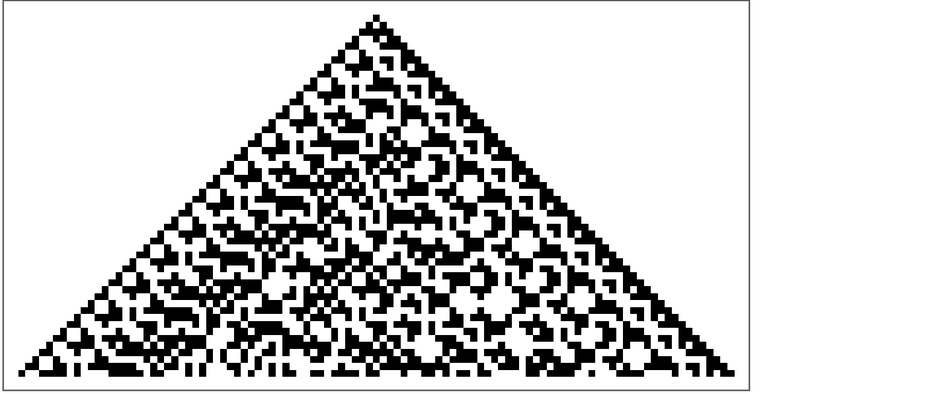✕ ```ArrayPlot[ CellularAutomaton[{10710, {2, {{0, 8, 0}, {4, 2, 1}}}, 1, 2}, {{{1}, {1}}, 0}, 51]]```

We see a fundamental computational fact: just like my favorite rule 30 cellular automaton, even though the initial condition is simple, the system behaves in a complex—and in many ways seemingly random—way.

But here’s the thing: this happens both if one runs it forward in time, and backward: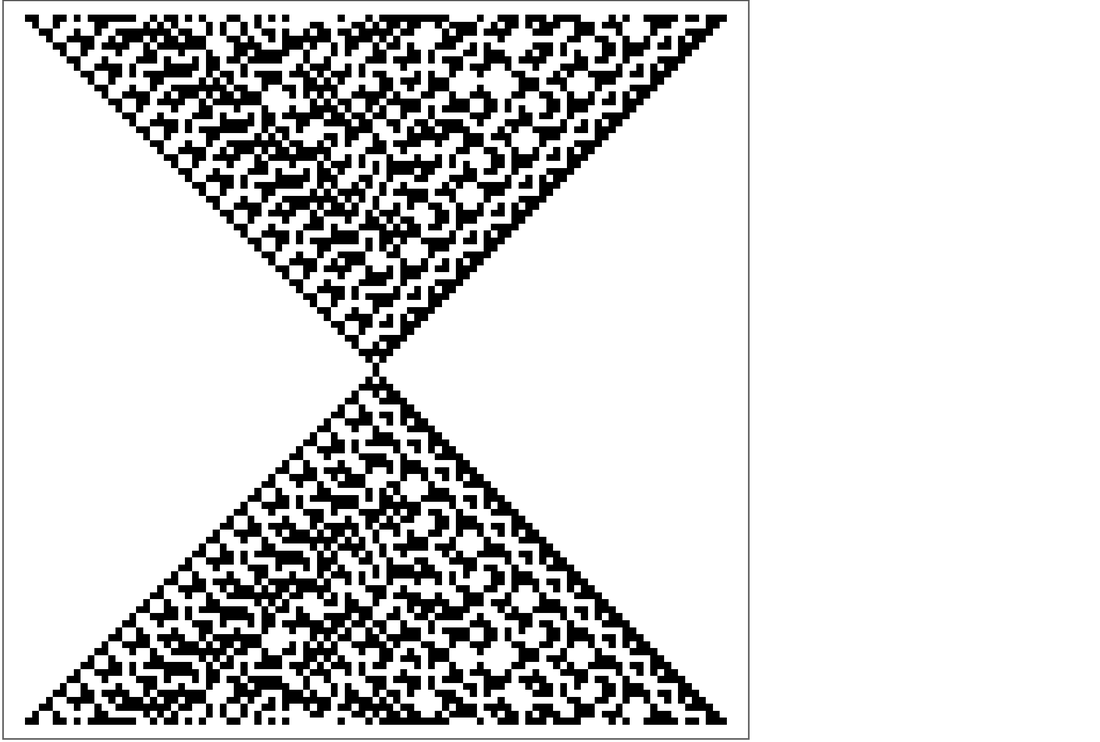✕ ```ArrayPlot[ CellularAutomaton[{10710, {2, {{0, 8, 0}, {4, 2, 1}}}, 1, 2}, Take[Reverse[ CellularAutomaton[{10710, {2, {{0, 8, 0}, {4, 2, 1}}}, 1, 2}, {{{1}, {1}}, 0}, 51]], 2], 101]]```

The randomization is just a feature of the execution of the rule—forward or backward. At some moment we have a configuration that looks simple. But when we run it forward in time, it “randomizes”. And the same happens if we go backward in time.

But why is there this apparent randomization? The evolution of the cellular automaton is effectively performing a computation. And to recognize a pattern in its output we have to do a computation too. But the point is that as soon as the evolution of the cellular automaton is computationally irreducible, recognizing a pattern inevitably takes an irreducible amount of computational work. It’s as if the cellular automaton is “encrypting” its initial condition—and so we have to do lots of computational work (perhaps even exponentially more than the cellular automaton itself) to be able to “decrypt” it.

It’s not that it’s impossible to invert the final state of the cellular automaton and find that it evolved from a simple state. It’s just that to do so takes an irreducible amount of computational work. And if we as observers are bounded in our computational capabilities we eventually won’t be able to do it—so we won’t be able to recognize that the system evolved from a simple state.

The picture above shows that once we have a simple state it’ll tend to evolve to a randomized state—just like we typically see. But the picture also shows that we can in principle set up a complicated initial state that will evolve to produce the simple state. So why don’t we typically see this happening in everyday life? It’s basically again a story of limited computational capabilities. Assume we have some computational system for setting up initial states. Then we can readily imagine that it would take only a limited number of computational operations to set up a simple state. But to set up the complicated and seemingly random state we’d need to be able to evolve to the simple state will take a lot more computational operations—and if we’re bounded in our computational capabilities we won’t be able to do it.

What we’ve seen here in a simple cellular automaton also happens with gas molecules—or idealized hard spheres. Say you start the molecules off in some special “simple” configuration, perhaps with all the molecules in the corner of a box. Then you let the system run, with molecules repeatedly colliding and so on. Looked at in a computational way, we can say that the process of evolution of the system is a computation—and we can expect that it will be a computationally irreducible one. And just like with the cellular automaton, any computationally bounded observer will inevitably see “Second-Law behavior”.

The traditional treatment of the Second Law talks a lot about entropy—which measures the number of possible configurations consistent with a measurement one makes on the system. (Needless to say, counting configurations is a lot easier in a fundamentally discrete system like a cellular automaton, than in standard real-number classical mechanics.) Well, if we measure the value of every single cell in a cellular automaton, there’s only one configuration consistent with our measurement—and given this measurement the whole past and future of the cellular automaton is determined, and we’ll always measure the same entropy for it.

But imagine instead that we can’t do such complete and precise measurements. Then there may be many configurations of the system consistent with the results we get. But the point is that if the actual configuration of the system is actually simple, computationally bounded measurements will readily be able to recognize this, and determine that there’s only one (or a few) configurations consistent with their results. But if the actual configuration is complicated, computationally bounded measurements won’t be able to determine which of many configurations one’s looking at. The result is that in terms of such measurements, the entropy of the system will be considered larger.

In the typical treatment of statistical mechanics over the past century one usually talks about “coarse-grained” measurements, but it’s always been a bit unclear what constitutes a “valid” coarse graining. I think what we now understand about computational irreducibility finally clarifies this, and lets us say what’s really going on in the Second Law: entropy seems to increase because the irreducible computation done by a system can’t successfully be “decrypted” by a computationally bounded observer.

Even back in the 1860s James Maxwell realized that if you could have a “demon” who basically tweaked individual molecules to unrandomize a gas, then you wouldn’t see Second-Law behavior. And, yes, if the demon had sufficient computational capabilities you could make this work; the Second Law relies on the idea that no such computational capabilities are available.

And as soon as the Second Law is in effect, one can start “assuming that things are random”, or, more specifically, that at least in some aggregate sense, the behavior of a system will follow statistical averages. This assumption is critical in deriving standard continuum fluid behavior from underlying molecular dynamics. And it’s also critical in deriving the continuum form of space from our underlying discrete model—and for deriving things like special and general relativity.

In other words, the fact that a fluid—or space—seems like a continuum to us is a reflection of the boundedness of our computational capabilities. If we could apply as much computation as the underlying molecules in the gas—or the discrete elements in space—then we could recognize many details that would go beyond the continuum description. But with bounded computation, we just end up describing fluids—or space—in terms of aggregate continuum parameters.

We talk about mechanical work—that involves patterns of motion in molecules that we can readily recognize as organized—being useful. And we talk about “heat”—that involves patterns of motion in molecules that seem random to us—as being fundamentally less useful. But this is really just a reflection of our computational boundedness. There is all sorts of detailed information in the motions associated with heat; it’s just that we can’t decode them to make use of them.

Today when we describe a gas we’ll typically say that it’s characterized by temperature and pressure. But that misses all the detail associated with the motion of molecules. And I suspect that in time the coarseness of our current descriptions of things like gases will come to seem quite naive. There’ll be all sorts of other features and parameters that effectively correspond to different kinds of computations performed on the configuration of molecules.

People sometimes talk disparagingly about the possible “heat death of the universe”, in which all of the orderly “mechanical work” motion has degraded into “heat”. But I don’t think that’s the right characterization. Yes, our current ways of looking at microscopic motions might only be to say it’s “generic heat”. But actually there’ll be all this rich structure in there, if only we were making the right measurements, and doing the right computations.

## Space Demons

If our models are going to reproduce what we currently know about physics, it’s got to be the case that in some large-scale limit, casual balls behave essentially like geodesic balls expanding at the speed of light. But this will only be an aggregate statement—that doesn’t, for example, talk about each individual relation in the spatial graph.

Computational irreducibility implies that—just like with molecules in a gas—the configurations of the evolving spatial hypergraph will tend to appear seemingly random with respect to sufficiently bounded computations. And it’s important for us to use this in doing statistical averaging for our mathematical derivations.

But the question is: Can we “compute around” that seeming randomness? Perhaps at the edge of the causal cone there are lots of little space tunnels that transiently arise from the detailed underlying dynamics of the system. But will these just seem to arise “randomly”, or can we compute where they will be, so we can potentially make use of them?

In other words, can we have a kind of analog of Maxwell’s demon not for molecules in a gas, but for atoms of space: what we might call a “space demon”? And if we had such an entity, could it let us go faster than light?

Let’s look again at the case of gas molecules. Consider an idealized hard-sphere gas in a box and track the motion of one of the “molecules”: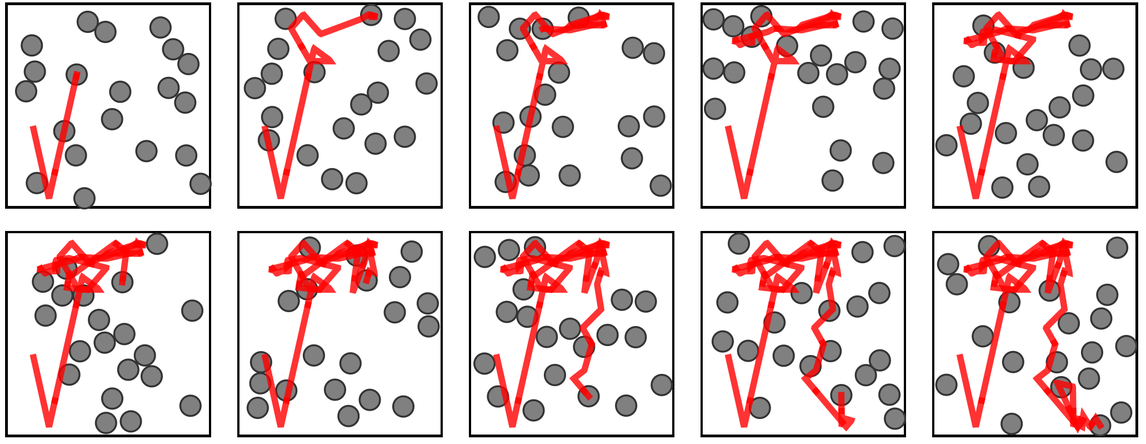✕ ```CloudGet["https://wolfr.am/177ScopeX"]; GraphicsGrid[ Partition[Rest[visualize2D[20, 2000, 10, 2, 200]], 5]]```

The molecule bounces around having a sequence of collisions, and moves according to what seems to be a random walk. But now let’s imagine we have a “gas demon” who’s “riding on a molecule”. And every time its molecule collides with another one, let’s imagine that the demon can make a decision about whether to stay with the molecule it’s already on, or to jump to the other molecule in the collision.

And now let’s say the demon is trying to “compute its way” across the box, deciding by looking at the history of the system which molecule it should hitch a ride on at each collision. Yes, the demon will have to do lots of computation. But the result will be that it can get itself transported across the system much faster than if it just stuck with one molecule. In other words, by using computation, it can “beat randomness” (and diffusion).

If we think of the collisions between hard spheres as events, we can construct a causal graph of their causal relationships: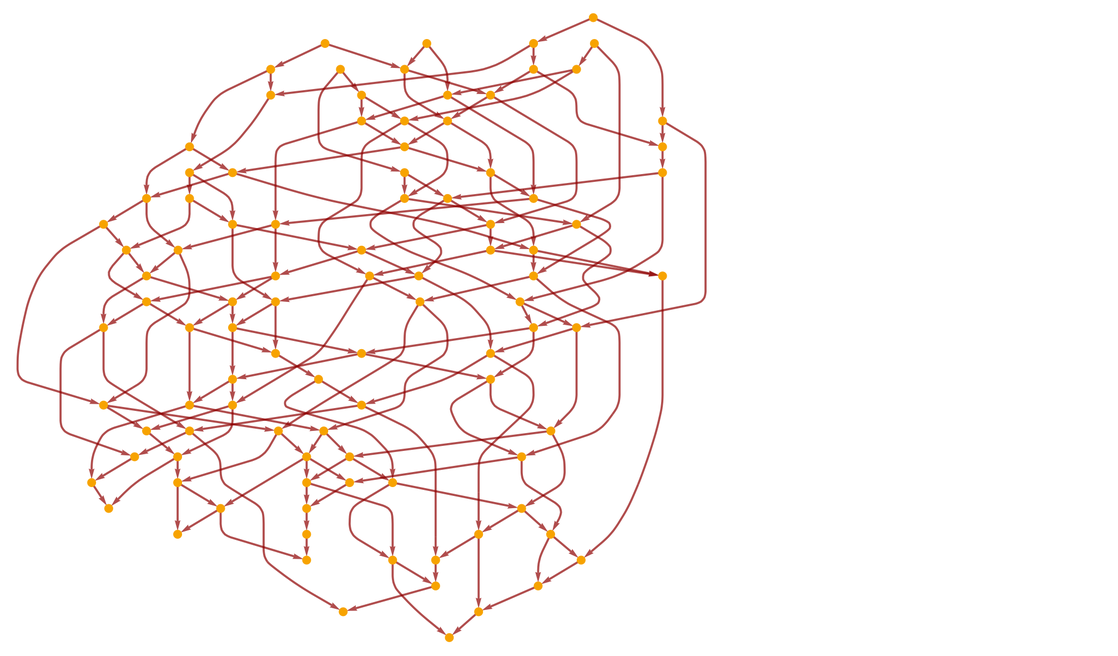✕ ```collisionsToCausalGraph[collisions_] := Module[{particles, instants, edges}, (*Extract particles from list of collisions*) particles = Union @@ collisions[[All, ;; 2]]; (*Generate causal graph from collisions. Construct a directed edge whenever an uninterrupted sequence of two \ collisions involves the same particle.*) edges = Catenate[Function[particle, instants = SplitBy[Select[ collisions, #[] === particle || #[] === particle &], Last]; Catenate[ BlockMap[DirectedEdge @@@ Tuples[#] &, instants, 2, 1]] ] /@ particles]; (*Finally, construct the graph*) Graph[DeleteDuplicates@edges, Sequence[ GraphLayout -> "LayeredDigraphEmbedding", AspectRatio -> 1/GoldenRatio, ImageSize -> Large]] ] genCausalGraph[num_, steps_, boxSize_, dimension_] := Module[{collisions, initialConditions, stepSize = .1, rad = 1.}, (*Choose initial conditions in N-dimensions*) initialConditions = N[{RandomReal[{-#, #} &@(boxSize - rad), {num, dimension}], RandomPoint[Ball[ConstantArray[0, dimension]], num]}]; collisions = Select[Rest[simulateCollisionsBox[ initialConditions, N[boxSize], N[stepSize], N[rad], steps][[ 2]]], #[] > Round[steps/5] &]; collisionsToCausalGraph[collisions] ] SeedRandom; hscg = Graph[ResourceFunction["WolframPhysicsProjectStyleData"][ "CausalGraph"]["Function"][genCausalGraph[20, 500, 10, 2]], AspectRatio -> .9]```

At each event there are two incoming causal edges and two outgoing ones, corresponding to the spheres involved in a particular collision. And we can think of what the demon is doing as having to choose at each node in the causal graph which outgoing edge to follow. Or, in other words, the demon is determining its path in the causal graph.

Just like for our models, we can construct a causal cone for the hard-sphere gas (here continuing for more steps)—and the path taken by the demon is restricted to not go outside this cone: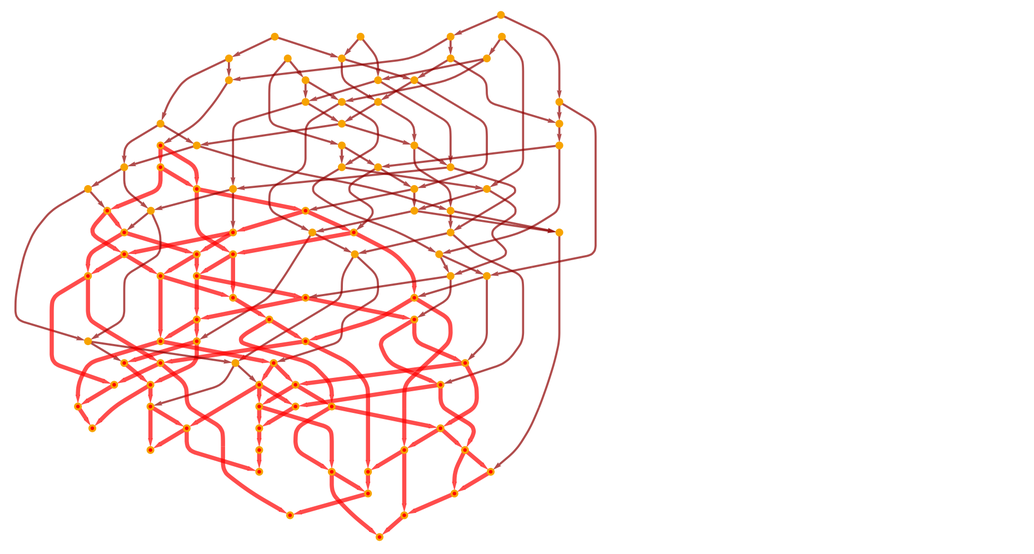✕ ```HighlightGraph[hscg, Style[Subgraph[hscg, VertexOutComponent[hscg, {SortBy[VertexList[hscg], Last][]}]], Red, Thick]]```

But also like for our models, the relationship between positions in the causal ball obtained from this causal cone, and actual spatial positions, is in general complicated. At least if we were operating in an infinite region (as opposed to a finite box), the border of the causal ball in the hard-sphere gas would just be a circle. But the point is that there are always “tendrils” that stick out, and if there’s a finite box, it’s even more complicated: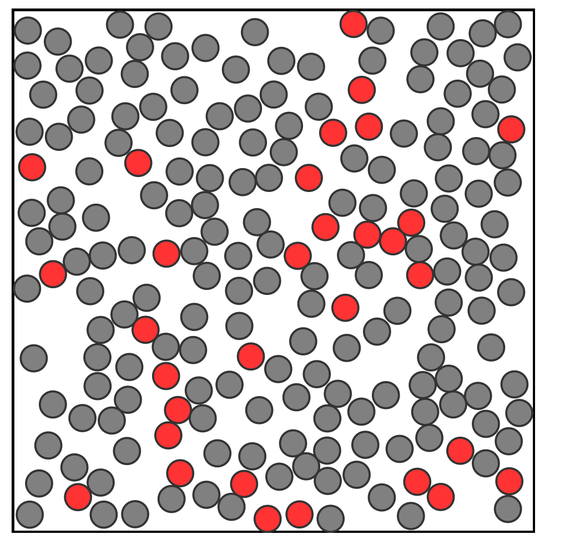✕ ```trajectories2D[num_, steps_, boxSize_] := Module[{initialConditions, traj, stepSize = .1, rad = 1}, (*Change seed for different behavior!*) SeedRandom; (*Randomly distributed positions with randomly distributed \ velcities*) initialConditions = N[{RandomReal[{-#, #} &@(boxSize - rad), {num, 2}], RandomPoint[Ball[ConstantArray[0, 2]], num]}]; (*Simulate a box, animate the results*) traj = simulateCollisionsBox[initialConditions, N[boxSize], N[stepSize], N[rad], steps][[1, All, 1]]\[Transpose] ] hcg = genCausalGraph[200, 500, 20, 2]; mems = Union[ Flatten[Take[#, 2] & /@ SortBy[VertexOutComponent[ hcg, {SortBy[VertexList[hcg], Last][]}], Last]]]; With[{boxSize = 20, rad = 1}, Graphics[ {{FaceForm[], EdgeForm[Black], Rectangle[{-boxSize, -boxSize}, {boxSize, boxSize}]}, MapIndexed[ Style[Disk[#, rad], EdgeForm[GrayLevel[.2]], If[MemberQ[mems, First[#2]], Lighter[Red, .2], Gray]] &, trajectories2D[200, 500, 20][[All, 500]]]}]]```

But the point is that if the demon can make a judicious choice of which “tendrils” to follow, it can move faster than the speed defined by the “average border” of the causal cone.

If our “hard-sphere gas” were made, for example, of idealized electronic turtles, each with a computer and sensors on board, it wouldn’t seem too difficult to have a “demon turtle”. Even if our “hard spheres” were the size of microorganisms, it wouldn’t seem surprising to have a “demon”. It’s harder to imagine for actual molecules or particles; there just doesn’t seem to be anywhere to “put the computation apparatus”. Though if we started thinking about cooperation among many different hard spheres then it begins to seem more plausible again. After all, perhaps we could set up a configuration of a group of hard spheres, whose evolution will do the computation we need.

OK, so what about the case of actual space in our models? In some ways it’s a more demanding situation: after all, every aspect of the internal structure of a space demon must—like everything else—be encoded in the structure of the spatial hypergraph.

There is much we don’t know yet. For example, if there are “transient space tunnels” formed, what regularities might they show? In a hard-sphere gas, especially in 2D, there are surprisingly long time correlations between spheres, associated with what amounts to collective “hydrodynamic” behavior. And we don’t know what similar phenomena might exist in the spatial hypergraphs in our models.

But then, of course, there is the question of how to actually construct “space demons” to take advantage of transient space tunnels. The Principle of Computational Equivalence has both good and bad news here. The bad news is that it implies that the evolution of the spatial hypergraph will show computational irreducibility—so it’ll take irreducible amounts of computational work to predict what it does. But the good news is that the dynamics of the hypergraph will be capable of universal computation, and can therefore in principle be “programmed” to do computations that could do whatever can be done to “figure out what will happen”.

The key question is then whether there are sufficient “pockets of computational reducibility” associated with space tunnels that we’ll be able to successfully exploit. We know that in the continuum limit there’s plenty of computational reducibility: that’s why our models can reproduce mathematical theories like general relativity and quantum mechanics.

But space tunnels aren’t a phenomenon of the usual continuum limit; they’re something different. We don’t know what a “mathematical theory of space tunnels” would be like. Conceivably, insofar as ordinary continuum behavior can be thought of as related to the central limit theorem and Gaussian distributions, a “theory of space tunnels” could have something to do with extreme value distributions. But most likely the mathematics—if it exists, and if we can even call it that—will be much more alien.

When we say that a gas can be characterized as having a certain temperature, we’re saying that we’re not going to describe anything about the specific motions of the molecules; we’re just going to say that they’re “random”, with some average speed. But as I mentioned above, in reality there are all sorts of detailed patterns and correlations in these motions. And while as a whole they will show computational irreducibility, it is inevitable that there will be pockets of computational reducibility too. We don’t know what they are—and perhaps if we did, we could even use some of them for technological purposes. (Right now, we pretty much only use the very organized motions of molecules that we call “mechanical work”.)

But now the challenge in creating a space demon is to find such pockets of reducibility in the underlying behavior of space. In a sense, much of the historical task of engineering has been to identify pockets of reducibility in our familiar physical world: circular motion, ferromagnetic alignment of spins, wave configurations of fields, etc. In any given case, we’ll never know how hard it’s going to be: the process of finding pockets of reducibility is itself a computationally irreducible process.

But let’s say we could construct a space demon. We don’t know what characteristics it would have. Would it let us create borders around a space tunnel that would allow some “standard material object” to pass through the tunnel? Or would it instead allow a space tunnel to be constructed that could only pass through some special kind of hypergraph structure—that we might even characterize (in a nod to science fiction) as a means of “subspace communication” (i.e. communication that’s making use of structures that lie “below” space as we usually experience it)?

## Quantum Effects

Most of what I’ve said about causal graphs, etc. so far has basically been classical. I’ve assumed that there’s in a sense just one thread of history for the universe. But the full story in our models—and in physics—is more complicated. Instead of there being a single theory of history, there’s a whole multiway graph that includes all the possible choices for how updating events can happen.

And in general instead of just having an ordinary causal cone, one really has a multiway causal cone—that in effect has extent not only in physical space but also in branchial space. And just as we have talked about selecting reference frames in spacetime, we also need to talk about selecting quantum observation frames in branchtime. And just as reference frames in spacetime give us a way to make sense of how events are organized in spacetime, and how we would observe or measure them there, so similarly quantum observation frames give us a way to make sense of how events are organized in branchtime, and what we would infer about them from quantum measurements.

In what we’ve said so far about space tunnels, we’re basically always assuming there’s a single thread of history involved. But really we should be talking about multiway causal cones, and tunnels that have extent both in physical space and branchial space, or, in other words, multispace tunnels.

We might imagine space tunnels are always “just fluctuations”, and that they’d be different on every “branch of history”. But a key point about multiway systems—and about multispace—is that they imply that we can expect coherence not only in physical space but also in branchial space, just as a “wave packet” is bounded both in physical and branchial space.

In our models, “vacuum fluctuations” in quantum mechanics and in the structure of space are intimately connected; in the end they are both just facets of the multiway causal graph. In ordinary quantum field theory one is used to virtual particles which individually have propagators (typically like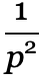) that imply they can show “virtual” faster-than-light effects. But we also know—as technically implemented in the commutation relations for field operators—that in the structure of standard quantum field theory there can be no real correlations “outside the light cone”. In our models, there can also be no correlations outside the (multiway) causal cone. But the whole issue is how projections of that multiway causal cone map onto geodesic balls representing distance in space.

So what does all this mean for space demons? That they actually need to be not just space demons, but multispace demons, operating not just in physical space, but also in branchial space, or in the space of quantum states. And, yes, this is yet more complicated, but it doesn’t in any obvious way change whether things are possible.

When we imagine a space demon identifying features of space that can form a space tunnel, we can expect that it’ll do this at a particular place in physical space. In other words, if we end up going faster than light, there’ll be a particular origination point in our physical space for our journey (or, in some science fiction terms, our “jump”). And it’s really no different for branchial space and multispace demons. A multispace tunnel will presumably have some location both in physical space and branchial space.

In the way we currently think about things, “going there” in branchial space basically means doing a certain quantum measurement—though causal invariance implies that in the end all quantum observers will agree about what happened (and e.g. that one successfully “went faster than light”).

It’s all quite complicated, and certainly far from completely worked out. And there’s another issue as well. The speed of light constrains maximum speeds in physical space. But in our models, there’s also the maximum entanglement speed, which constrains maximum speeds in branchial space. And just as we can imagine space tunnels providing ways to go “faster than c”, so also we can imagine multispace tunnels providing ways to go “faster than ζ”.

## Is It Possible? Can We Make It Work?

OK, so what’s the bottom line? Is it in principle possible to go faster than light? And if so, how can we actually do it?

I’m pretty sure that, yes, in principle it’s possible. In fact, as soon as one views space as having an underlying structure, and not just being a mathematical manifold “all the way down”, it’s pretty much inevitable. But it still requires essentially “hacking” space, and “reverse engineering” its structure to find features like “space tunnels” that one can use.

How is all this consistent with relativity, and its assumption of the absoluteness of the speed of light? Well, it isn’t. The phenomena and possibilities I’m describing here are ones that occur in the “substrate” below where relativity operates. It’s as if our standard physics—with relativity, etc.—are part of the “high-level operating system” of the universe. But what we’re talking here about doing is creating hacks down at the “machine code” level.

Put another way: relativity is something that arises in our models as a large-scale limit, when one’s averaged out all the underlying details. But the whole point here is to somehow leverage and “line up” those underlying details, so they produce the effects we’re interested in. But when we look at the whole “bulk” universe, and the full large-scale limit, anything we might be able to do at the level of the details will seem infinitesimal—and won’t affect our overall conclusion that relativity is a feature of the general physics of the universe.

Now of course, even though something may in principle be possible, that doesn’t mean it can be done in practice. Maybe it’s fairly easy to go a tiny distance faster than light, but to scale up to anything substantial requires resources beyond what we—or even the universe—could ever muster. And, yes, as I discussed, that is a possibility. Because in a sense what we have to do is to “beat computational irreducibility” in the evolution of space. And in the abstract there is no way to tell how hard this might be.

Let’s say we have the general objective of “going faster than light”. There will be an immense (and probably infinite) number of detailed ways we could imagine achieving this. And in general there will be no upper bound on the amount of computation needed for any one of them. So if we ask “Will any of them work?”, that’ll be formally undecidable. If we find one that we can show works, great. But we could in principle have to go on testing things forever, never being sure that nothing can work.

And, yes, this means that even though we might know the final underlying rule for physics, we still might fundamentally never be sure whether it’s possible to go faster than light. We might have successfully “reduced physics to mathematics”, but then we still have all the issues of mathematics—like Gödel’s theorem—to contend with. And just as Gödel’s theorem tells us there’s no upper bound on the lengths of proofs we might need in arithmetic, so now we’re in a situation where there’s no upper bound on the “complexity of the process” that we might need in physics to establish whether it’s possible to go faster than light.

Still, just because something is in general undecidable, it doesn’t mean we won’t be able to figure it out. Maybe we’ll have to give up on transporting ordinary material faster than light, and we’ll only be dealing with some specially crafted form of information. But there’s no reason to think that with an objective as broad as “somehow go faster than light” that we won’t be able to, in effect, find some pocket of computational reducibility that makes it possible for us to do it.

And the fact is that the history of engineering is full of cases where an initial glimmer of possibility was eventually turned into large-scale technological success. “Can one achieve heavier-than-air flight?” There were detailed hydrodynamic effects, and there were pieces of what later became control theory. And eventually there was an engineering construction that made it work.

It’s hard to predict the process of engineering innovation. We’ve known the basic physics around controlled nuclear fusion for more than half a century. But when will we actually make it work as an engineering reality? Right now the idea of hacking space to go faster than light seems far away from anything we could in practice do. But we have no idea how high—or low—the barrier actually is.

Might it require having our own little black hole? Or might it be something that just requires putting together things we already have in just the right way? Not long ago it was completely unclear that we could “beat the uncertainty principle” enough to measure gravitational waves. Or that we could build an atomic force microscope that could move individual atoms around. Or that we could form a physical Bose–Einstein condensate. But in cases like these it turned out that we already had the “raw materials” we needed; we just had to figure out what to do with them.

A few years ago, when I was trying to make up fictional science for the movie Arrival, I thought a little about how a present-day physicist might think about the mechanism for an interstellar spacecraft that showed up one day. It was before our current models, but I had already thought a lot about the potential discrete structure of spacetime. And the best fictional idea I came up with then about how to “access it” was through some kind of “gravitational laser”. Gravitons, like photons, are bosons that can in principle form quantum condensates. And at least at the level of a made-for-a-movie whiteboard I figured out a little of how this might work.

But from what we know now, there are other ideas. Perhaps the best analogy—at least for “communication” if not “travel”—is that one’s trying to get a signal “through a complex medium” as efficiently as possible. And that’s of course been the basic problem forever in communications systems based on electrical, electromagnetic or optical processes.

Often it’s been claimed that there’s some fundamental limit, say to transmission rates. But then an engineering solution will be found that overcomes it. And actually the typical mechanism used is a little like our demons. If one’s signal is going to be degraded by “noise”, figure out how to predict the noise, then “sculpt” the process of transmission around it. In 5G technology for example, there’s even an explicit concept of “pilot signals” that continually probe the local radio environment so that actual communication signals can be formed in just the right ways.

But, OK, let’s say there is a practical way to go faster than light, or at least to send signals faster than light. Then why aren’t we seeing lots of more-advanced-than-us extraterrestrial intelligences doing this all over the universe? Maybe we just have to figure out the right engineering trick and then we’ll immediately be able to tap into a universe-scale conversation. And while it’s fun to imagine just how wild the social network of the universe might get, I think there’s a fundamental problem here (even beyond the “what’s really the use case?”). Let’s say we can see processes that correspond to faster-than-light communication. Are they part of a “conversation”, “saying” something meaningful? Or are they just “physical processes” that are going on?

Well, of course, anything that happens in the universe is, essentially by definition, a “physical process”. So then we might start talking about whether what we’re seeing is an “intentionally created” physical process, or one that’s just “happening naturally”. But—as I’ve written extensively about elsewhere—it’s a slippery slope. And the Principle of Computational Equivalence basically tells us that in the end we’ll never be able to distinguish the “intelligent” from the “merely computational”, or, given our model of physics, the “merely physical”—at least unless what we’re seeing is aligned in detail with our particular human ways of thinking.

At the outset we might have imagined that going faster than light was an open-and-shut case, and that physics had basically proved that—despite a few seemingly pathological examples in general relativity—it isn’t possible. I hope what’s become clear here is that actually the opposite is true. In our models of physics, going faster than light is almost inevitably possible in principle. But to actually do it requires engineering that may be irreducibly difficult.

But maybe it’s like in 1687 when a then-new model of physics implied that artificial satellites might be possible. After 270 years of steady engineering progress, there they were. And so it may be with going faster than light. Our models now suggest it’s possible. But whether the engineering required can be done in ten, a hundred, a thousand, a million or a billion years we don’t know. But maybe at least there’s now a path to turn yet another “pure-science-fiction impossibility” into reality.

## A Few Questions

My talk at NASA generated many questions. Here are a few answers.

### What about warp bubbles and the Alcubierre metric?

Warp bubbles are a clever way to get something a bit like faster-than-light travel in ordinary general relativity. The basic idea is to set up a solution to Einstein’s equations in which space is “rapidly contracting” in front of a “bubble region”, and expanding behind it: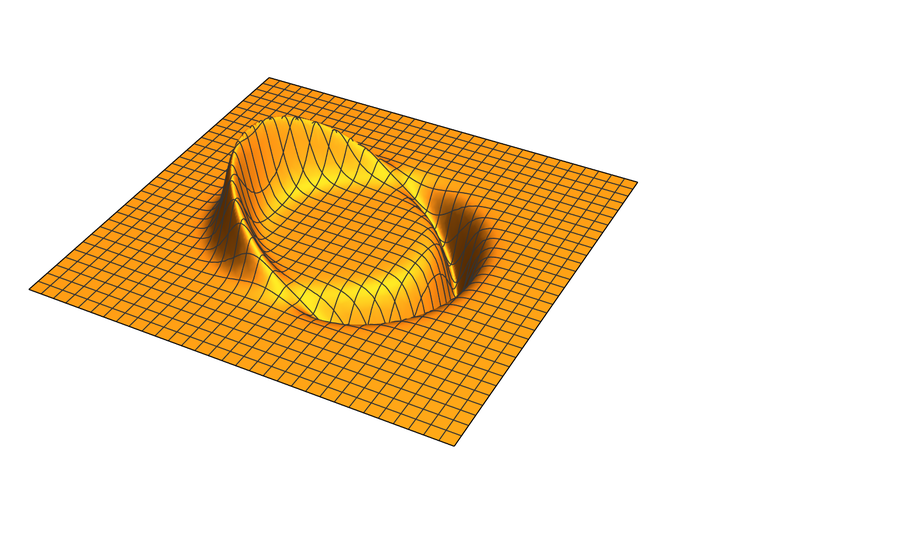✕ ```Cell[CellGroupData[{Cell[BoxData[ RowBox[{ RowBox[{"expansion", "=", RowBox[{ RowBox[{"(", RowBox[{ InterpretationBox[ StyleBox["\[Sigma]", ShowAutoStyles->False, AutoSpacing->False], \$CellContext`\[Sigma], Editable->False], " ", RowBox[{ InterpretationBox[ StyleBox["Coth", ShowAutoStyles->False, AutoSpacing->False], Coth, Editable->False], "[", RowBox[{ InterpretationBox[ StyleBox["R", ShowAutoStyles->False, AutoSpacing->False], \$CellContext`R, Editable->False], " ", InterpretationBox[ StyleBox["\[Sigma]", ShowAutoStyles->False, AutoSpacing->False], \$CellContext`\[Sigma], Editable->False]}], "]"}], " ", RowBox[{"(", RowBox[{ RowBox[{"-", SuperscriptBox[ RowBox[{ InterpretationBox[ StyleBox["Sech", ShowAutoStyles->False, AutoSpacing->False], Sech, Editable->False], "[", RowBox[{ InterpretationBox[ StyleBox["\[Sigma]", ShowAutoStyles->False, AutoSpacing->False], \$CellContext`\[Sigma], Editable->False], " ", RowBox[{"(", RowBox[{ RowBox[{"-", InterpretationBox[ StyleBox["R", ShowAutoStyles->False, AutoSpacing->False], \$CellContext`R, Editable->False]}], "+", SqrtBox[ RowBox[{ SuperscriptBox[ RowBox[{"(", RowBox[{ InterpretationBox[ StyleBox["x", ShowAutoStyles->False, AutoSpacing->False], \$CellContext`x[], Editable->False], "-", RowBox[{ InterpretationBox[ StyleBox["xs", ShowAutoStyles->False, AutoSpacing->False], \$CellContext`xs, Editable->False], "[", InterpretationBox[ StyleBox["t", ShowAutoStyles->False, AutoSpacing->False], \$CellContext`t[], Editable->False], "]"}]}], ")"}], "2"], "+", SuperscriptBox[ InterpretationBox[ StyleBox["y", ShowAutoStyles->False, AutoSpacing->False], \$CellContext`y[], Editable->False], "2"], "+", SuperscriptBox[ InterpretationBox[ StyleBox["z", ShowAutoStyles->False, AutoSpacing->False], \$CellContext`z[], Editable->False], "2"]}]]}], ")"}]}], "]"}], "2"]}], "+", SuperscriptBox[ RowBox[{ InterpretationBox[ StyleBox["Sech", ShowAutoStyles->False, AutoSpacing->False], Sech, Editable->False], "[", RowBox[{ InterpretationBox[ StyleBox["\[Sigma]", ShowAutoStyles->False, AutoSpacing->False], \$CellContext`\[Sigma], Editable->False], " ", RowBox[{"(", RowBox[{ InterpretationBox[ StyleBox["R", ShowAutoStyles->False, AutoSpacing->False], \$CellContext`R, Editable->False], "+", SqrtBox[ RowBox[{ SuperscriptBox[ RowBox[{"(", RowBox[{ InterpretationBox[ StyleBox["x", ShowAutoStyles->False, AutoSpacing->False], \$CellContext`x[], Editable->False], "-", RowBox[{ InterpretationBox[ StyleBox["xs", ShowAutoStyles->False, AutoSpacing->False], \$CellContext`xs, Editable->False], "[", InterpretationBox[ StyleBox["t", ShowAutoStyles->False, AutoSpacing->False], \$CellContext`t[], Editable->False], "]"}]}], ")"}], "2"], "+", SuperscriptBox[ InterpretationBox[ StyleBox["y", ShowAutoStyles->False, AutoSpacing->False], \$CellContext`y[], Editable->False], "2"], "+", SuperscriptBox[ InterpretationBox[ StyleBox["z", ShowAutoStyles->False, AutoSpacing->False], \$CellContext`z[], Editable->False], "2"]}]]}], ")"}]}], "]"}], "2"]}], ")"}], " ", RowBox[{"(", RowBox[{ InterpretationBox[ StyleBox["x", ShowAutoStyles->False, AutoSpacing->False], \$CellContext`x[], Editable->False], "-", RowBox[{ InterpretationBox[ StyleBox["xs", ShowAutoStyles->False, AutoSpacing->False], \$CellContext`xs, Editable->False], "[", InterpretationBox[ StyleBox["t", ShowAutoStyles->False, AutoSpacing->False], \$CellContext`t[], Editable->False], "]"}]}], ")"}], " ", RowBox[{ SuperscriptBox[ InterpretationBox[ StyleBox["xs", ShowAutoStyles->False, AutoSpacing->False], \$CellContext`xs, Editable->False], "\[Prime]", MultilineFunction->None], "[", InterpretationBox[ StyleBox["t", ShowAutoStyles->False, AutoSpacing->False], \$CellContext`t[], Editable->False], "]"}]}], ")"}], "/", RowBox[{"(", SqrtBox[ RowBox[{ SuperscriptBox[ InterpretationBox[ StyleBox["x", ShowAutoStyles->False, AutoSpacing->False], \$CellContext`x[], Editable->False], "2"], "-", RowBox[{"2", " ", InterpretationBox[ StyleBox["x", ShowAutoStyles->False, AutoSpacing->False], \$CellContext`x[], Editable->False], " ", RowBox[{ InterpretationBox[ StyleBox["xs", ShowAutoStyles->False, AutoSpacing->False], \$CellContext`xs, Editable->False], "[", InterpretationBox[ StyleBox["t", ShowAutoStyles->False, AutoSpacing->False], \$CellContext`t[], Editable->False], "]"}]}], "+", SuperscriptBox[ RowBox[{ InterpretationBox[ StyleBox["xs", ShowAutoStyles->False, AutoSpacing->False], \$CellContext`xs, Editable->False], "[", InterpretationBox[ StyleBox["t", ShowAutoStyles->False, AutoSpacing->False], \$CellContext`t[], Editable->False], "]"}], "2"], "+", SuperscriptBox[ InterpretationBox[ StyleBox["y", ShowAutoStyles->False, AutoSpacing->False], \$CellContext`y[], Editable->False], "2"], "+", SuperscriptBox[ InterpretationBox[ StyleBox["z", ShowAutoStyles->False, AutoSpacing->False], \$CellContext`z[], Editable->False], "2"]}]], ")"}]}]}], ";"}]], "Input"], Cell[BoxData[ RowBox[{ RowBox[{ RowBox[{"expansionF", "[", RowBox[{"x_", ",", "\[Rho]_"}], "]"}], "=", RowBox[{ RowBox[{ RowBox[{"expansion", "/.", RowBox[{"{", RowBox[{ RowBox[{"\[Sigma]", "\[Rule]", "8"}], ",", RowBox[{"R", "\[Rule]", "1"}]}], "}"}]}], "/.", RowBox[{"{", RowBox[{"xs", "\[Rule]", RowBox[{"Function", "[", RowBox[{"t", ",", "t"}], "]"}]}], "}"}]}], "/.", RowBox[{"{", RowBox[{ RowBox[{ RowBox[{"t", "[", "]"}], "\[Rule]", "0"}], ",", RowBox[{ RowBox[{"x", "[", "]"}], "\[Rule]", "x"}], ",", RowBox[{ RowBox[{ RowBox[{"y", "[", "]"}], "^", "2"}], "\[Rule]", RowBox[{ RowBox[{"\[Rho]", "^", "2"}], "-", RowBox[{ RowBox[{"z", "[", "]"}], "^", "2"}]}]}]}], "}"}]}]}], ";"}]], "Input"], Cell[BoxData[ RowBox[{"Plot3D", "[", RowBox[{ RowBox[{"expansionF", "[", RowBox[{"x", ",", "\[Rho]"}], "]"}], ",", RowBox[{"{", RowBox[{"x", ",", RowBox[{"-", "2"}], ",", "2"}], "}"}], ",", RowBox[{"{", RowBox[{"\[Rho]", ",", RowBox[{"-", "2"}], ",", "2"}], "}"}], ",", RowBox[{"PlotRange", "\[Rule]", "All"}], ",", RowBox[{"MaxRecursion", "\[Rule]", "5"}], ",", RowBox[{"Boxed", "\[Rule]", "False"}], ",", RowBox[{"Axes", "\[Rule]", "None"}], ",", RowBox[{"Mesh", "\[Rule]", "30"}]}], "]"}]], "Input"] }, Open ]]```

To maintain this configuration, one needs negative mass on each side of the bubble: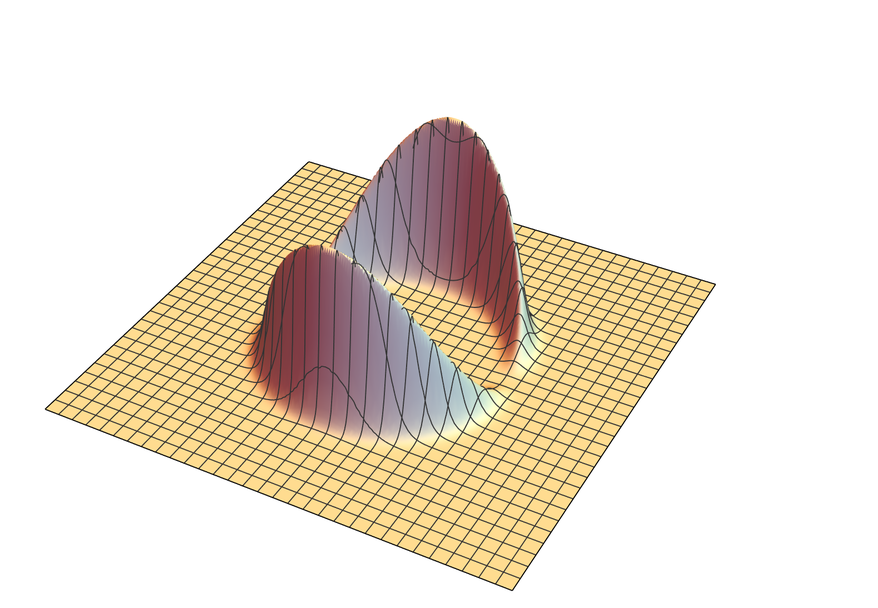✕ ```Cell[CellGroupData[{Cell[BoxData[ RowBox[{ RowBox[{"density", "=", RowBox[{"-", RowBox[{"(", RowBox[{ RowBox[{"(", RowBox[{ SuperscriptBox[ InterpretationBox[ StyleBox["\[Sigma]", ShowAutoStyles->False, AutoSpacing->False], \$CellContext`\[Sigma], Editable->False], "2"], " ", SuperscriptBox[ RowBox[{ InterpretationBox[ StyleBox["Cosh", ShowAutoStyles->False, AutoSpacing->False], Cosh, Editable->False], "[", RowBox[{ InterpretationBox[ StyleBox["R", ShowAutoStyles->False, AutoSpacing->False], \$CellContext`R, Editable->False], " ", InterpretationBox[ StyleBox["\[Sigma]", ShowAutoStyles->False, AutoSpacing->False], \$CellContext`\[Sigma], Editable->False]}], "]"}], "4"], " ", SuperscriptBox[ RowBox[{ InterpretationBox[ StyleBox["Sech", ShowAutoStyles->False, AutoSpacing->False], Sech, Editable->False], "[", RowBox[{ InterpretationBox[ StyleBox["\[Sigma]", ShowAutoStyles->False, AutoSpacing->False], \$CellContext`\[Sigma], Editable->False], " ", RowBox[{"(", RowBox[{ RowBox[{"-", InterpretationBox[ StyleBox["R", ShowAutoStyles->False, AutoSpacing->False], \$CellContext`R, Editable->False]}], "+", SqrtBox[ RowBox[{ SuperscriptBox[ InterpretationBox[ StyleBox["x", ShowAutoStyles->False, AutoSpacing->False], \$CellContext`x[], Editable->False], "2"], "-", RowBox[{"2", " ", InterpretationBox[ StyleBox["x", ShowAutoStyles->False, AutoSpacing->False], \$CellContext`x[], Editable->False], " ", RowBox[{ InterpretationBox[ StyleBox["xs", ShowAutoStyles->False, AutoSpacing->False], \$CellContext`xs, Editable->False], "[", InterpretationBox[ StyleBox["t", ShowAutoStyles->False, AutoSpacing->False], \$CellContext`t[], Editable->False], "]"}]}], "+", SuperscriptBox[ RowBox[{ InterpretationBox[ StyleBox["xs", ShowAutoStyles->False, AutoSpacing->False], \$CellContext`xs, Editable->False], "[", InterpretationBox[ StyleBox["t", ShowAutoStyles->False, AutoSpacing->False], \$CellContext`t[], Editable->False], "]"}], "2"], "+", SuperscriptBox[ InterpretationBox[ StyleBox["y", ShowAutoStyles->False, AutoSpacing->False], \$CellContext`y[], Editable->False], "2"], "+", SuperscriptBox[ InterpretationBox[ StyleBox["z", ShowAutoStyles->False, AutoSpacing->False], \$CellContext`z[], Editable->False], "2"]}]]}], ")"}]}], "]"}], "4"], " ", SuperscriptBox[ RowBox[{ InterpretationBox[ StyleBox["Sech", ShowAutoStyles->False, AutoSpacing->False], Sech, Editable->False], "[", RowBox[{ InterpretationBox[ StyleBox["\[Sigma]", ShowAutoStyles->False, AutoSpacing->False], \$CellContext`\[Sigma], Editable->False], " ", RowBox[{"(", RowBox[{ InterpretationBox[ StyleBox["R", ShowAutoStyles->False, AutoSpacing->False], \$CellContext`R, Editable->False], "+", SqrtBox[ RowBox[{ SuperscriptBox[ InterpretationBox[ StyleBox["x", ShowAutoStyles->False, AutoSpacing->False], \$CellContext`x[], Editable->False], "2"], "-", RowBox[{"2", " ", InterpretationBox[ StyleBox["x", ShowAutoStyles->False, AutoSpacing->False], \$CellContext`x[], Editable->False], " ", RowBox[{ InterpretationBox[ StyleBox["xs", ShowAutoStyles->False, AutoSpacing->False], \$CellContext`xs, Editable->False], "[", InterpretationBox[ StyleBox["t", ShowAutoStyles->False, AutoSpacing->False], \$CellContext`t[], Editable->False], "]"}]}], "+", SuperscriptBox[ RowBox[{ InterpretationBox[ StyleBox["xs", ShowAutoStyles->False, AutoSpacing->False], \$CellContext`xs, Editable->False], "[", InterpretationBox[ StyleBox["t", ShowAutoStyles->False, AutoSpacing->False], \$CellContext`t[], Editable->False], "]"}], "2"], "+", SuperscriptBox[ InterpretationBox[ StyleBox["y", ShowAutoStyles->False, AutoSpacing->False], \$CellContext`y[], Editable->False], "2"], "+", SuperscriptBox[ InterpretationBox[ StyleBox["z", ShowAutoStyles->False, AutoSpacing->False], \$CellContext`z[], Editable->False], "2"]}]]}], ")"}]}], "]"}], "4"], " ", SuperscriptBox[ RowBox[{ InterpretationBox[ StyleBox["Sinh", ShowAutoStyles->False, AutoSpacing->False], Sinh, Editable->False], "[", RowBox[{"2", " ", InterpretationBox[ StyleBox["\[Sigma]", ShowAutoStyles->False, AutoSpacing->False], \$CellContext`\[Sigma], Editable->False], " ", SqrtBox[ RowBox[{ SuperscriptBox[ InterpretationBox[ StyleBox["x", ShowAutoStyles->False, AutoSpacing->False], \$CellContext`x[], Editable->False], "2"], "-", RowBox[{"2", " ", InterpretationBox[ StyleBox["x", ShowAutoStyles->False, AutoSpacing->False], \$CellContext`x[], Editable->False], " ", RowBox[{ InterpretationBox[ StyleBox["xs", ShowAutoStyles->False, AutoSpacing->False], \$CellContext`xs, Editable->False], "[", InterpretationBox[ StyleBox["t", ShowAutoStyles->False, AutoSpacing->False], \$CellContext`t[], Editable->False], "]"}]}], "+", SuperscriptBox[ RowBox[{ InterpretationBox[ StyleBox["xs", ShowAutoStyles->False, AutoSpacing->False], \$CellContext`xs, Editable->False], "[", InterpretationBox[ StyleBox["t", ShowAutoStyles->False, AutoSpacing->False], \$CellContext`t[], Editable->False], "]"}], "2"], "+", SuperscriptBox[ InterpretationBox[ StyleBox["y", ShowAutoStyles->False, AutoSpacing->False], \$CellContext`y[], Editable->False], "2"], "+", SuperscriptBox[ InterpretationBox[ StyleBox["z", ShowAutoStyles->False, AutoSpacing->False], \$CellContext`z[], Editable->False], "2"]}]]}], "]"}], "2"], " ", RowBox[{"(", RowBox[{ SuperscriptBox[ InterpretationBox[ StyleBox["y", ShowAutoStyles->False, AutoSpacing->False], \$CellContext`y[], Editable->False], "2"], "+", SuperscriptBox[ InterpretationBox[ StyleBox["z", ShowAutoStyles->False, AutoSpacing->False], \$CellContext`z[], Editable->False], "2"]}], ")"}], " ", SuperscriptBox[ RowBox[{ SuperscriptBox[ InterpretationBox[ StyleBox["xs", ShowAutoStyles->False, AutoSpacing->False], \$CellContext`xs, Editable->False], "\[Prime]", MultilineFunction->None], "[", InterpretationBox[ StyleBox["t", ShowAutoStyles->False, AutoSpacing->False], \$CellContext`t[], Editable->False], "]"}], "2"]}], ")"}], "/", RowBox[{"(", RowBox[{"4", " ", RowBox[{"(", RowBox[{ SuperscriptBox[ InterpretationBox[ StyleBox["x", ShowAutoStyles->False, AutoSpacing->False], \$CellContext`x[], Editable->False], "2"], "-", RowBox[{"2", " ", InterpretationBox[ StyleBox["x", ShowAutoStyles->False, AutoSpacing->False], \$CellContext`x[], Editable->False], " ", RowBox[{ InterpretationBox[ StyleBox["xs", ShowAutoStyles->False, AutoSpacing->False], \$CellContext`xs, Editable->False], "[", InterpretationBox[ StyleBox["t", ShowAutoStyles->False, AutoSpacing->False], \$CellContext`t[], Editable->False], "]"}]}], "+", SuperscriptBox[ RowBox[{ InterpretationBox[ StyleBox["xs", ShowAutoStyles->False, AutoSpacing->False], \$CellContext`xs, Editable->False], "[", InterpretationBox[ StyleBox["t", ShowAutoStyles->False, AutoSpacing->False], \$CellContext`t[], Editable->False], "]"}], "2"], "+", SuperscriptBox[ InterpretationBox[ StyleBox["y", ShowAutoStyles->False, AutoSpacing->False], \$CellContext`y[], Editable->False], "2"], "+", SuperscriptBox[ InterpretationBox[ StyleBox["z", ShowAutoStyles->False, AutoSpacing->False], \$CellContext`z[], Editable->False], "2"]}], ")"}]}], ")"}]}], ")"}]}]}], ";"}]], "Input"], Cell[BoxData[ RowBox[{ RowBox[{"densityF", "[", RowBox[{"x_", ",", "\[Rho]_"}], "]"}], "=", RowBox[{ RowBox[{ RowBox[{"density", "/.", RowBox[{"{", RowBox[{ RowBox[{"\[Sigma]", "\[Rule]", "8"}], ",", RowBox[{"R", "\[Rule]", "1"}]}], "}"}]}], "/.", RowBox[{"{", RowBox[{"xs", "\[Rule]", RowBox[{"Function", "[", RowBox[{"t", ",", "t"}], "]"}]}], "}"}]}], "/.", RowBox[{"{", RowBox[{ RowBox[{ RowBox[{"t", "[", "]"}], "\[Rule]", "0"}], ",", RowBox[{ RowBox[{"x", "[", "]"}], "\[Rule]", "x"}], ",", RowBox[{ RowBox[{ RowBox[{"y", "[", "]"}], "^", "2"}], "\[Rule]", RowBox[{ RowBox[{"\[Rho]", "^", "2"}], "-", RowBox[{ RowBox[{"z", "[", "]"}], "^", "2"}]}]}]}], "}"}]}]}]], "Input"], Cell[BoxData[ RowBox[{"-", FractionBox[ RowBox[{"16", " ", SuperscriptBox["\[Rho]", "2"], " ", SuperscriptBox[ RowBox[{"Cosh", "[", "8", "]"}], "4"], " ", SuperscriptBox[ RowBox[{"Sech", "[", RowBox[{"8", " ", RowBox[{"(", RowBox[{ RowBox[{"-", "1"}], "+", SqrtBox[ RowBox[{ SuperscriptBox["x", "2"], "+", SuperscriptBox["\[Rho]", "2"]}]]}], ")"}]}], "]"}], "4"], " ", SuperscriptBox[ RowBox[{"Sech", "[", RowBox[{"8", " ", RowBox[{"(", RowBox[{"1", "+", SqrtBox[ RowBox[{ SuperscriptBox["x", "2"], "+", SuperscriptBox["\[Rho]", "2"]}]]}], ")"}]}], "]"}], "4"], " ", SuperscriptBox[ RowBox[{"Sinh", "[", RowBox[{"16", " ", SqrtBox[ RowBox[{ SuperscriptBox["x", "2"], "+", SuperscriptBox["\[Rho]", "2"]}]]}], "]"}], "2"]}], RowBox[{ SuperscriptBox["x", "2"], "+", SuperscriptBox["\[Rho]", "2"]}]]}]], "Output", CellGroupingRules->{"GroupTogetherGrouping", 10001.}, CellChangeTimes->{3.810596452088109*^9, 3.810596636896936*^9, 3.810599942079987*^9}, CellLabel->"Out="], Cell[BoxData[ RowBox[{"Plot3D", "[", RowBox[{ RowBox[{"-", RowBox[{"densityF", "[", RowBox[{"x", ",", "\[Rho]"}], "]"}]}], ",", RowBox[{"{", RowBox[{"x", ",", RowBox[{"-", "2"}], ",", "2"}], "}"}], ",", RowBox[{"{", RowBox[{"\[Rho]", ",", RowBox[{"-", "2"}], ",", "2"}], "}"}], ",", RowBox[{"PlotRange", "\[Rule]", "All"}], ",", RowBox[{"MaxRecursion", "\[Rule]", "5"}], ",", RowBox[{"Boxed", "\[Rule]", "False"}], ",", RowBox[{"Axes", "\[Rule]", "None"}], ",", RowBox[{"Mesh", "\[Rule]", "30"}], ",", RowBox[{"PlotStyle", "\[Rule]", "LightYellow"}]}], "]"}]], "Input"] }, Open ]]```

In a sense it’s like an asymmetric local analog of the expansion of the universe. Inside the bubble space is flat. But other parts of the universe are approaching or receding as a result of the contraction and expansion of space. And in fact this is happening so rapidly that (1) the bubble is effectively moving faster than light relative to the rest of the universe, and (2) there’s an event horizon around the bubble, so nothing can go in or out.

It’s rather easy to make a toy version of this within our models; here’s the corresponding causal graph: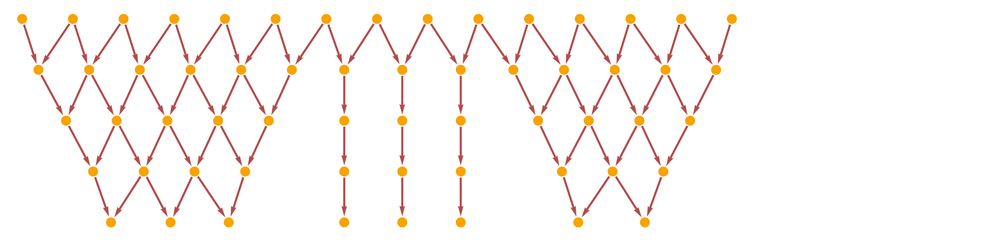✕ ```Graph[ResourceFunction["SubstitutionSystemCausalGraph"][ ResourceFunction["SubstitutionSystemCausalEvolution"][{"xo" -> "ox", "Xo" -> "Xo", "oX" -> "oX"}, "xoxoxoxoxoxooXoXoXxoxoxoxoxoxo", 5], "CausalGraph" -> True, "ColorTable" -> (LightGray &)], GraphLayout -> "LayeredDigraphEmbedding"]```

“Reconstructions of space” will then show that “parts of space” can “slip past others”, “as fast as they want”—but without causal interaction. Our space demon / space tunnel setup is rather different: there are no horizons involved; the whole point is to trace causal connections, but then to see how these map onto space.